# RD Sharma Solutions for Class 6 Maths Chapter 2: Playing with Numbers

The solutions are designed by experts in Mathematics having vast knowledge about the concepts. The students can make use of the solutions PDF in order to score well in the exam. RD Sharma Solutions contain answers to the chapter wise problems based on the latest CBSE syllabus. The experts mainly create the solutions after conducting extensive research on every topic to make them easy for the students.

The PDF can be used by the students as a reference material, which improves their problem solving abilities and to appear for the exam with confidence. The problems are solved in a comprehensive manner in order to make the answers easily understandable by the students. RD Sharma Solutions for Class 6 Maths Chapter 2 Playing with Numbers are provided here.

## RD Sharma Solutions for Class 6 Maths Chapter 2: Playing with Numbers Download PDF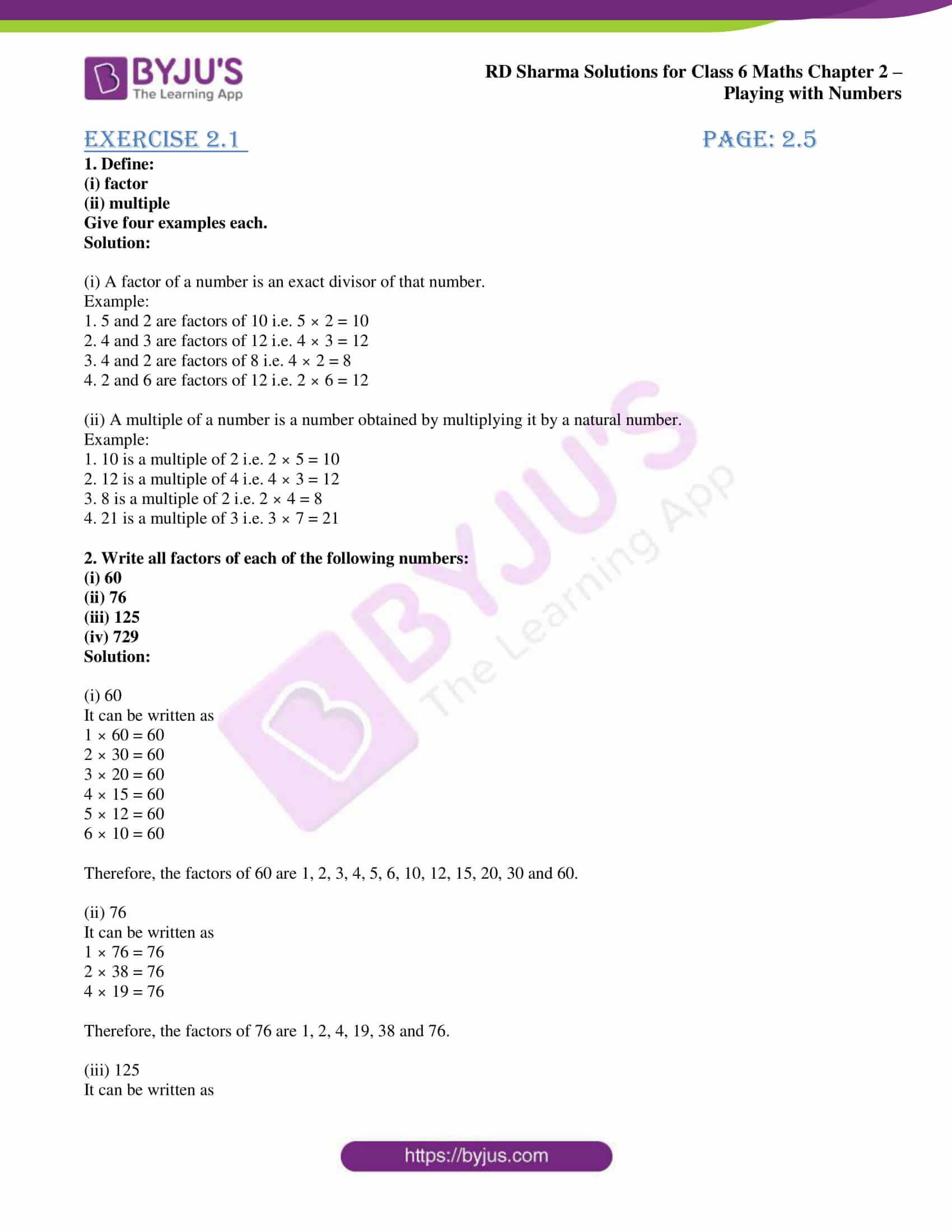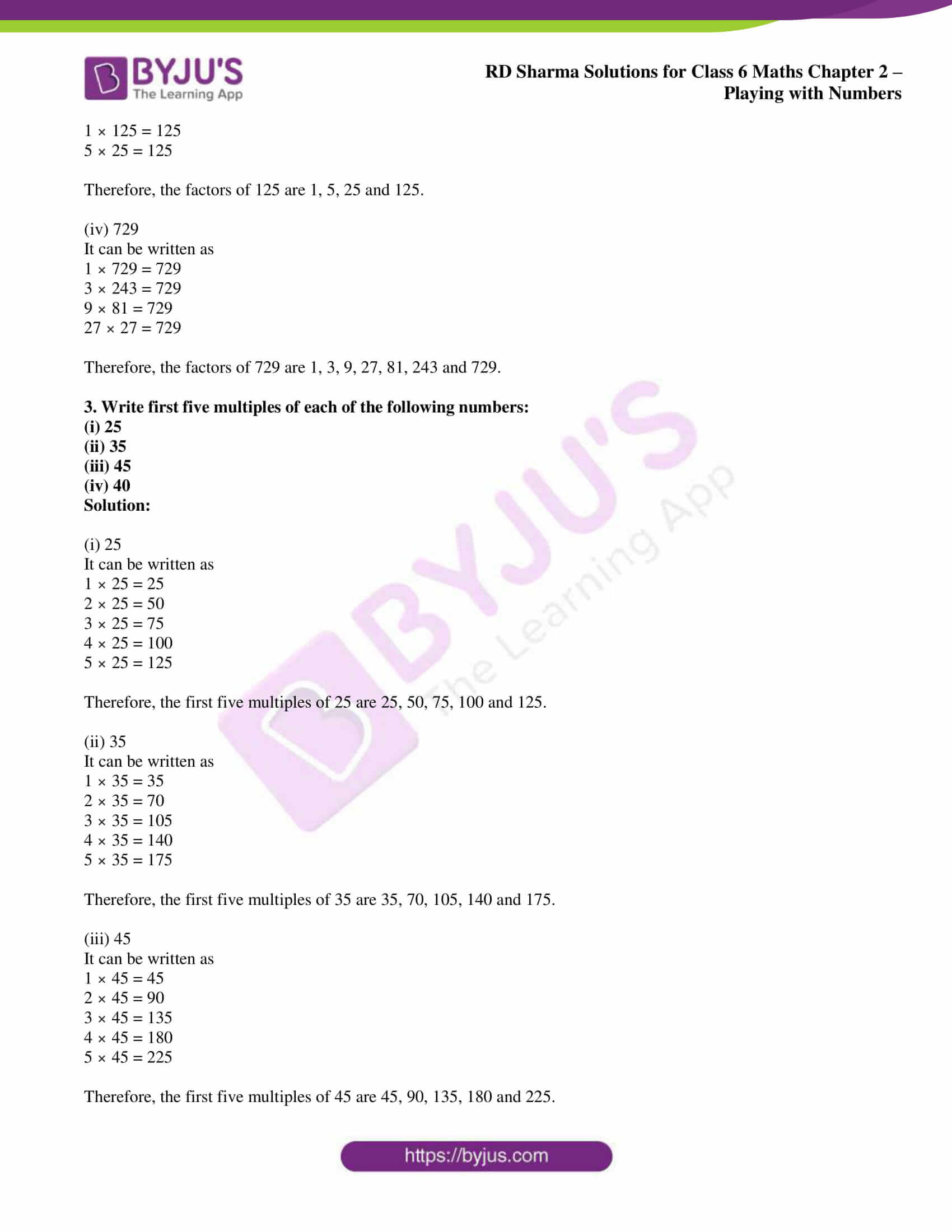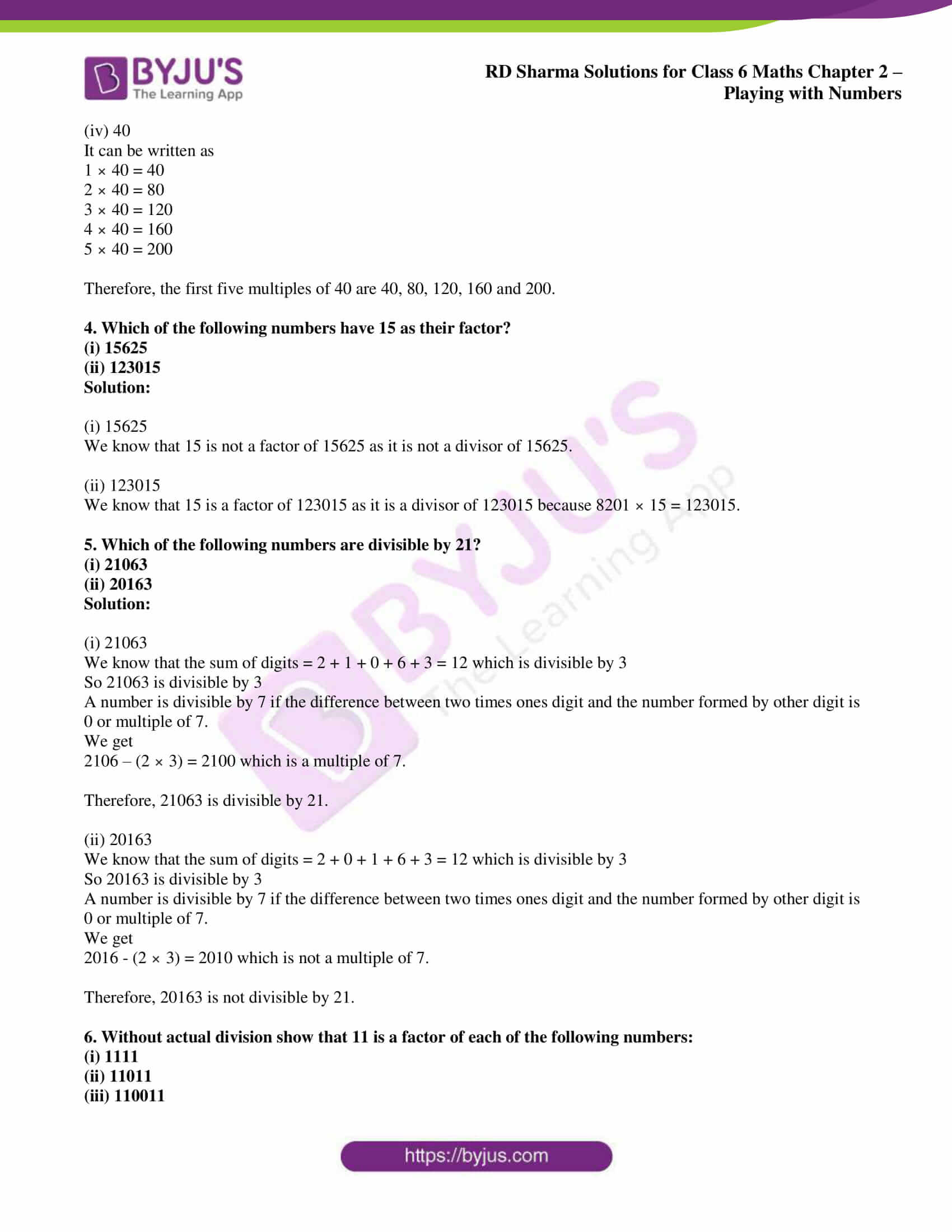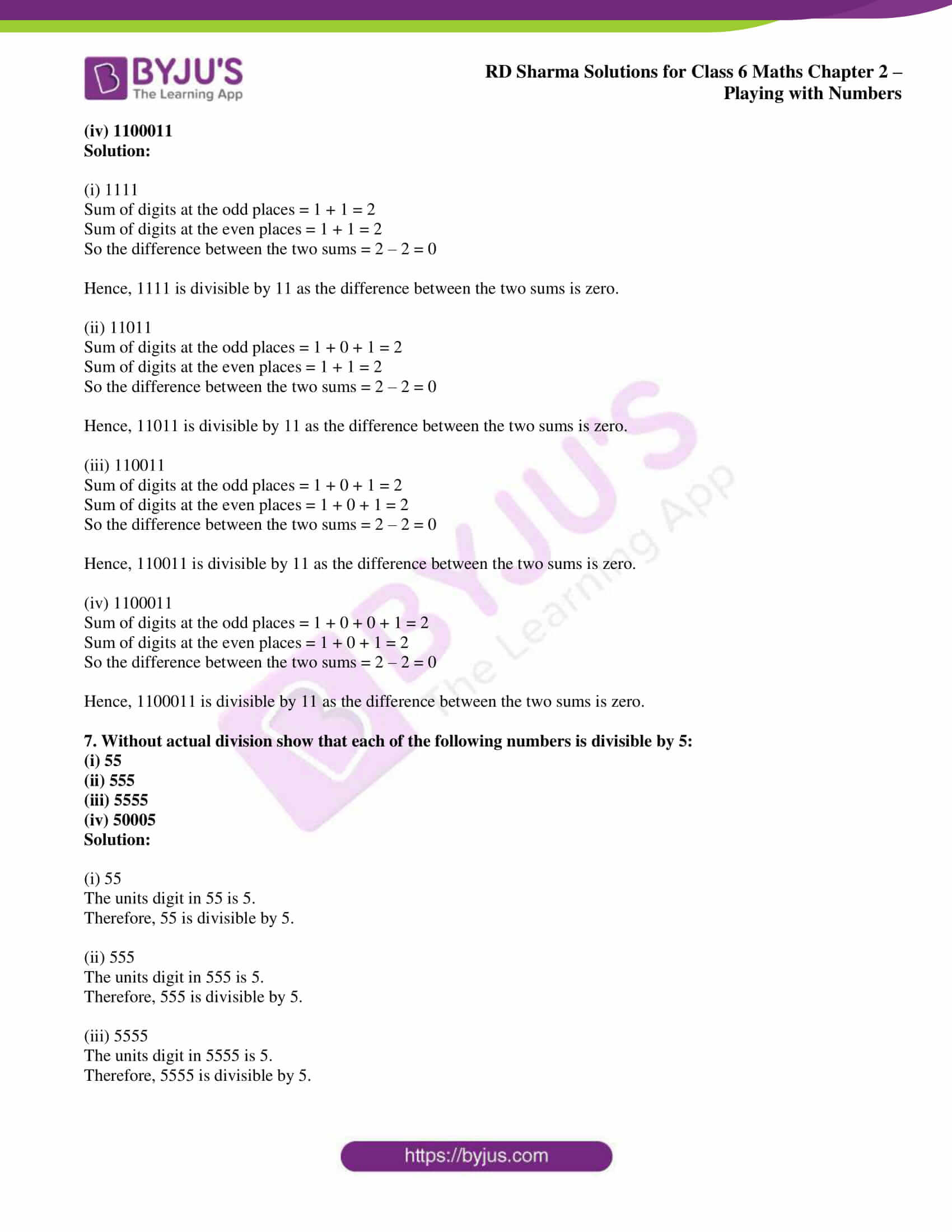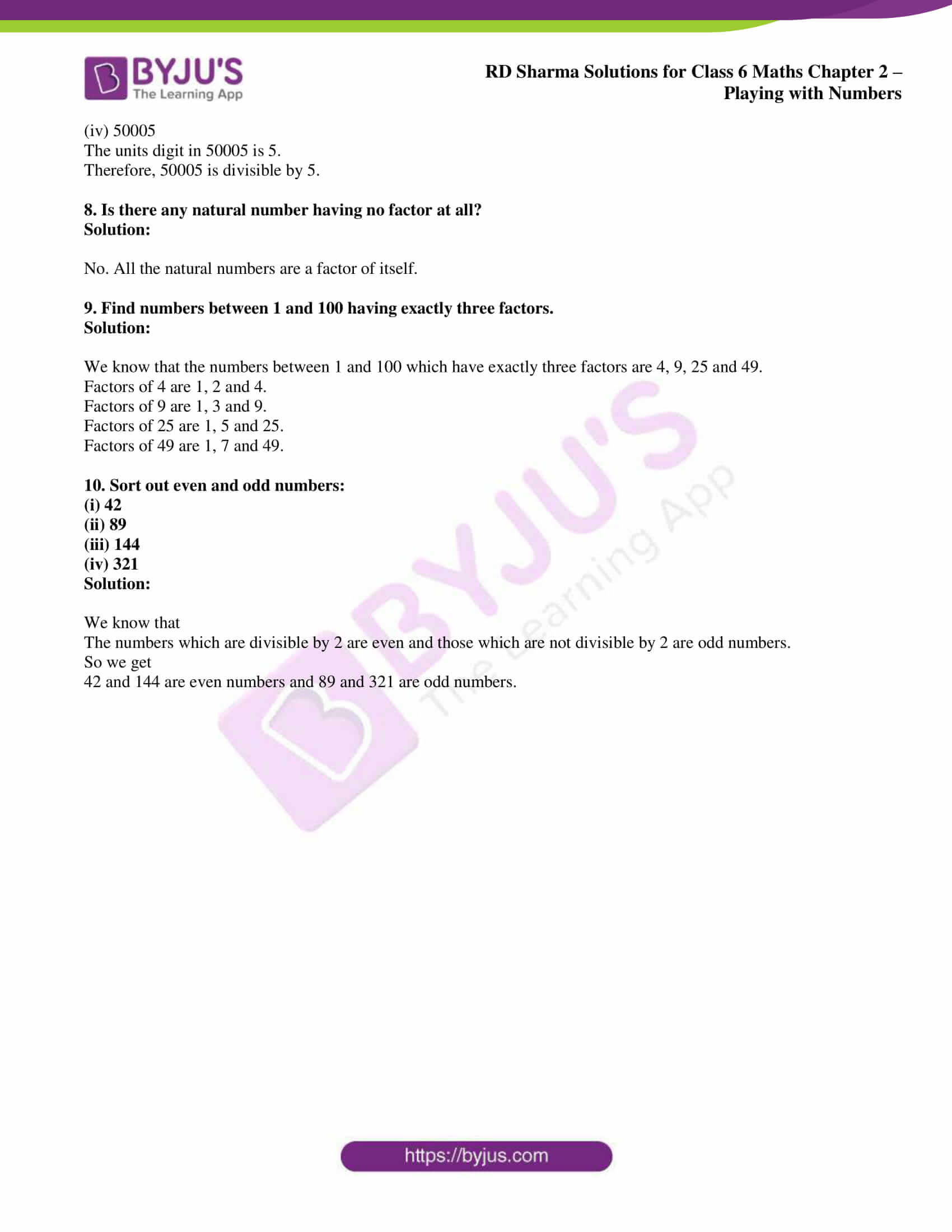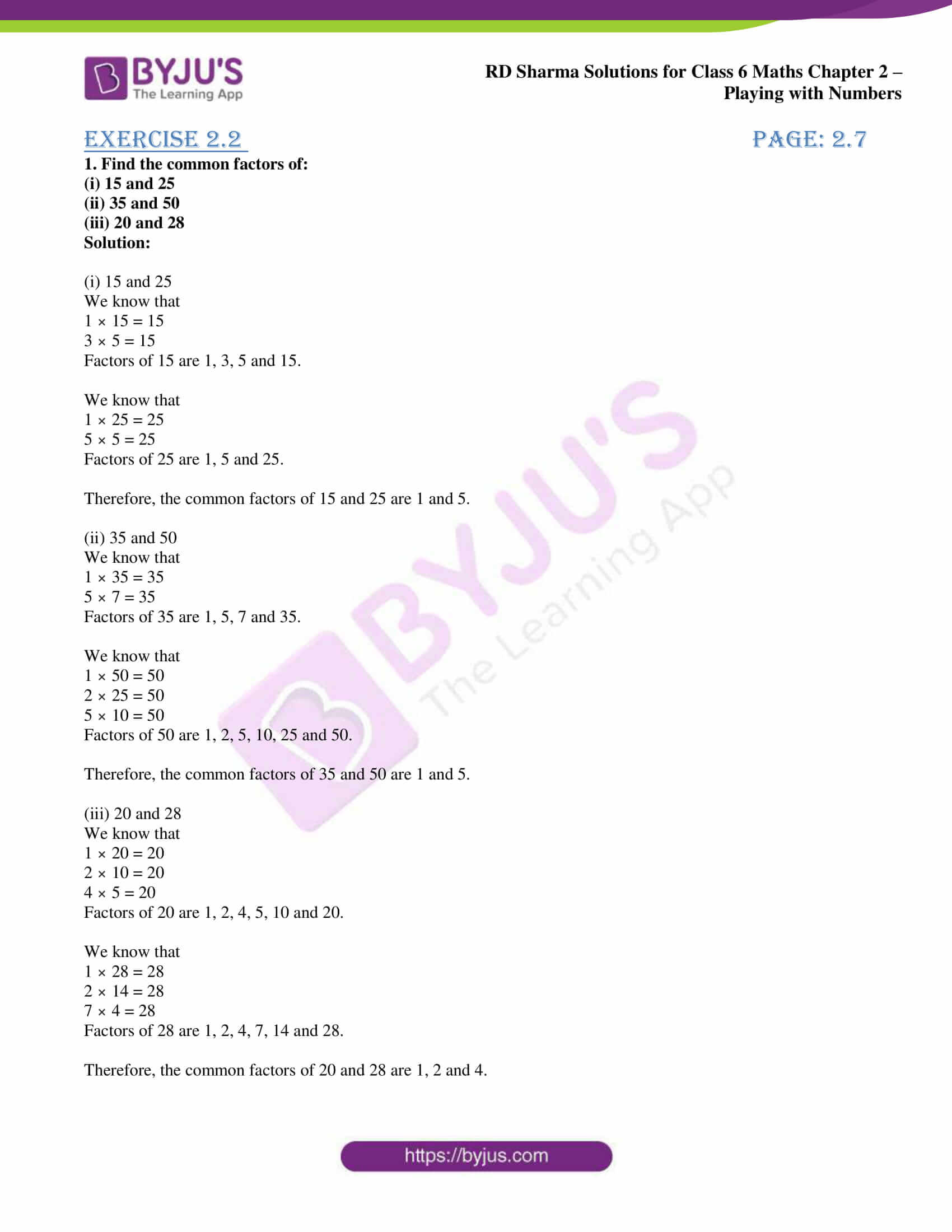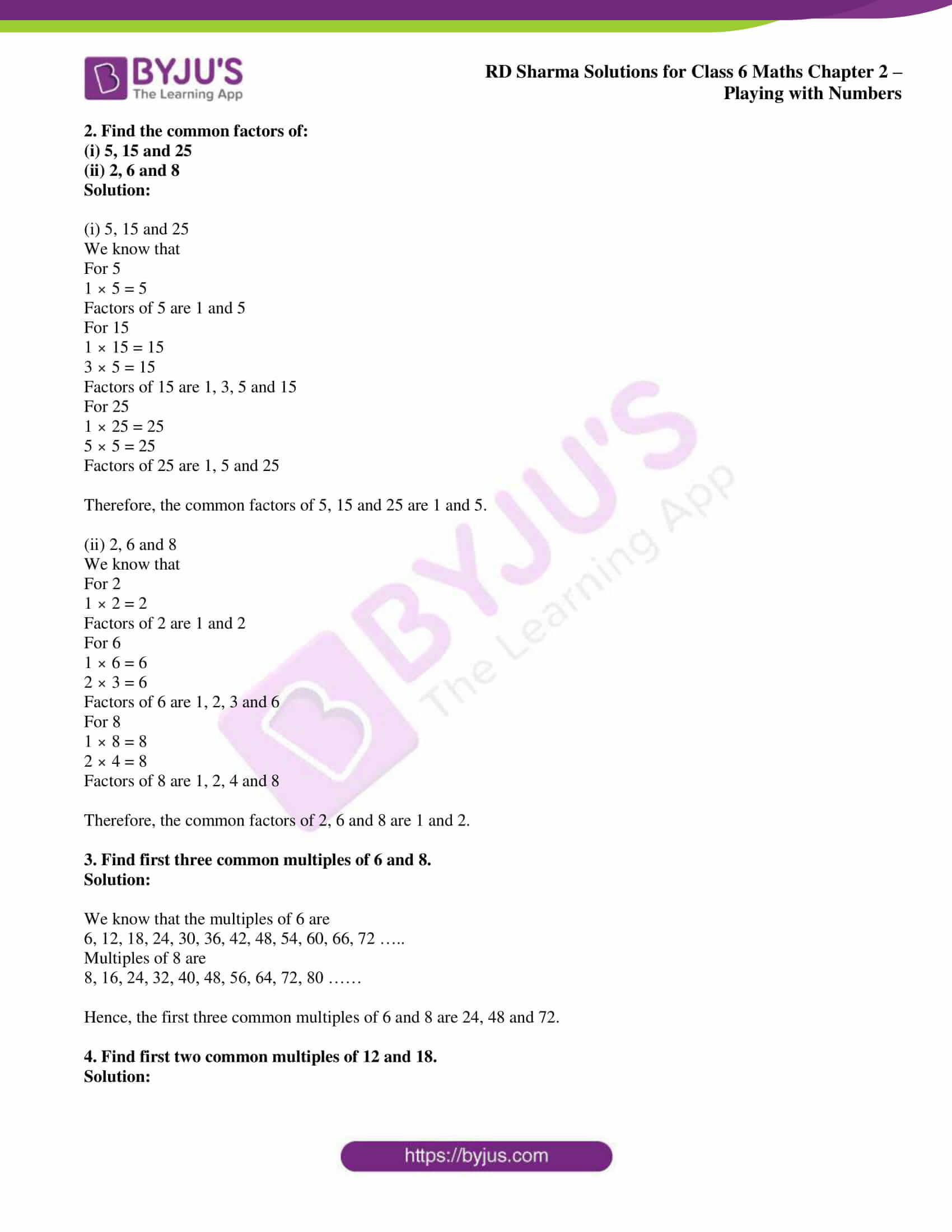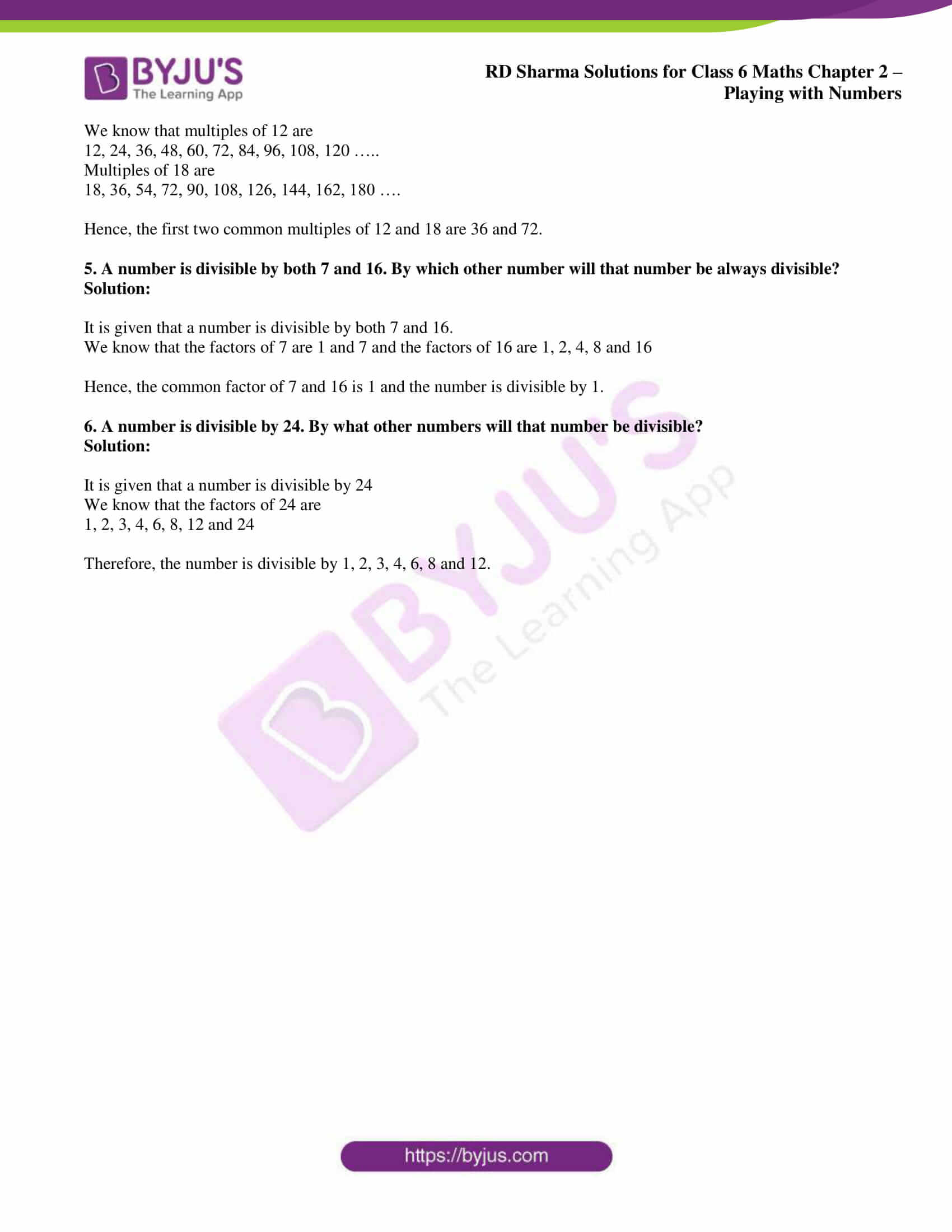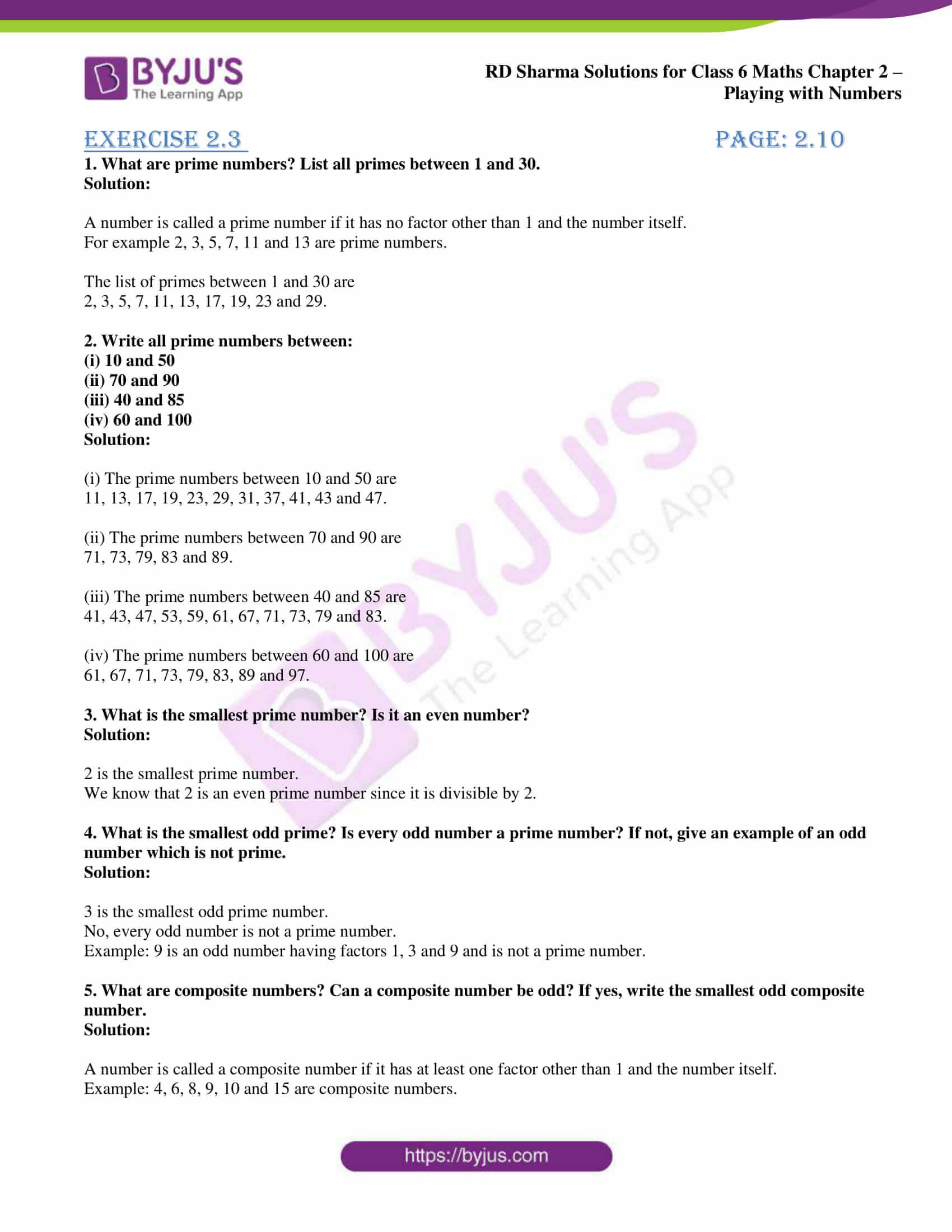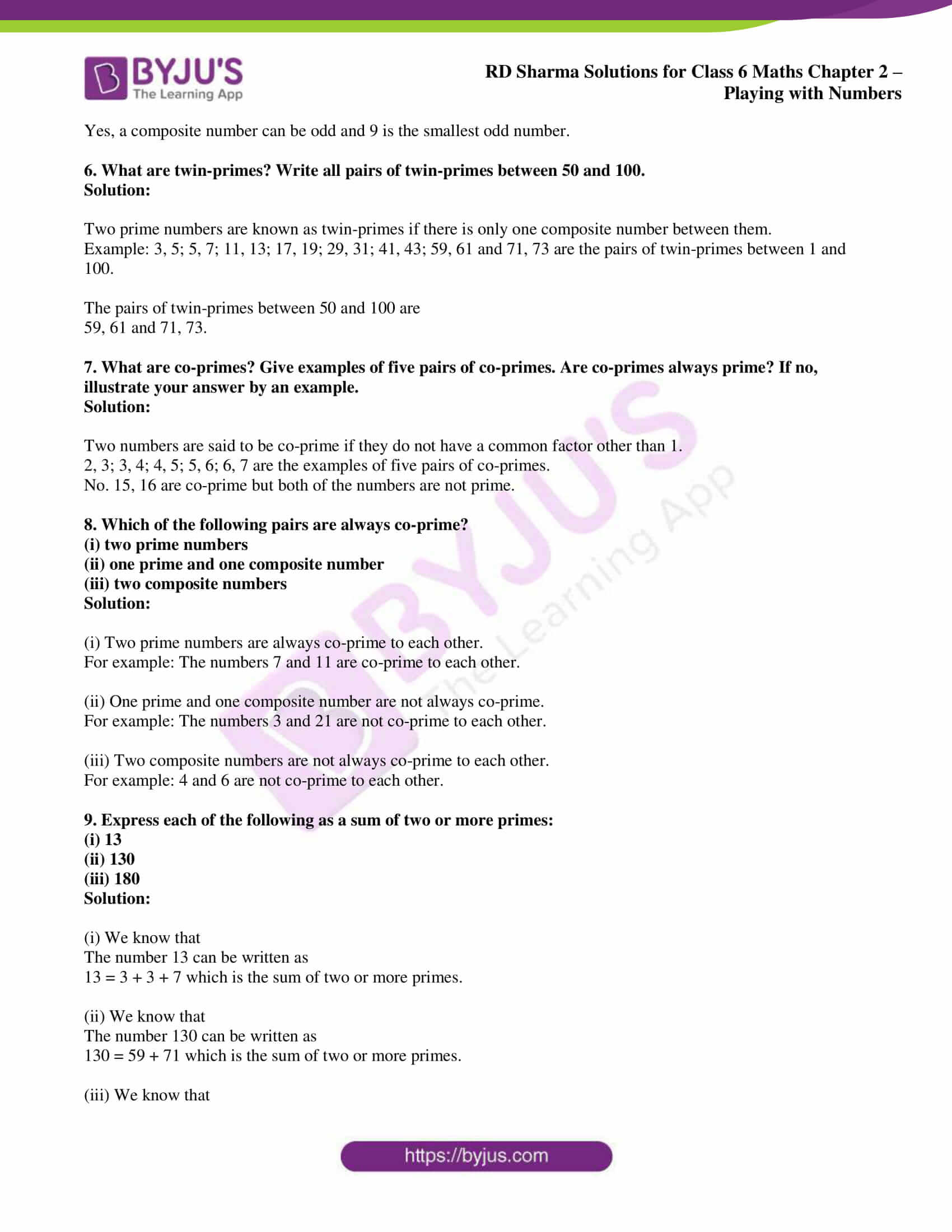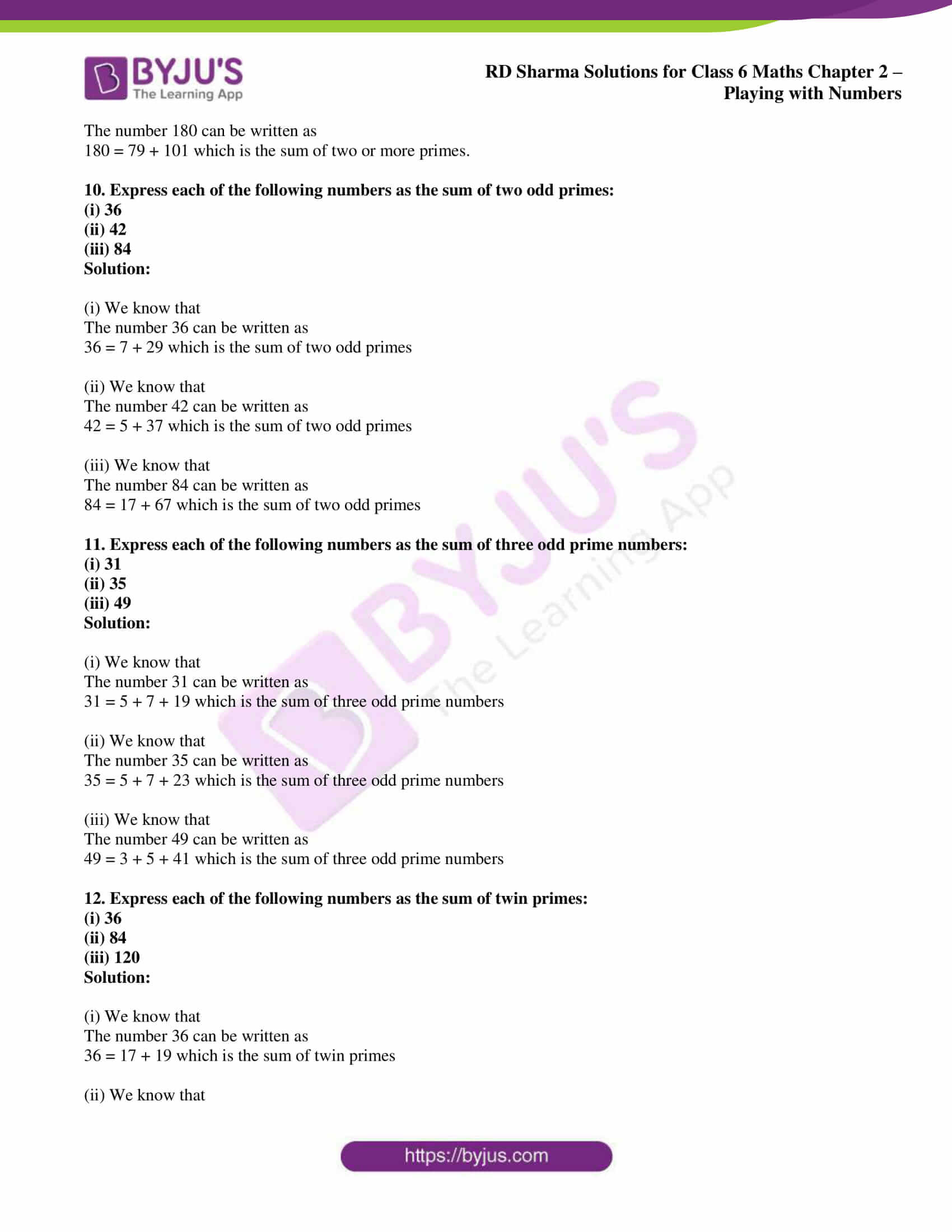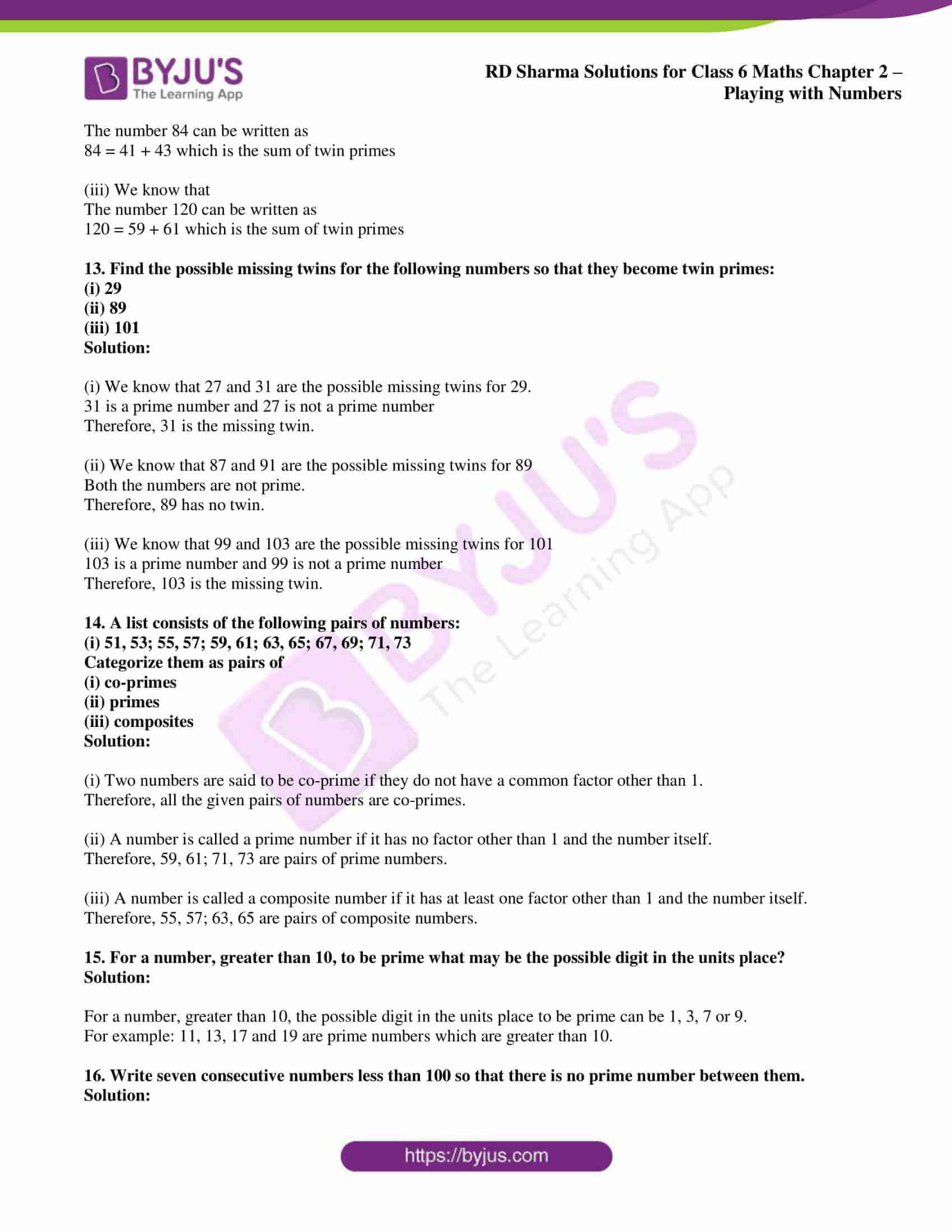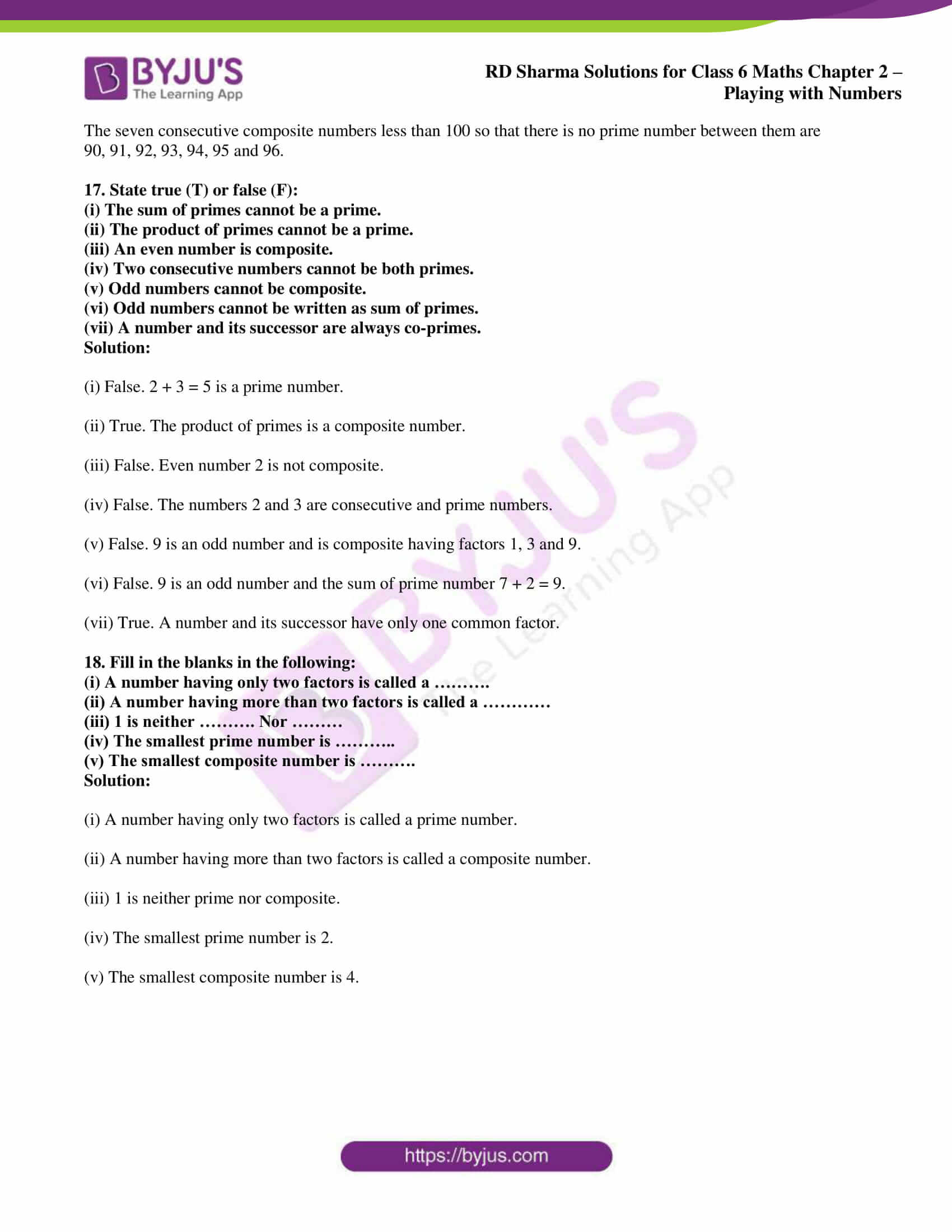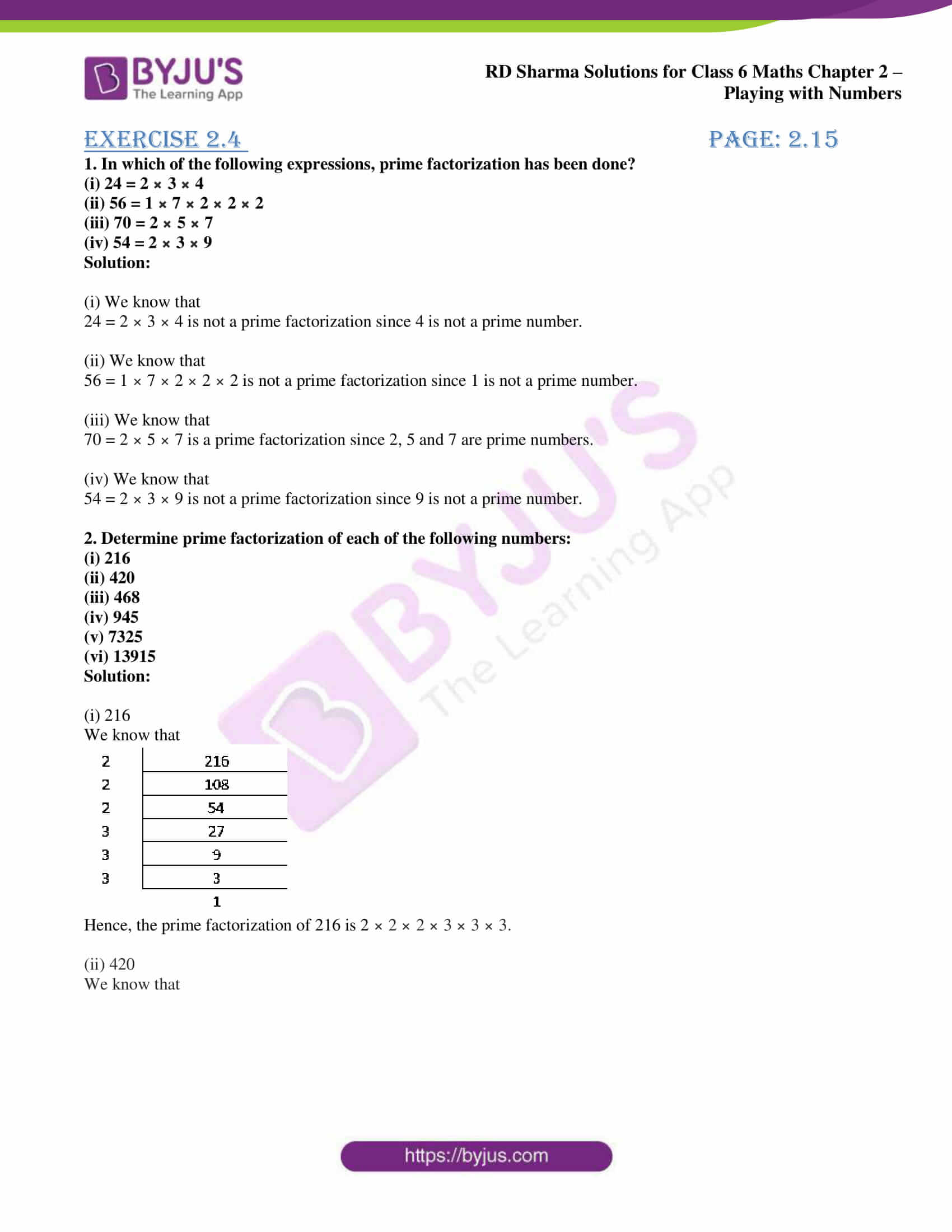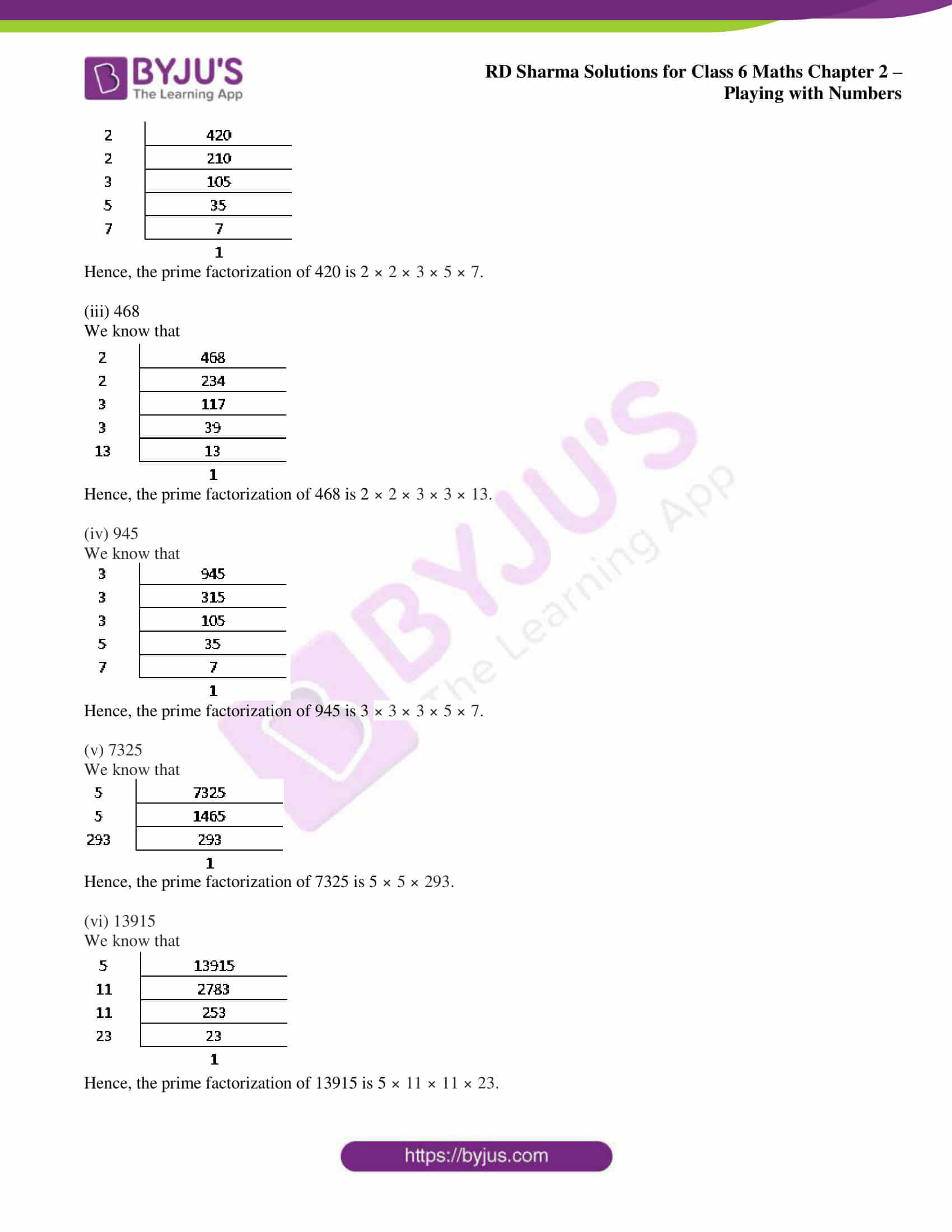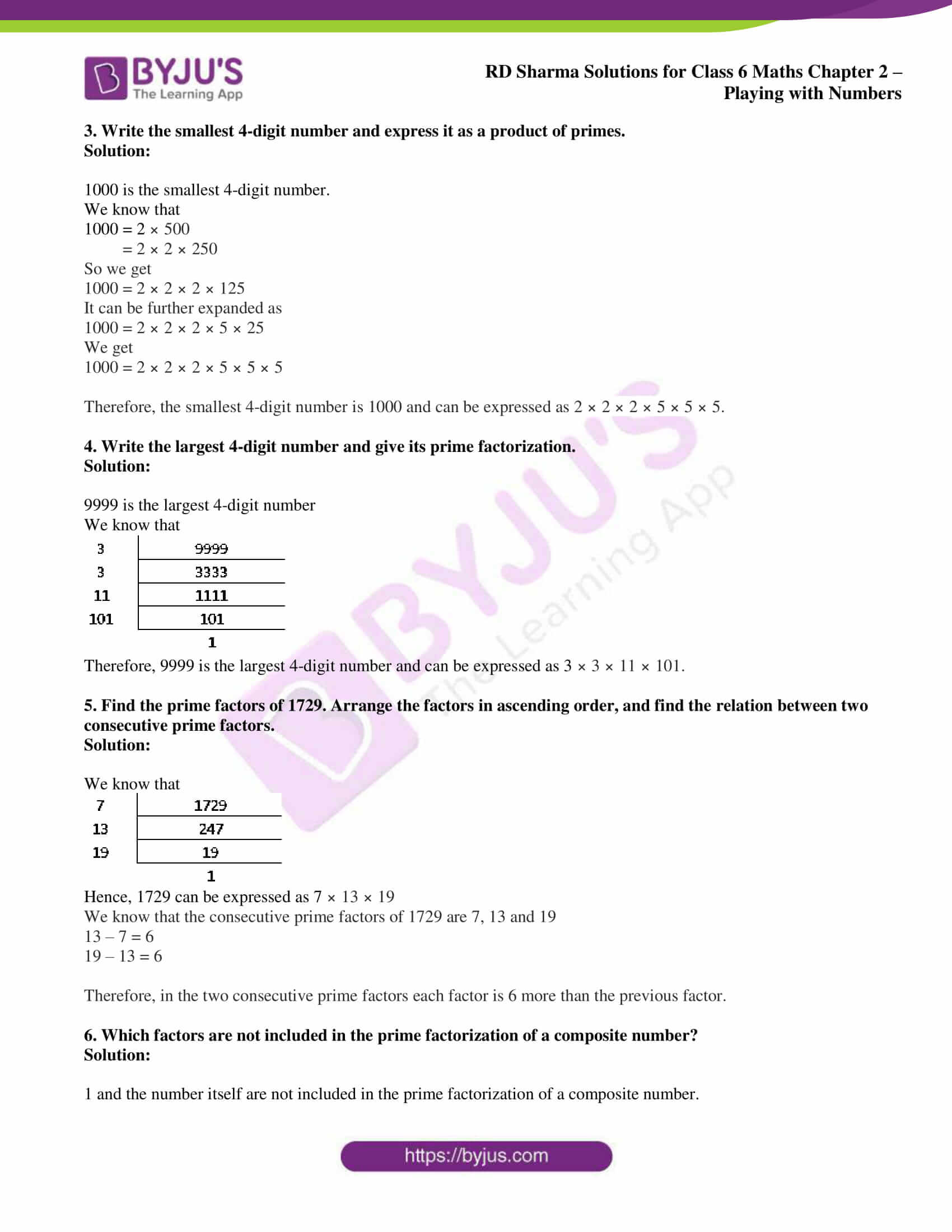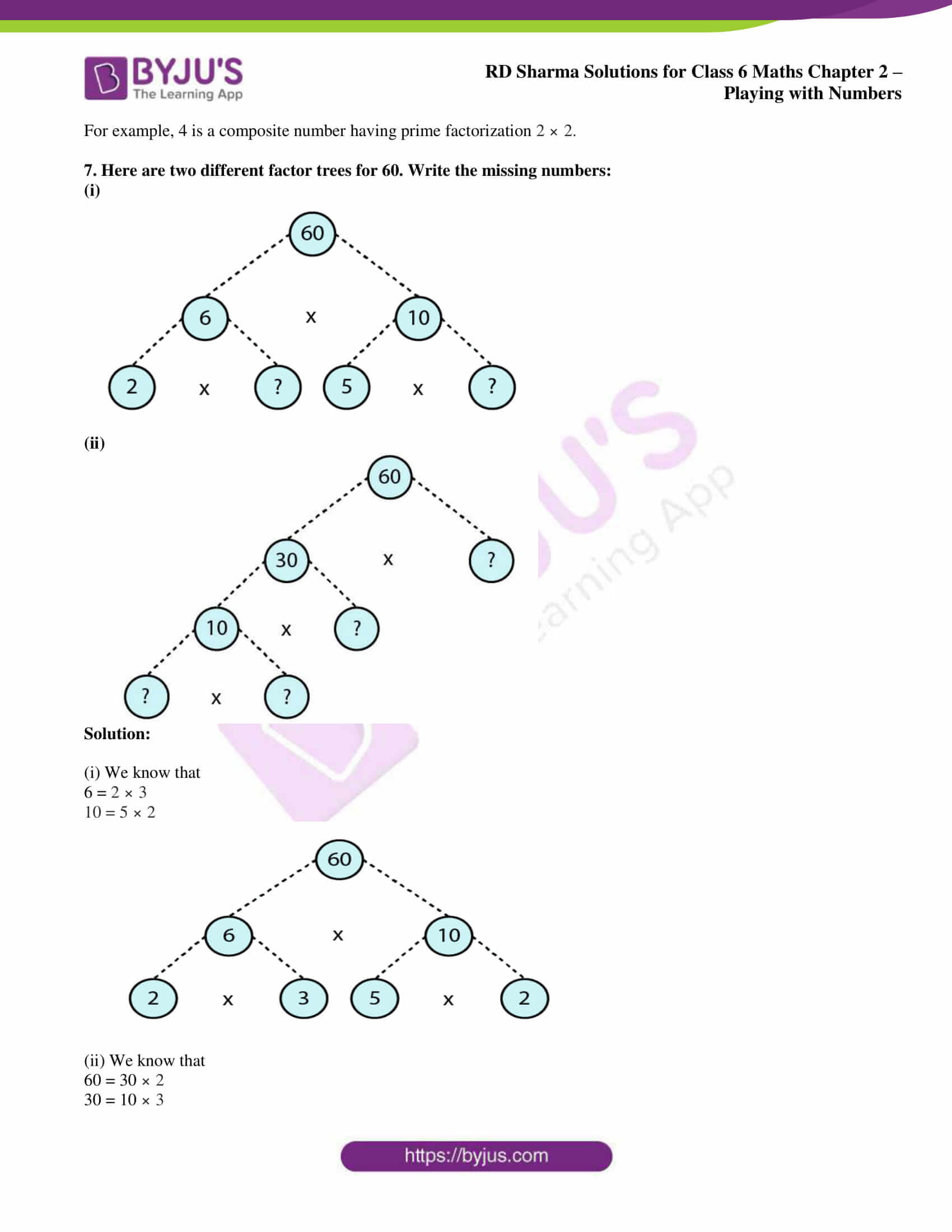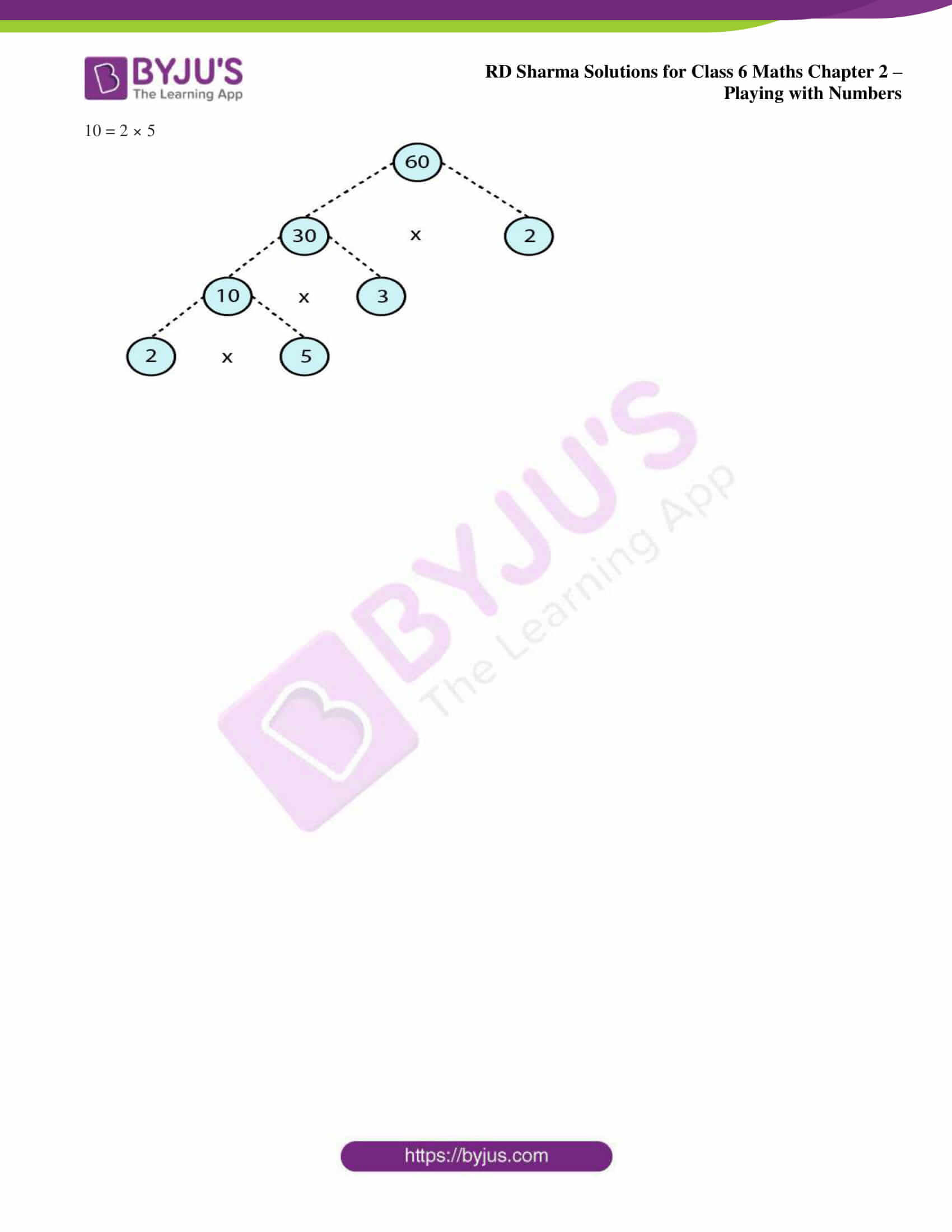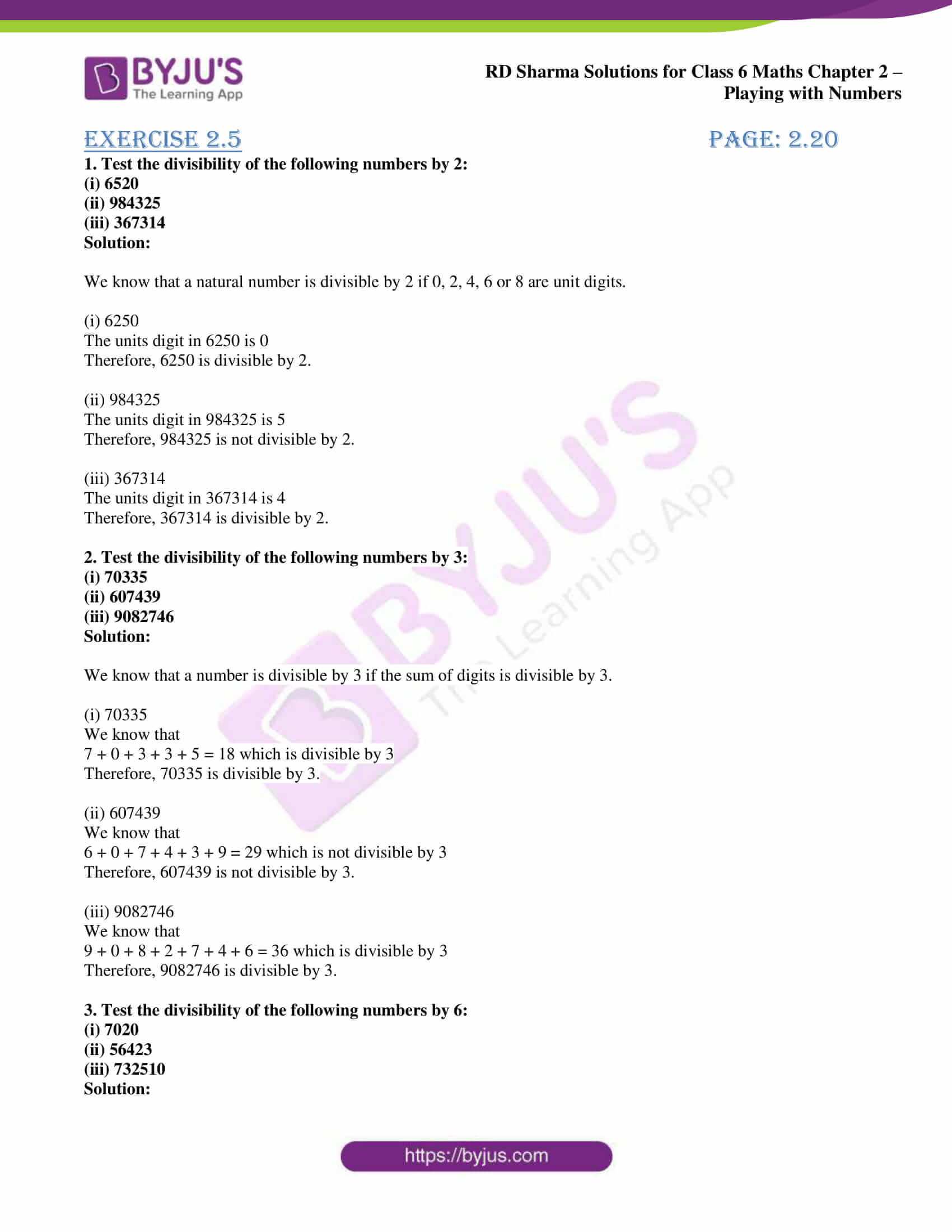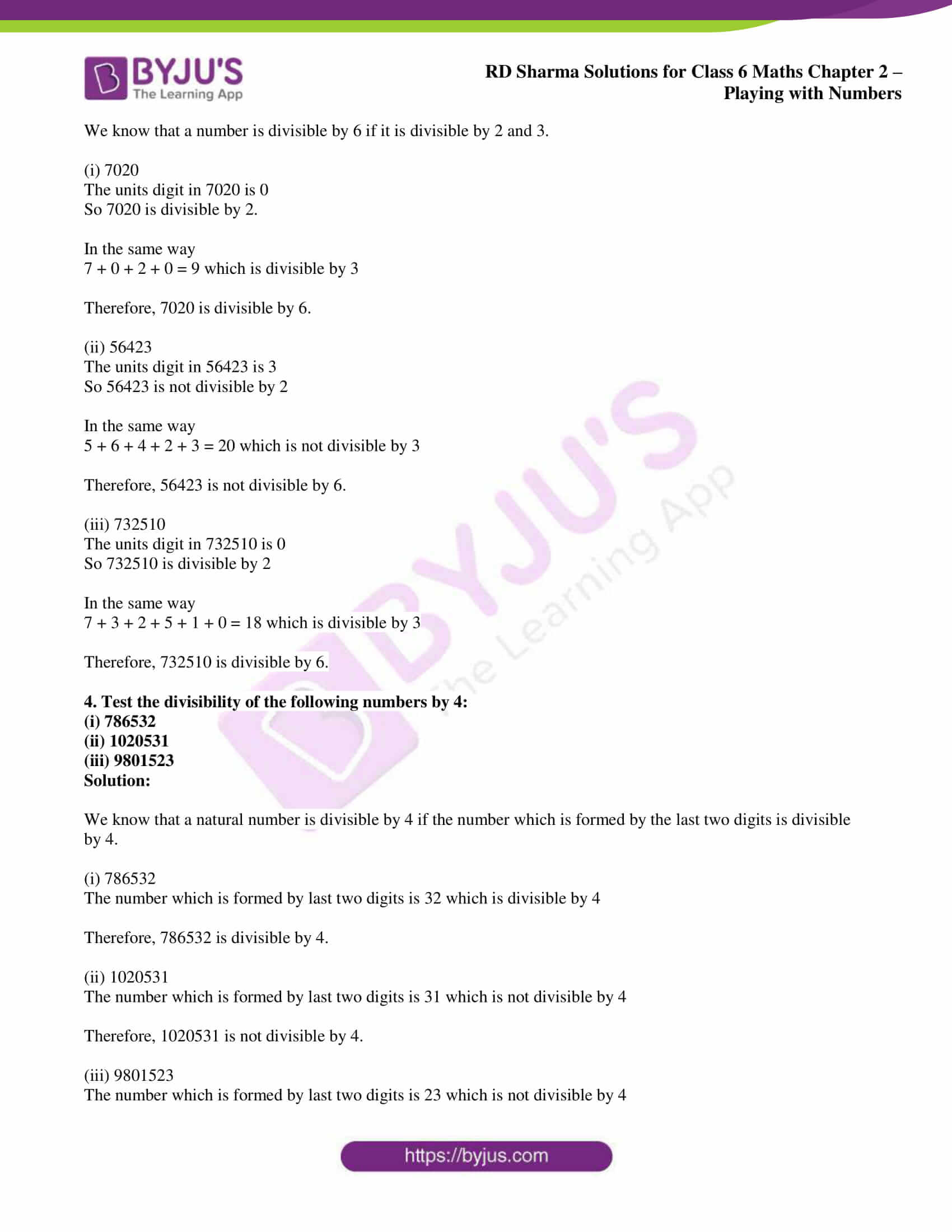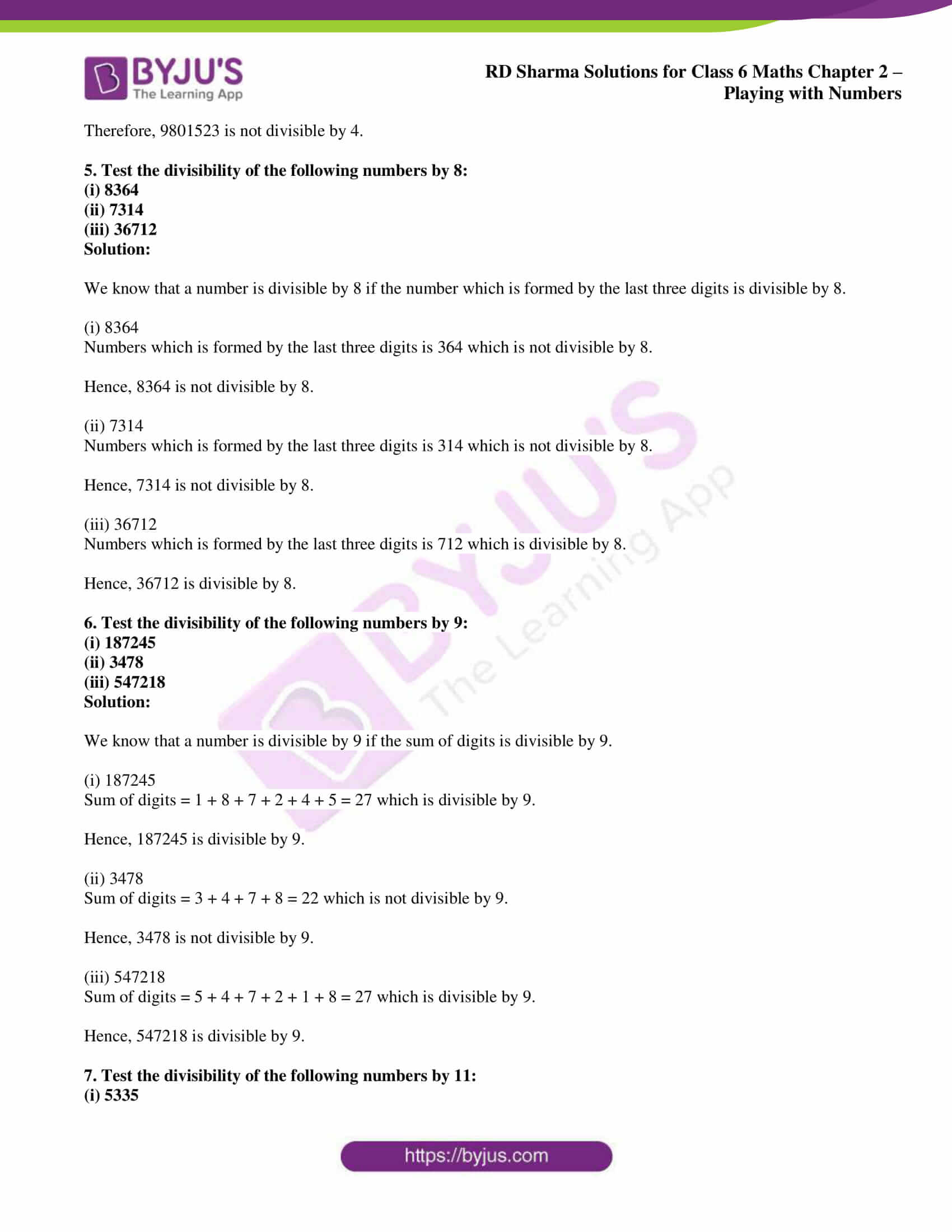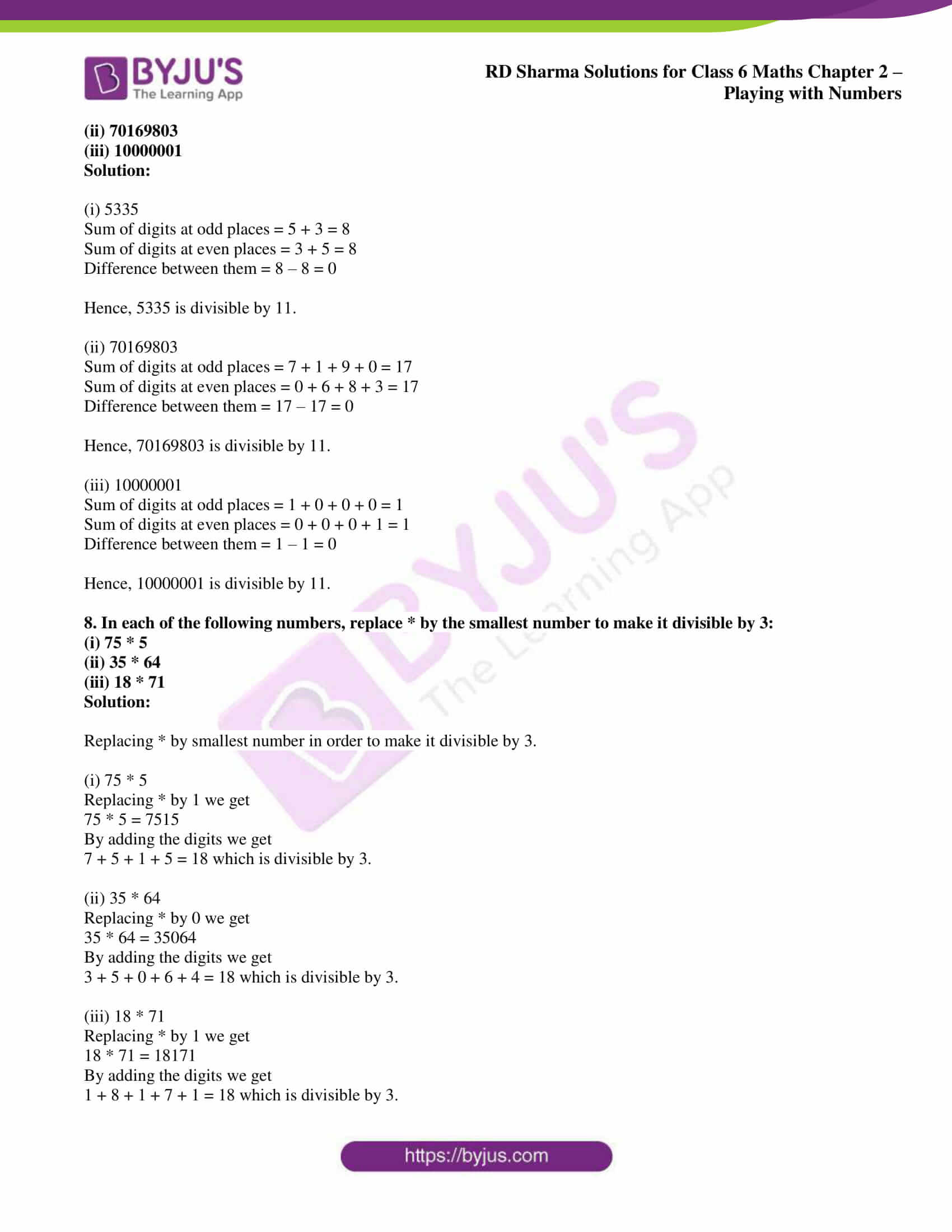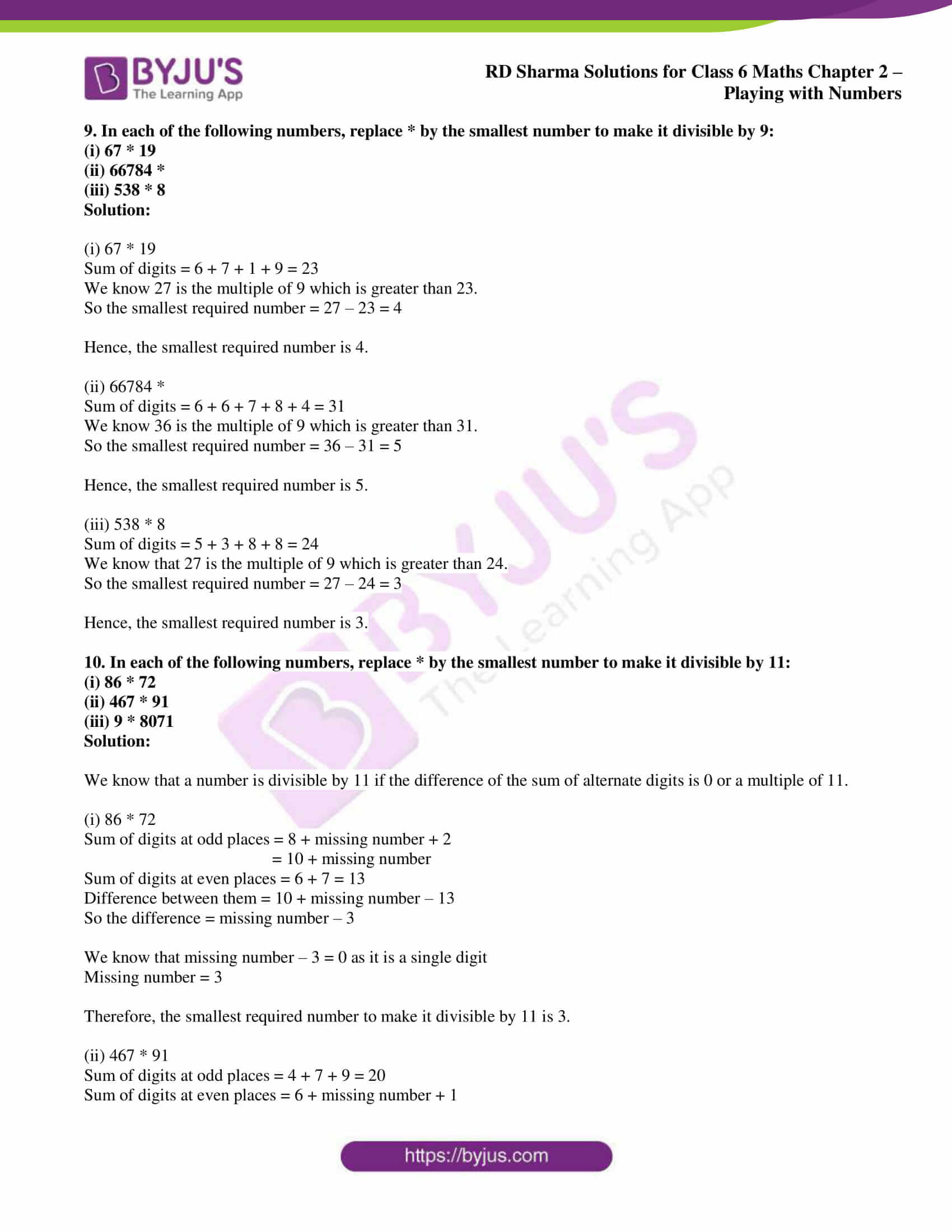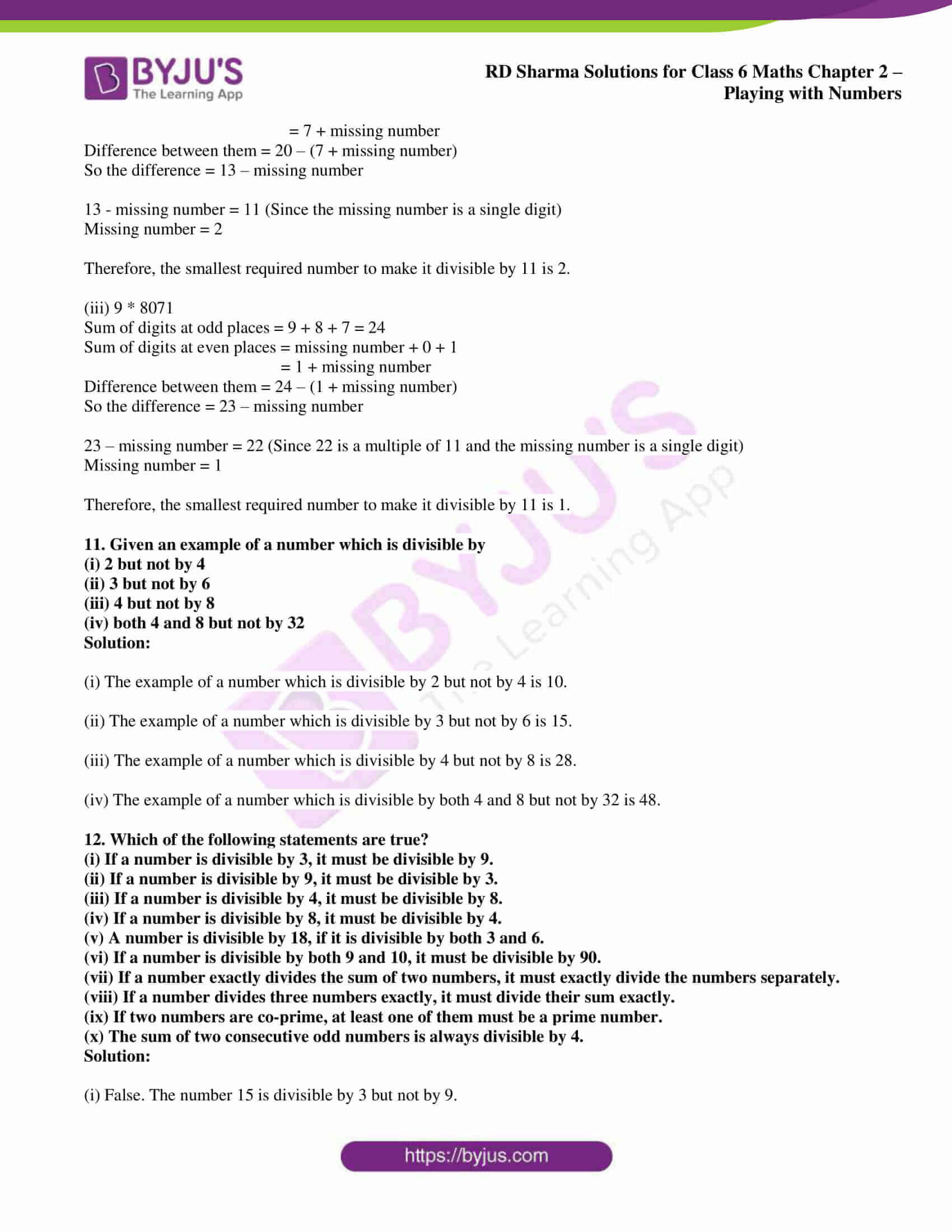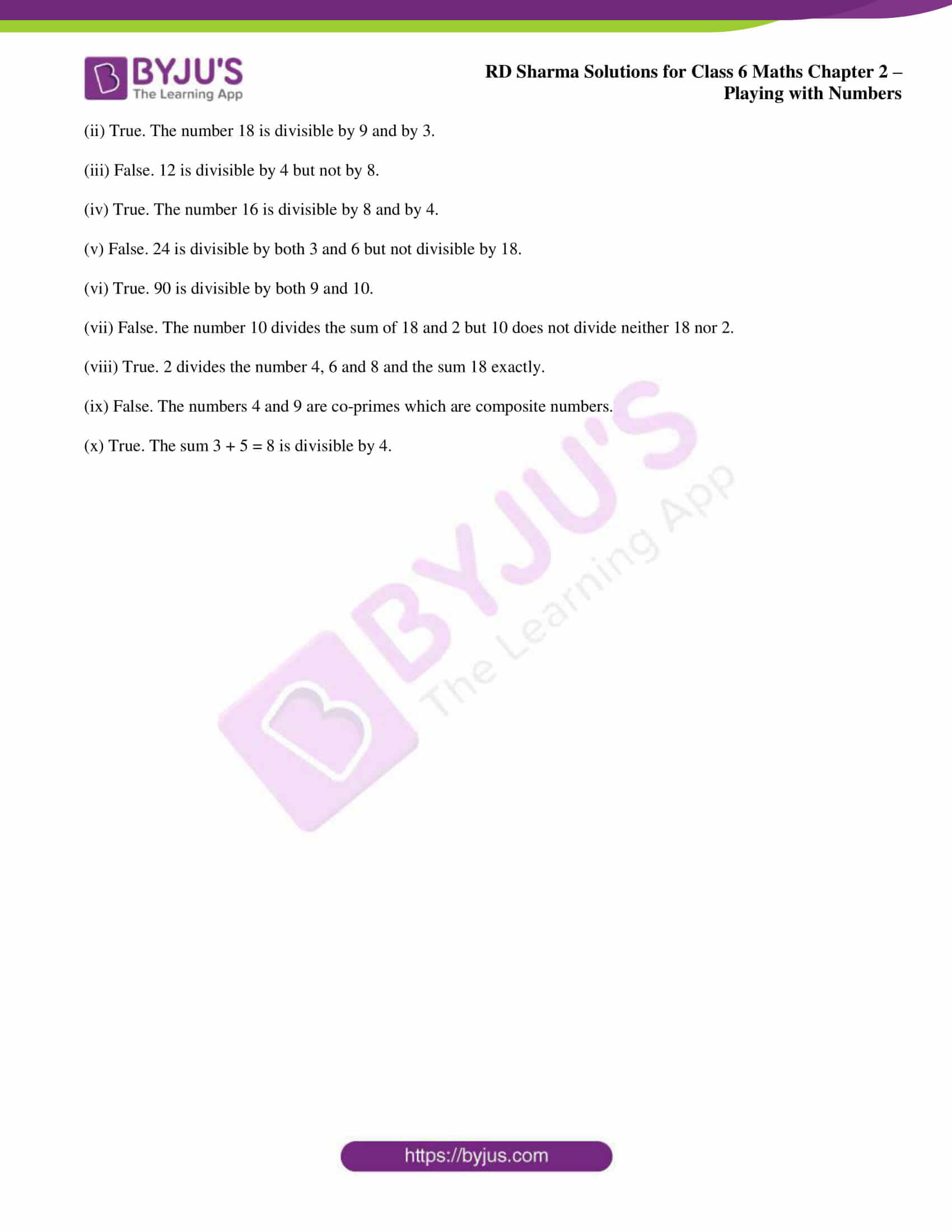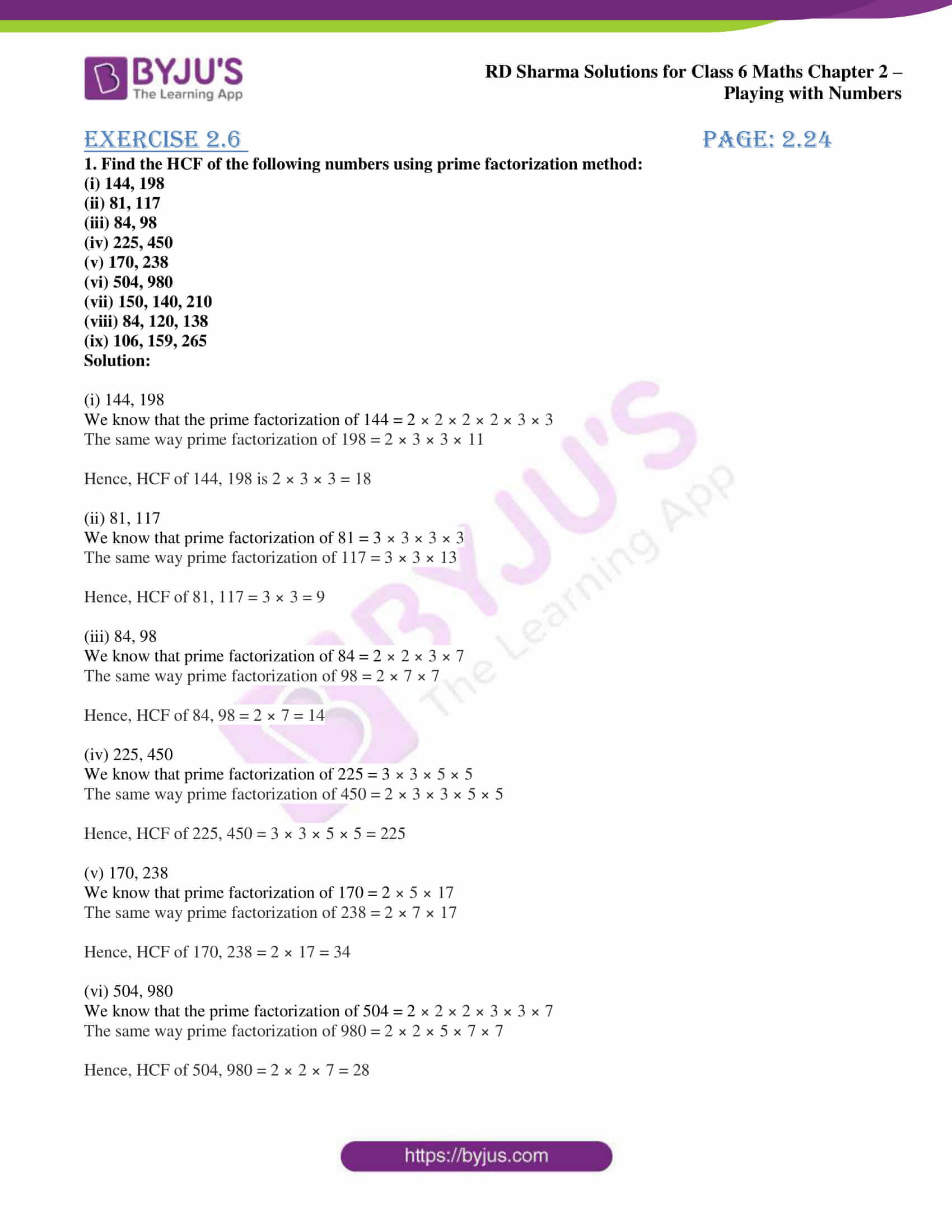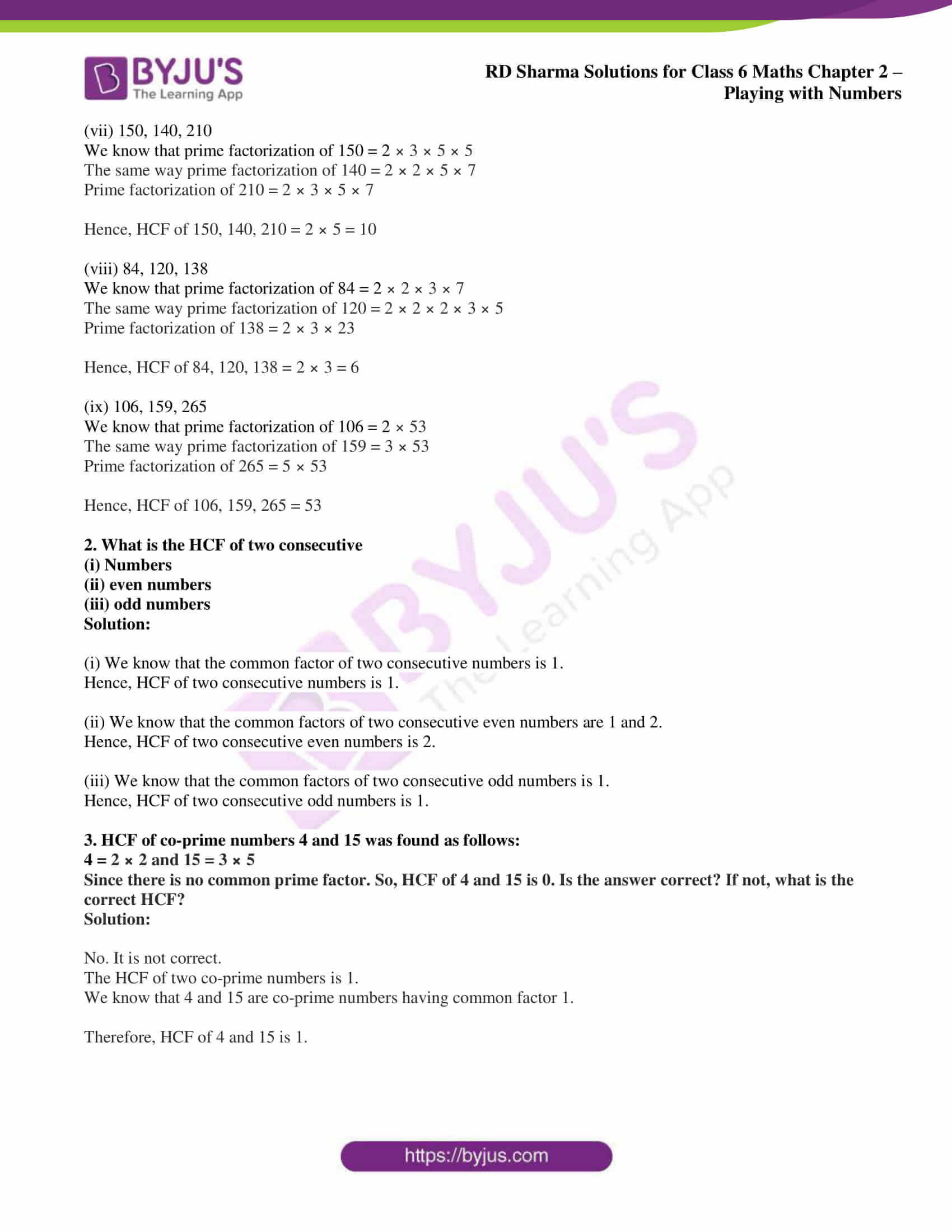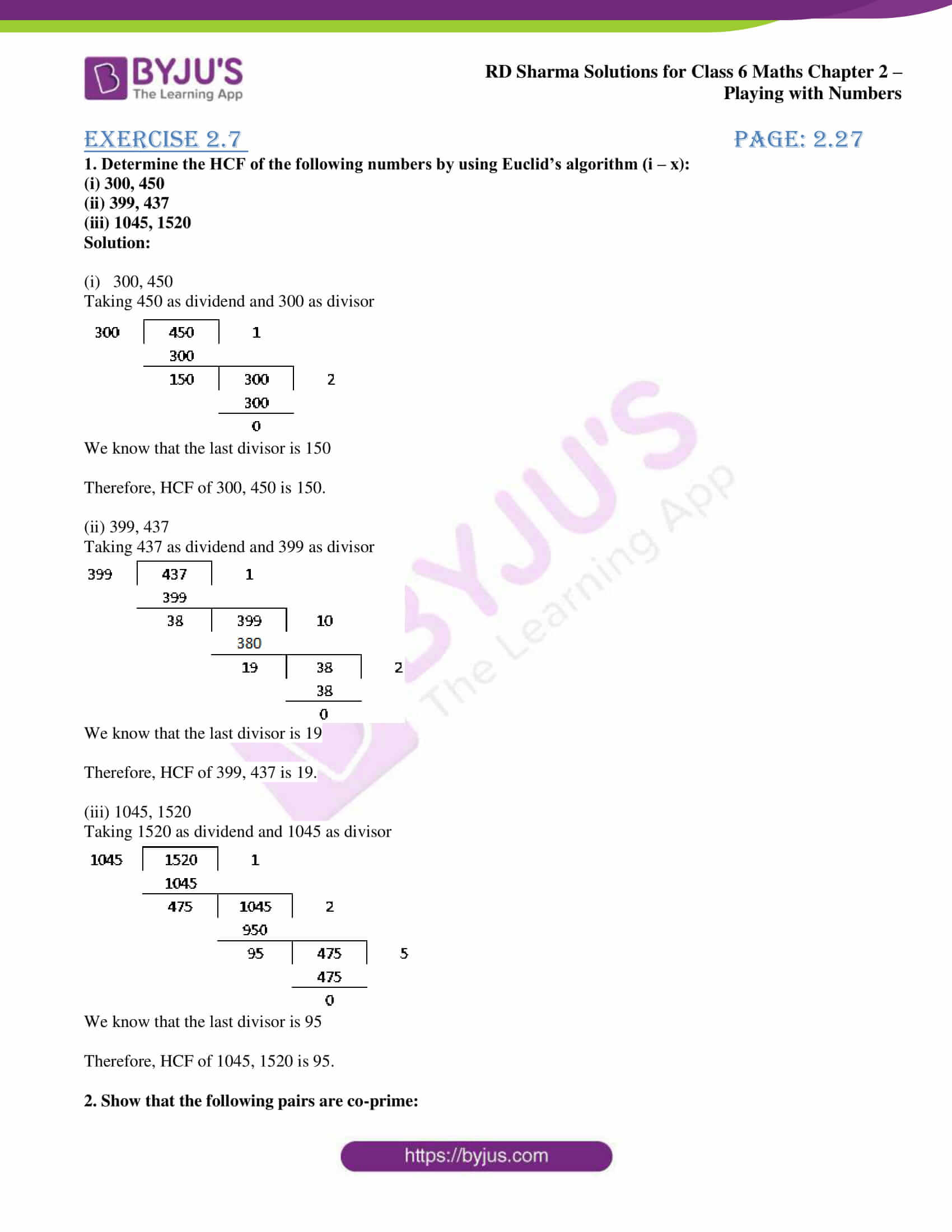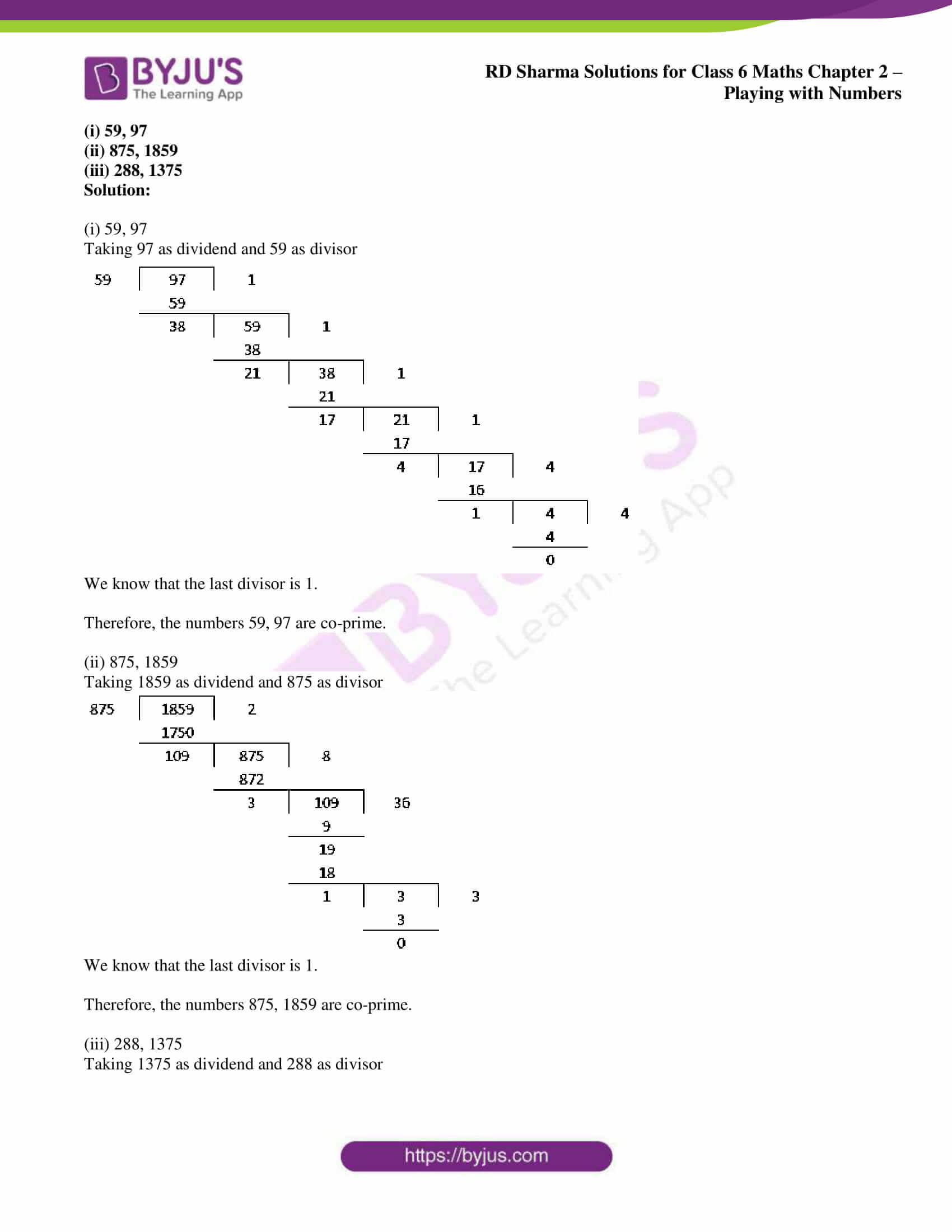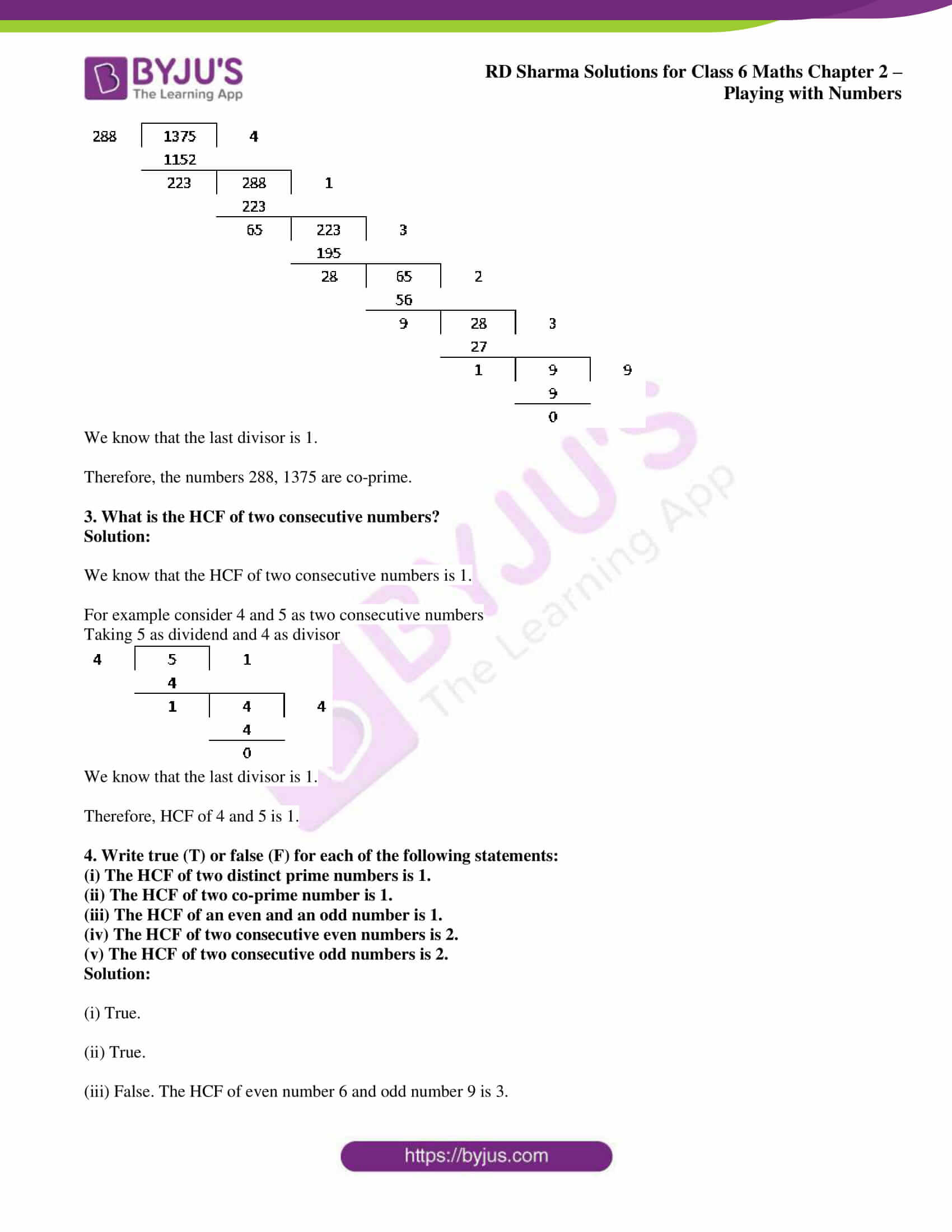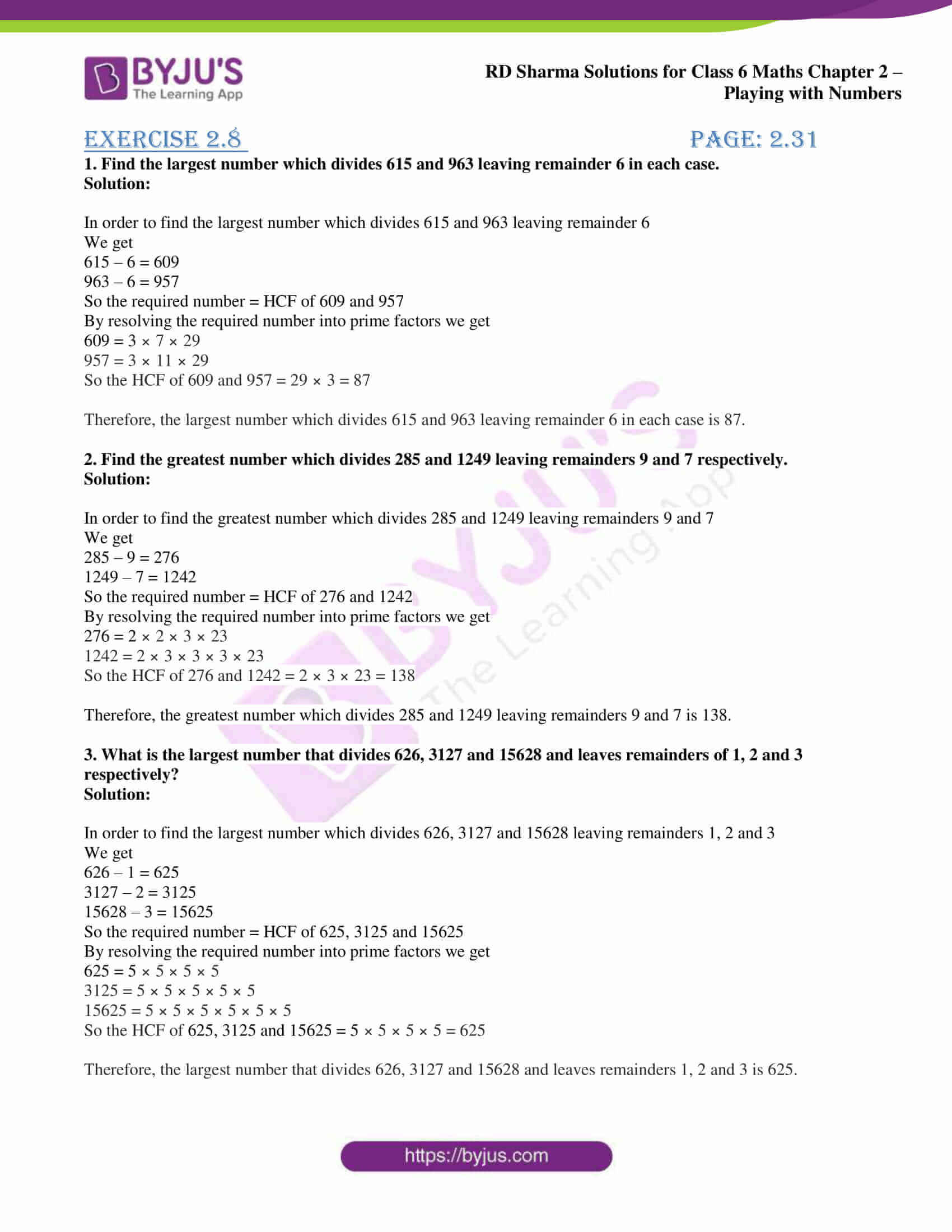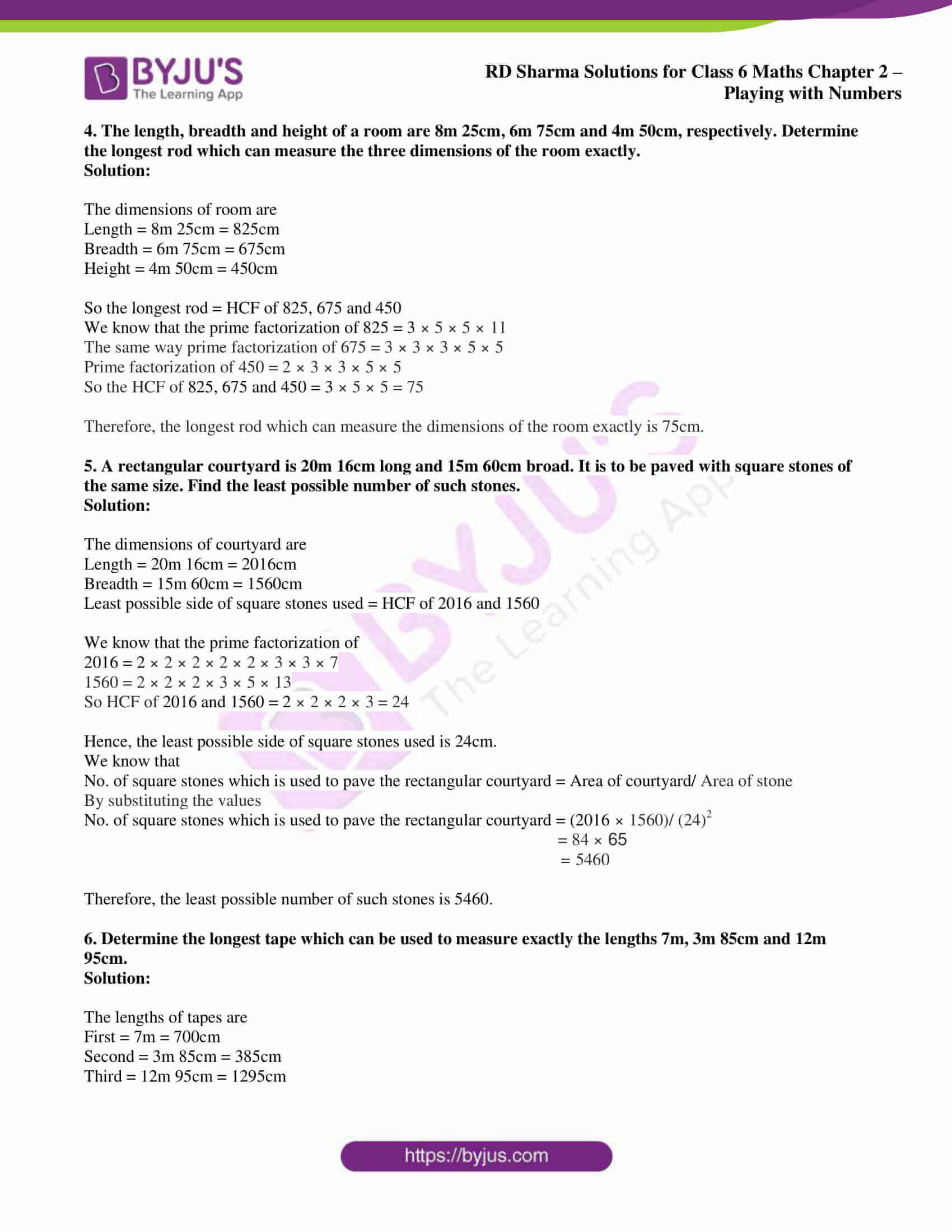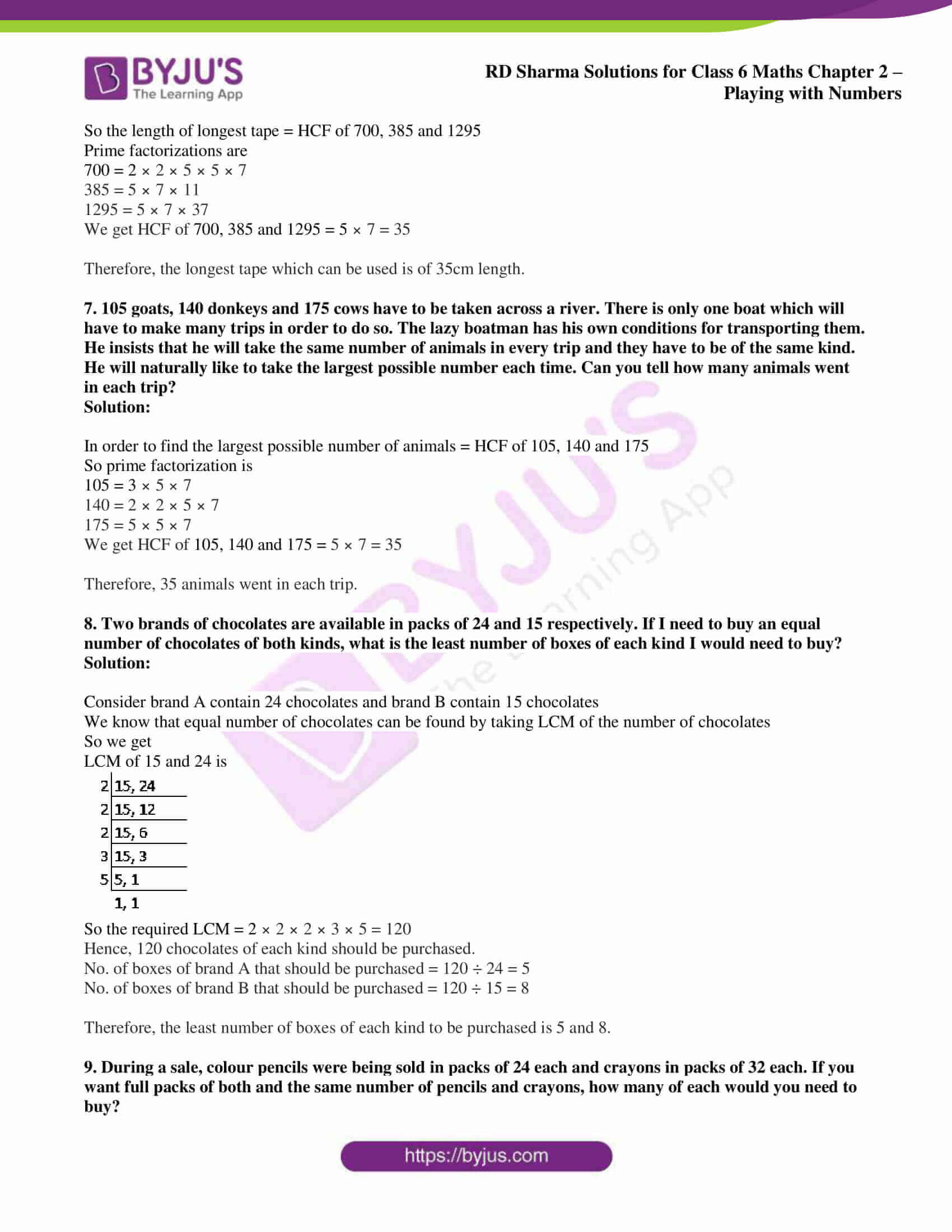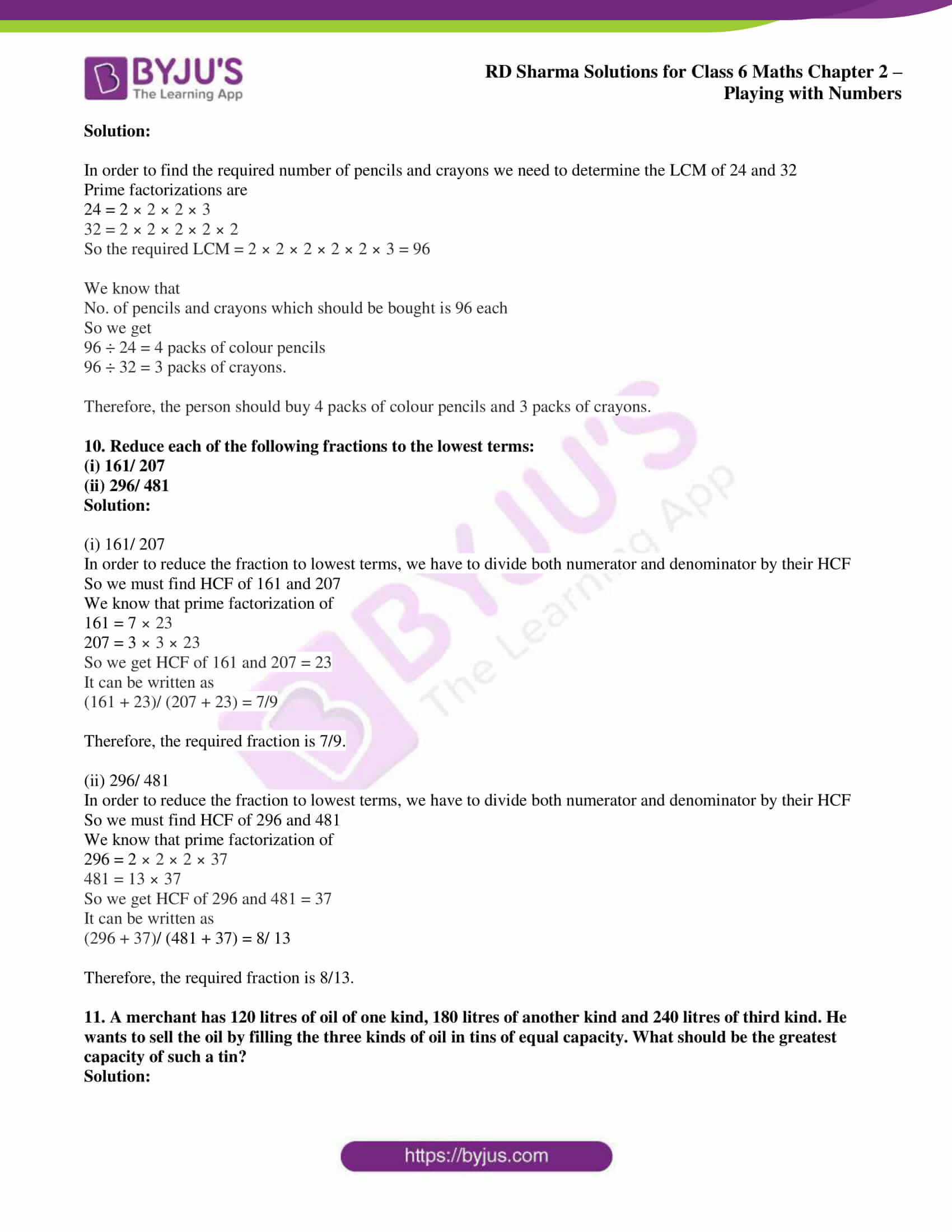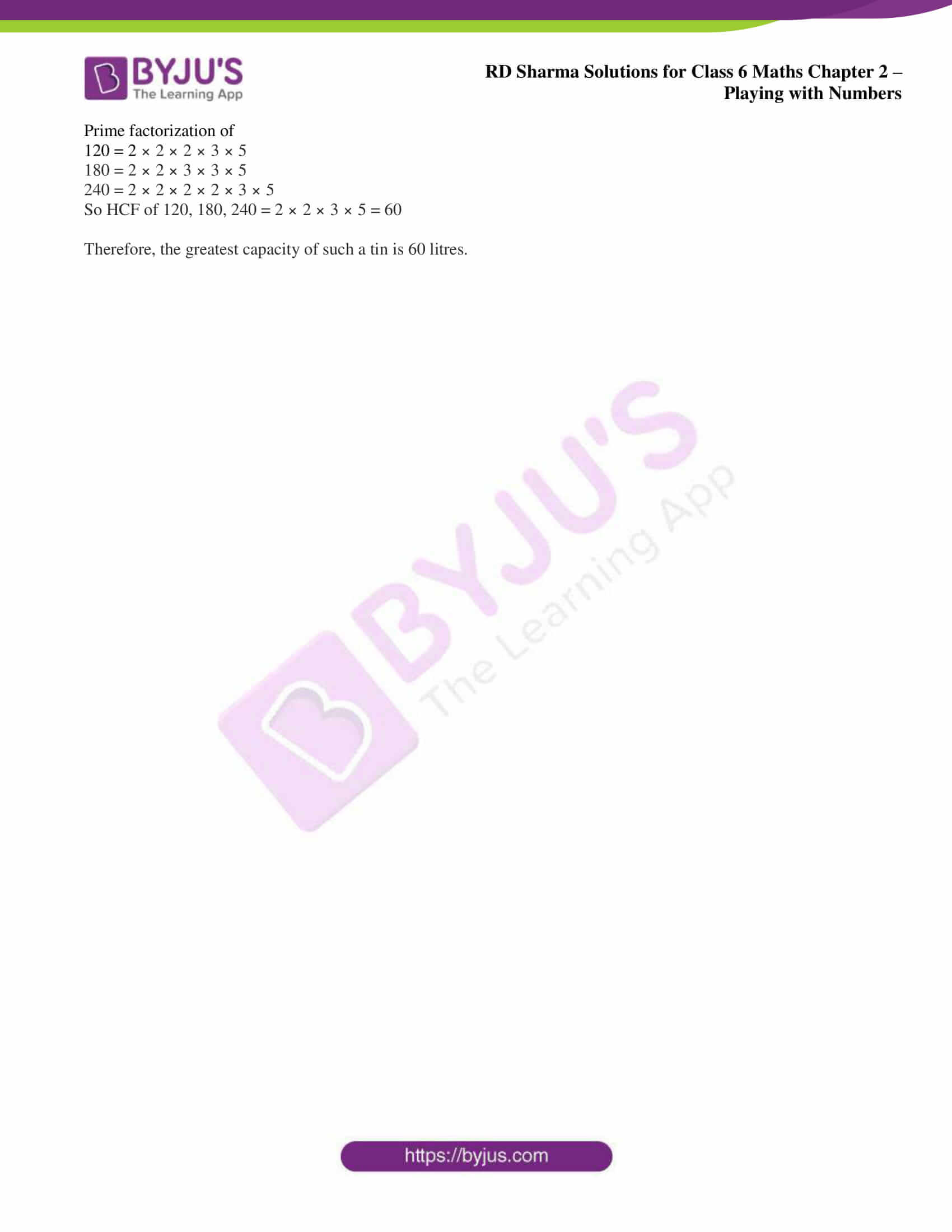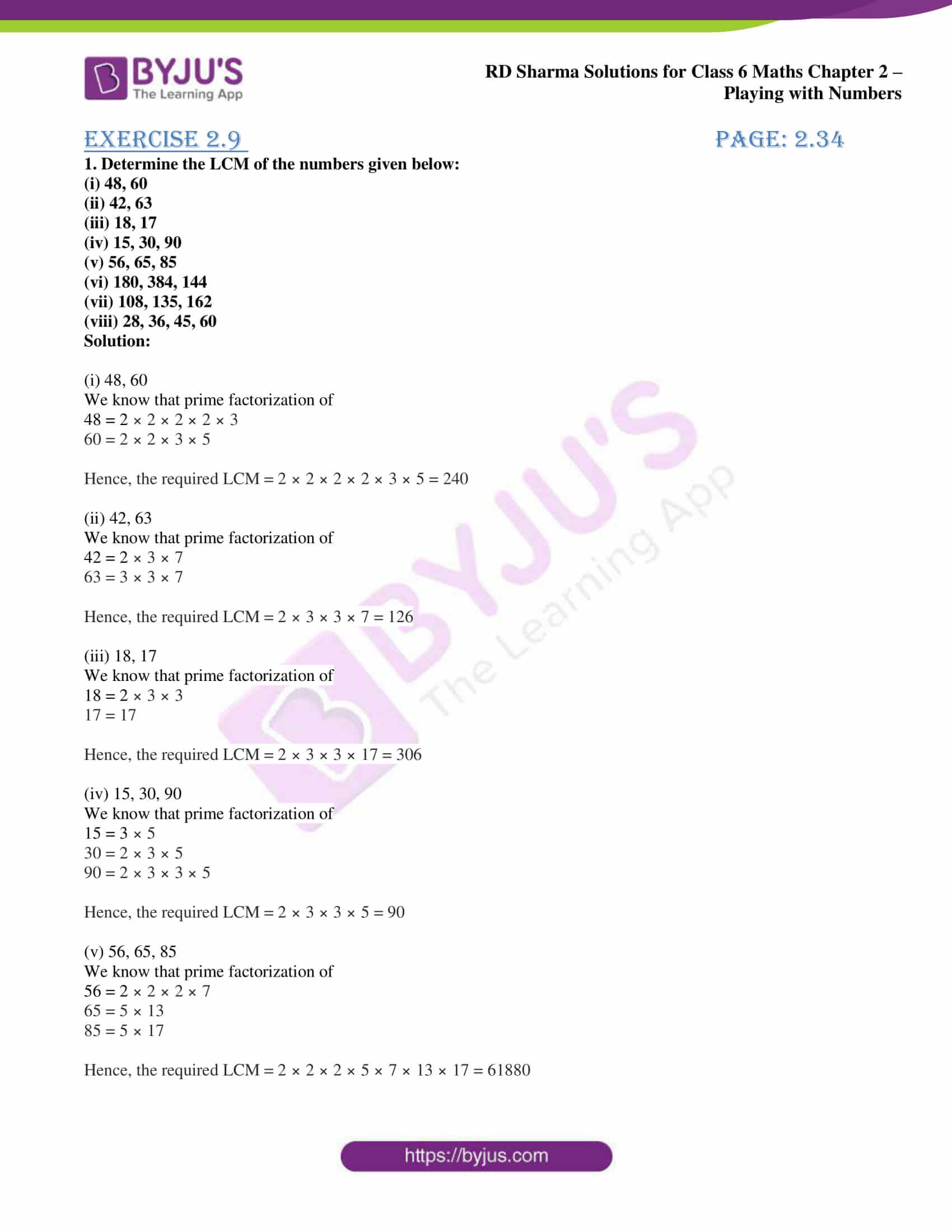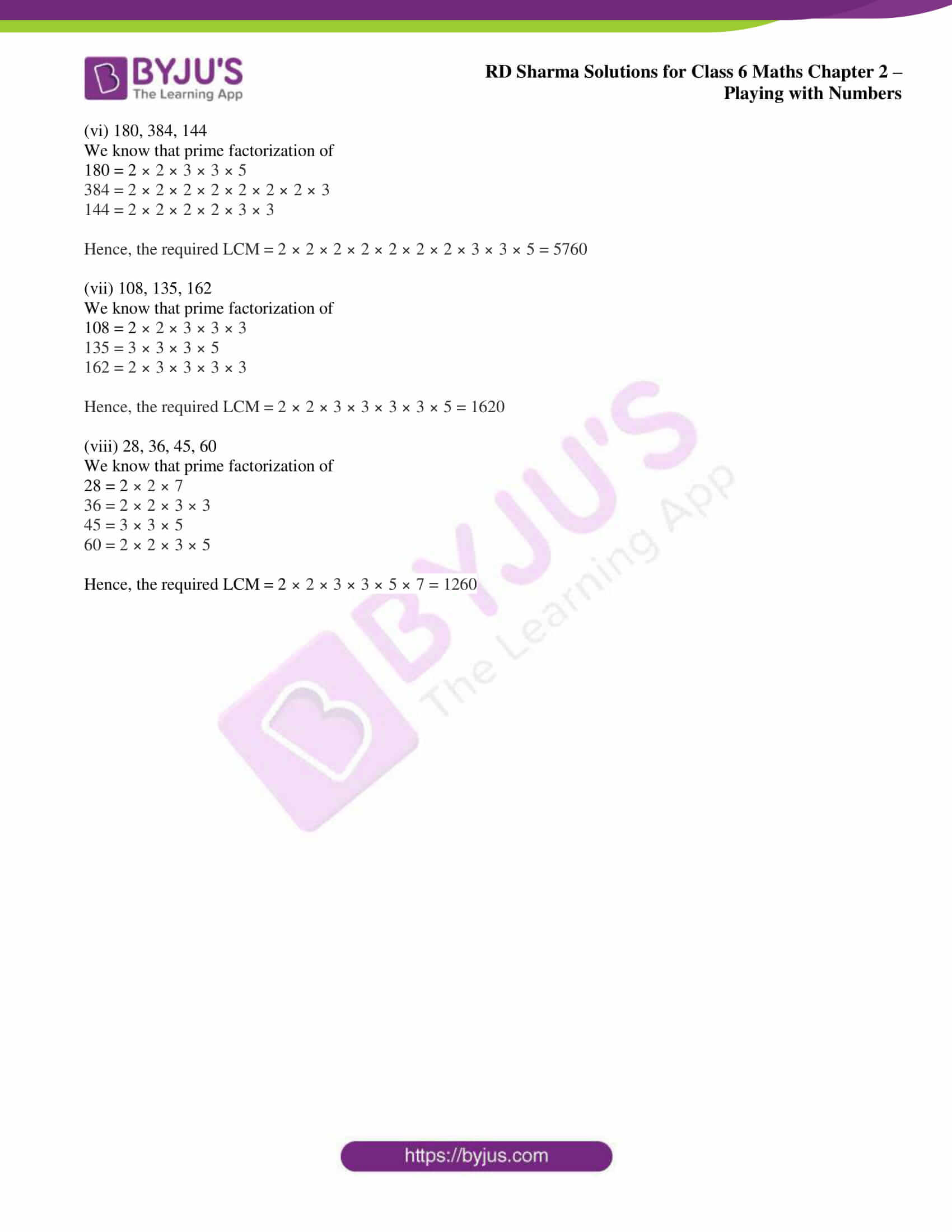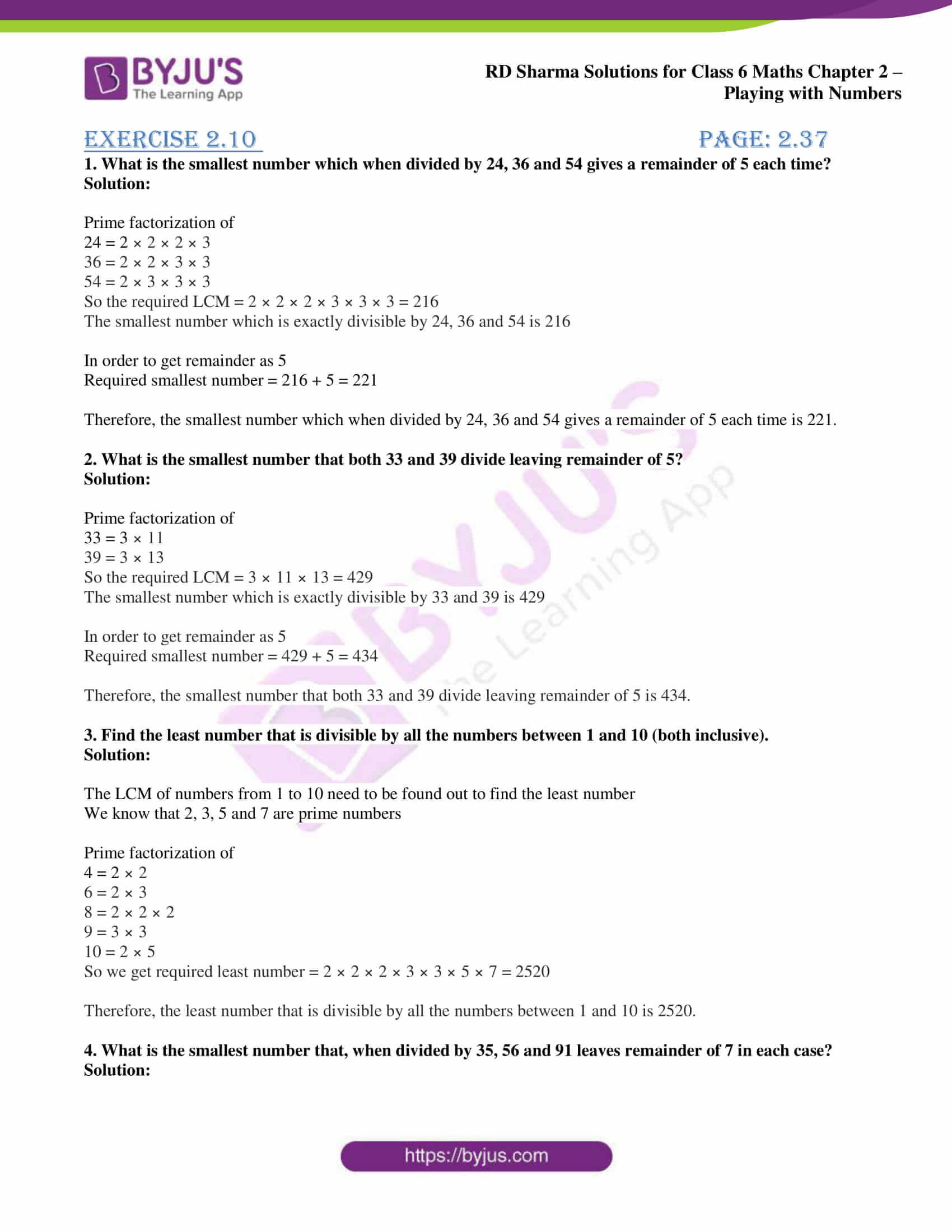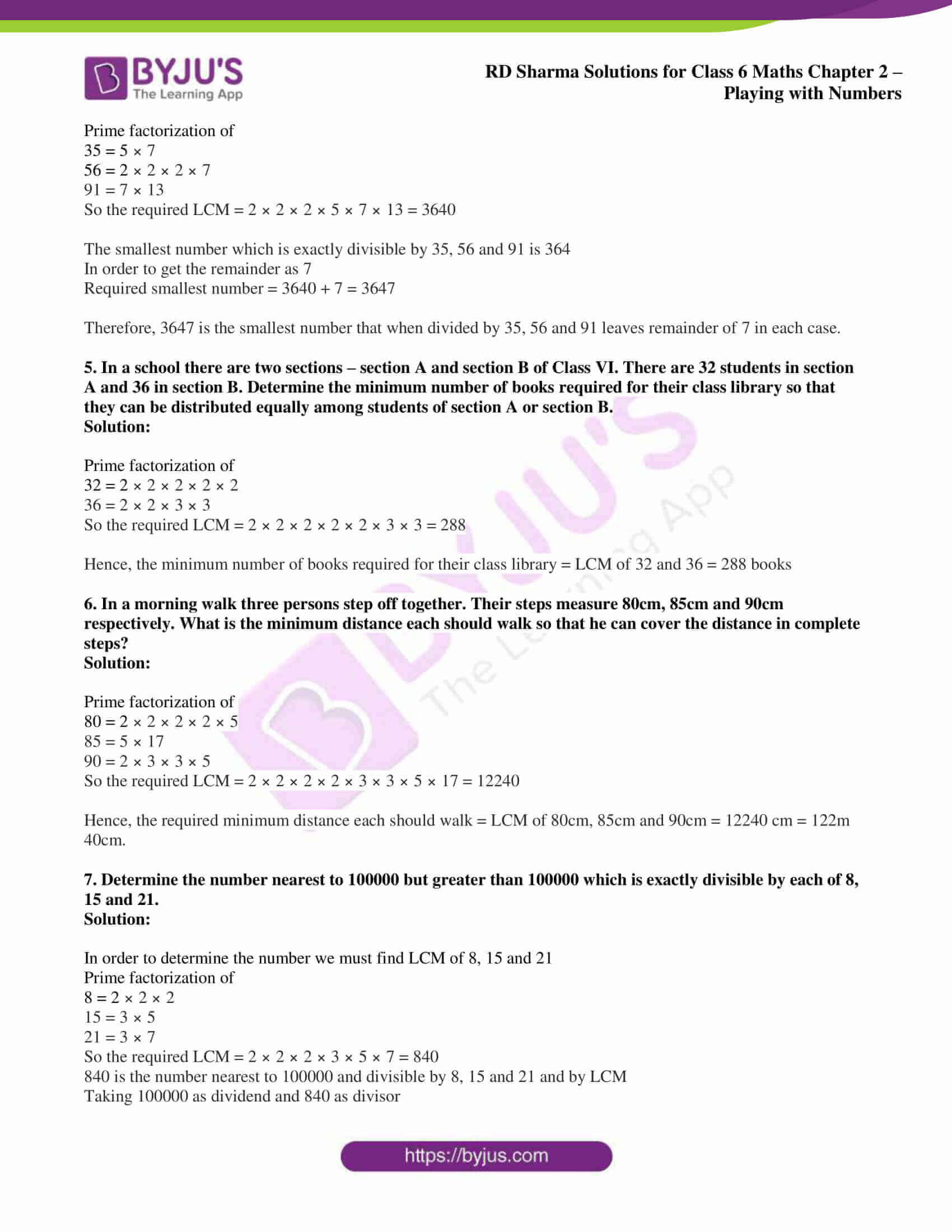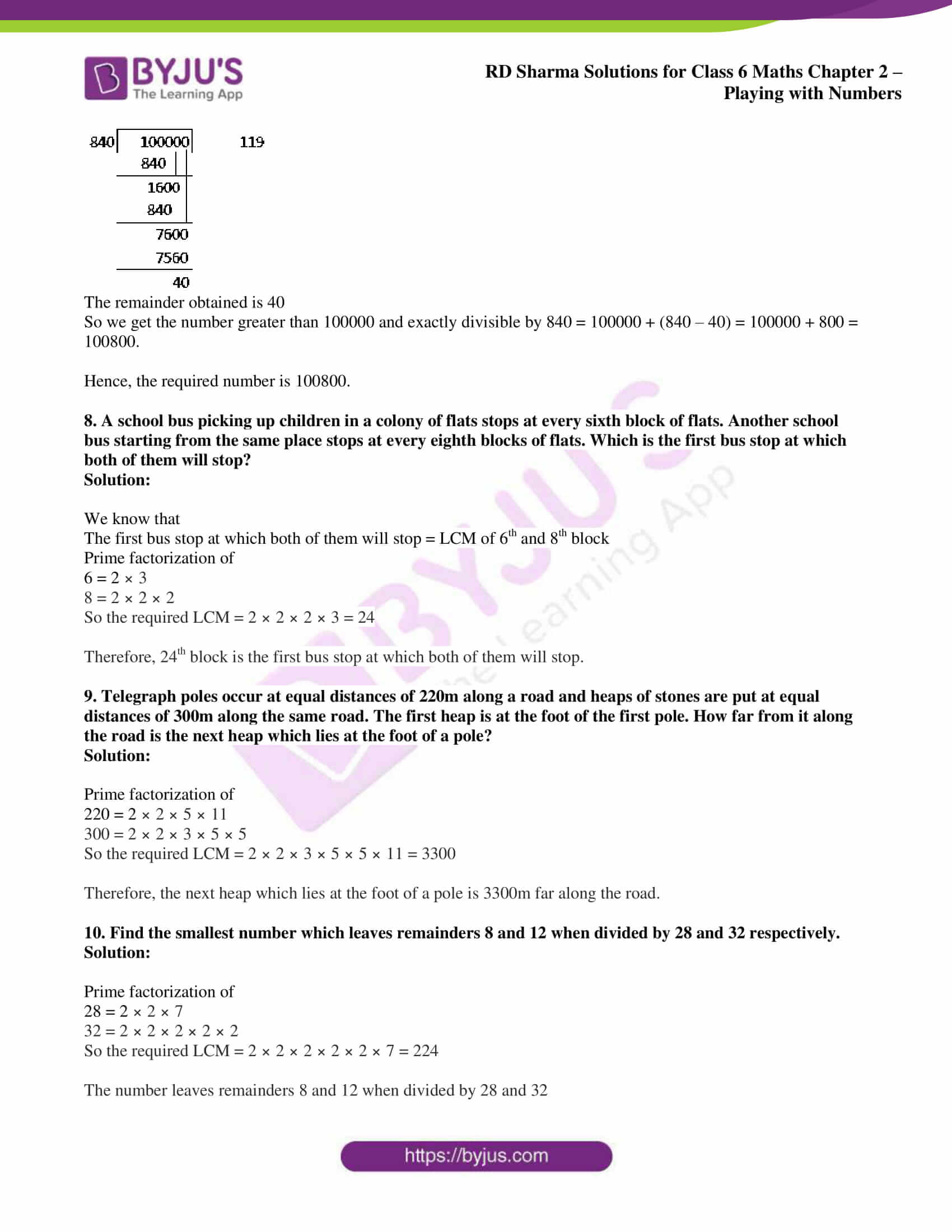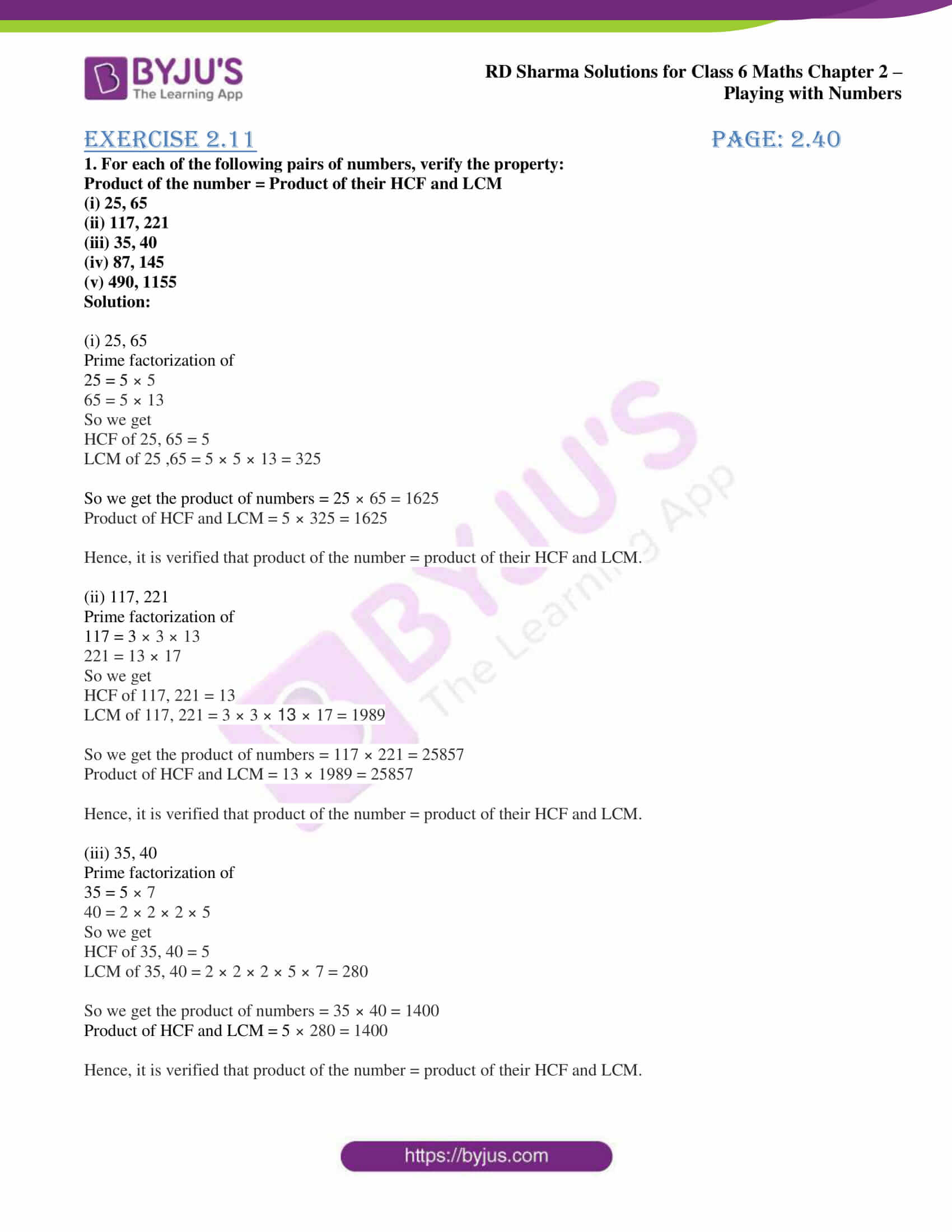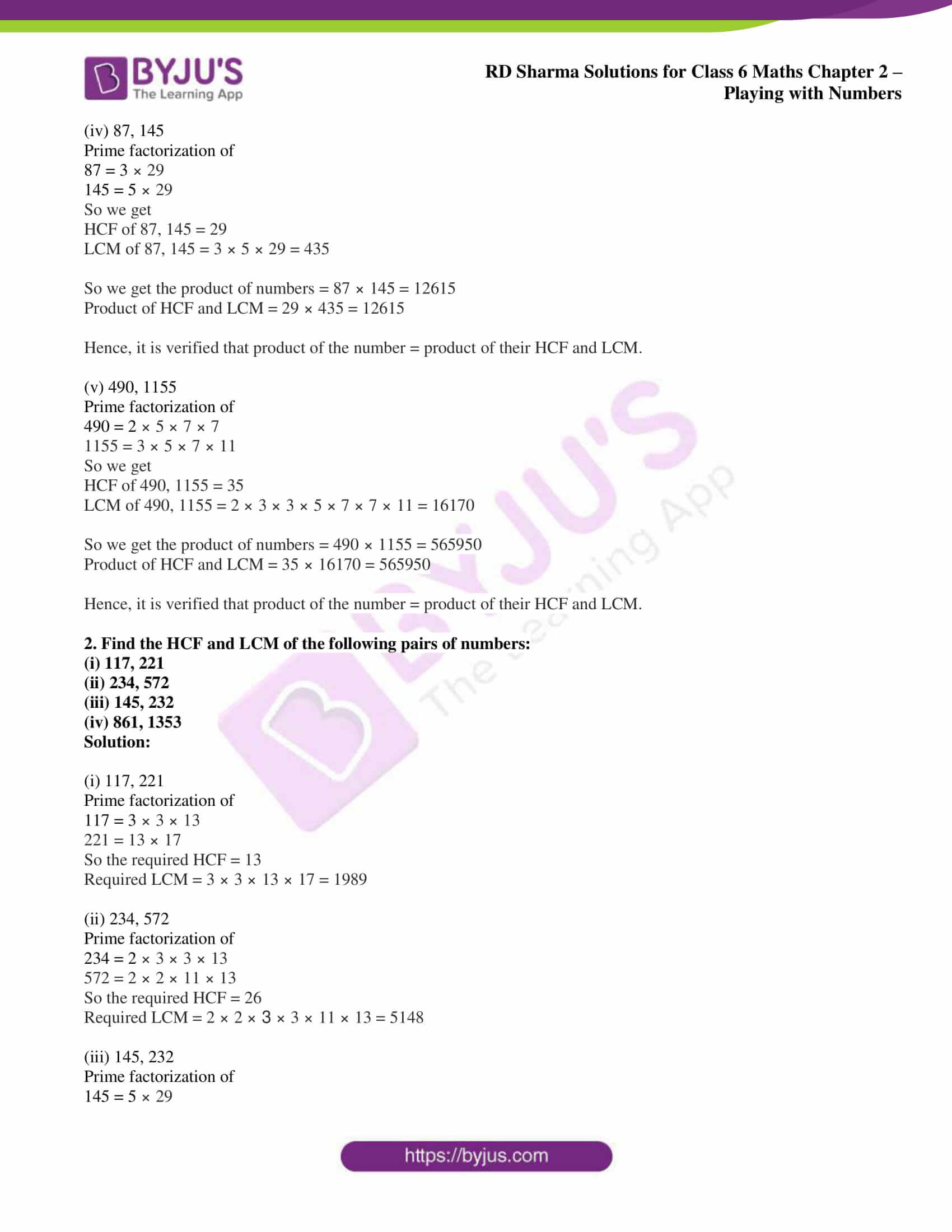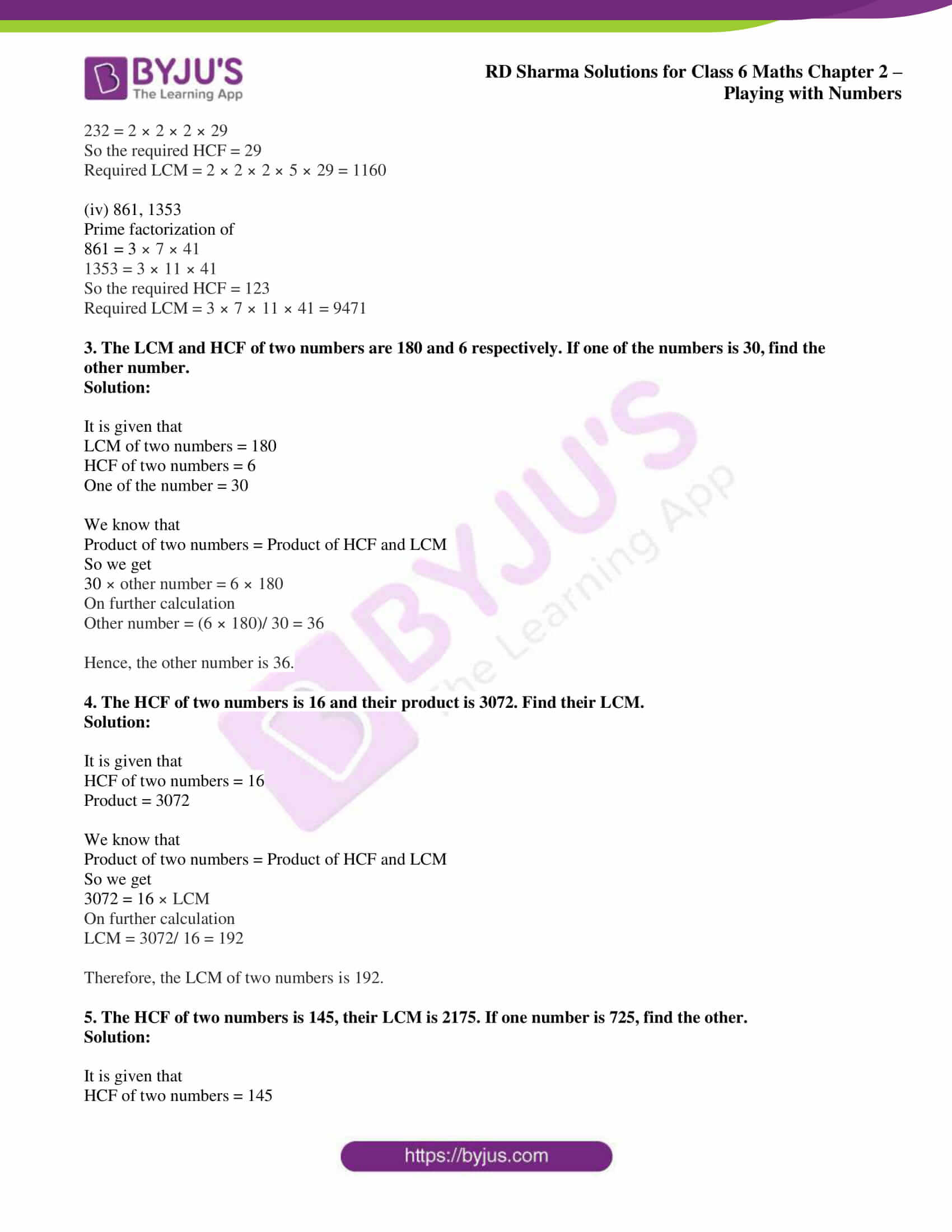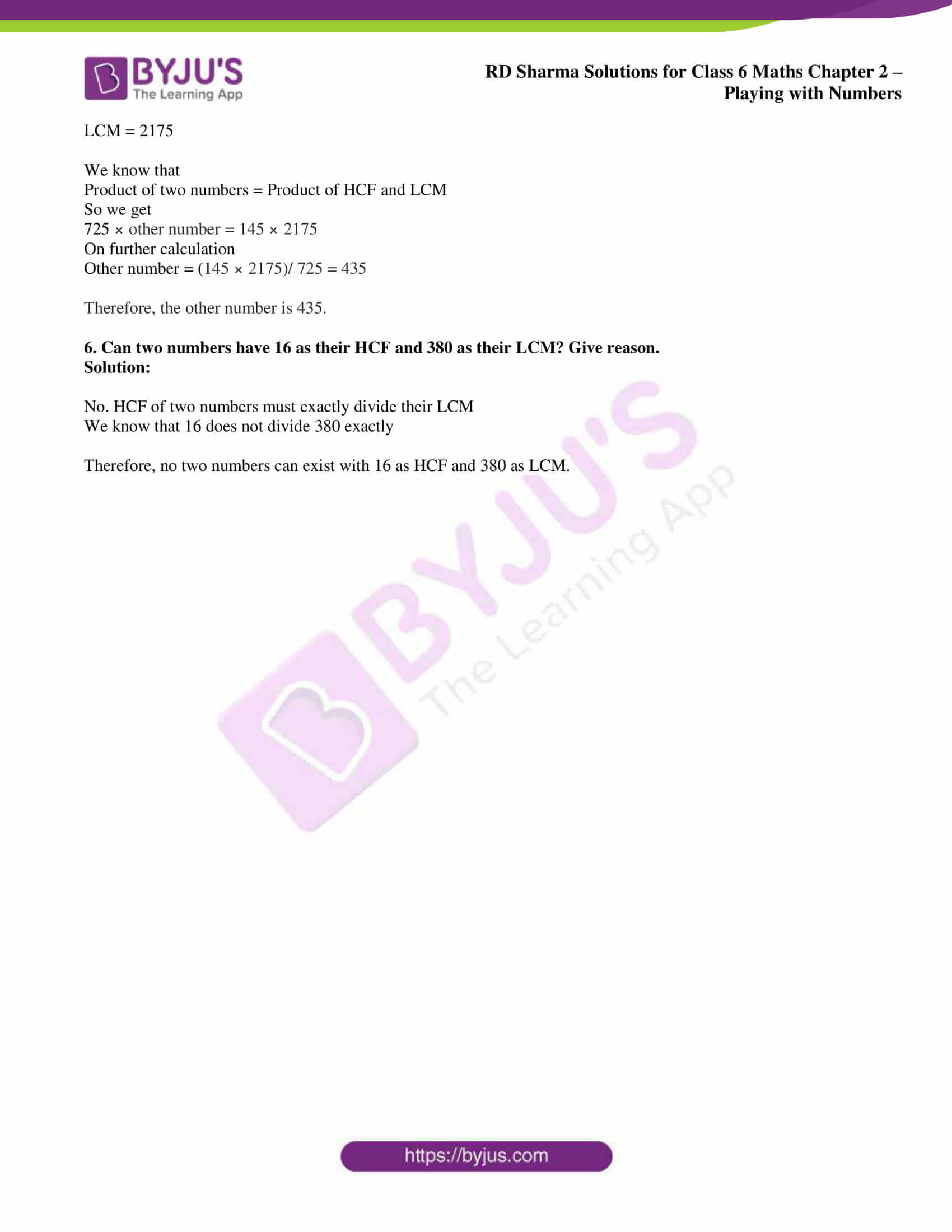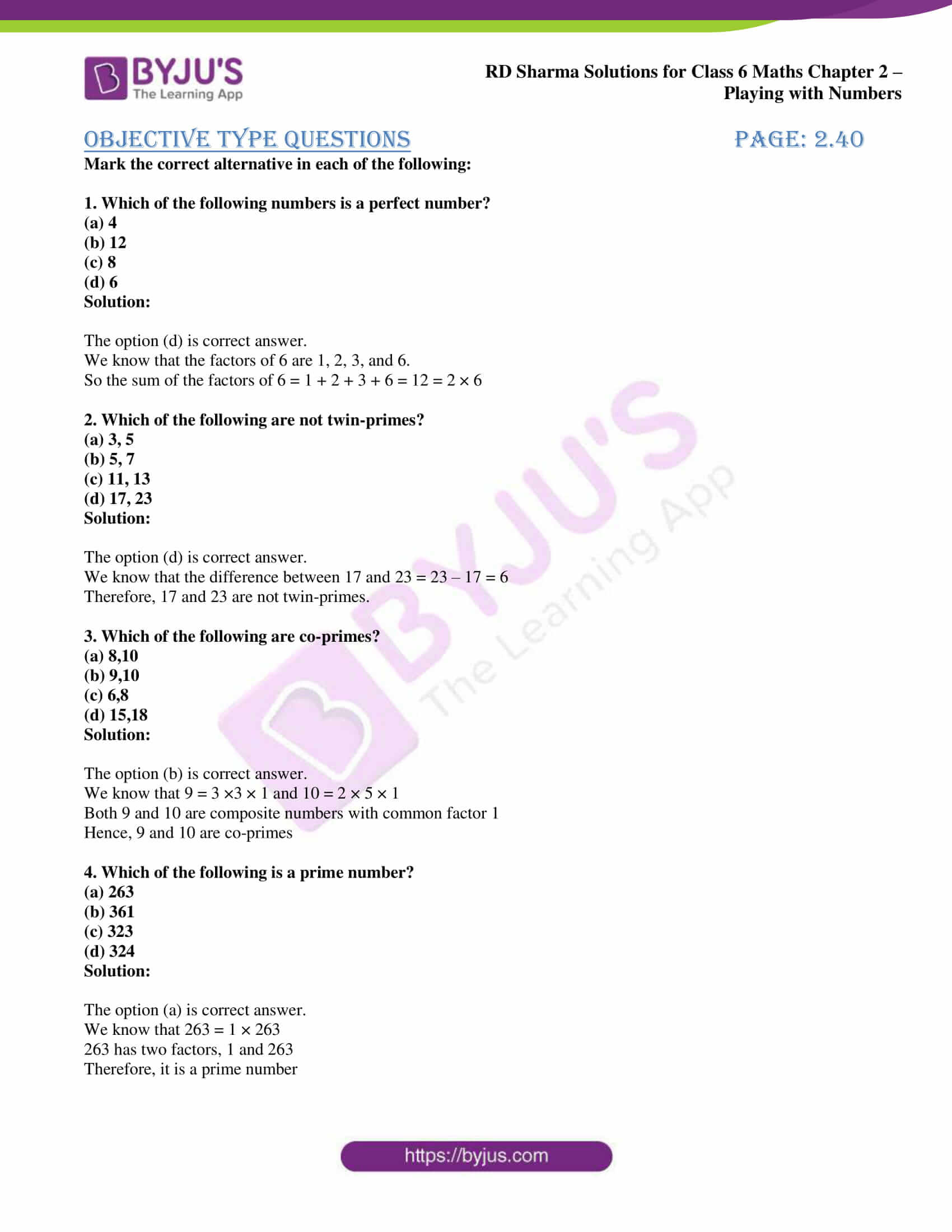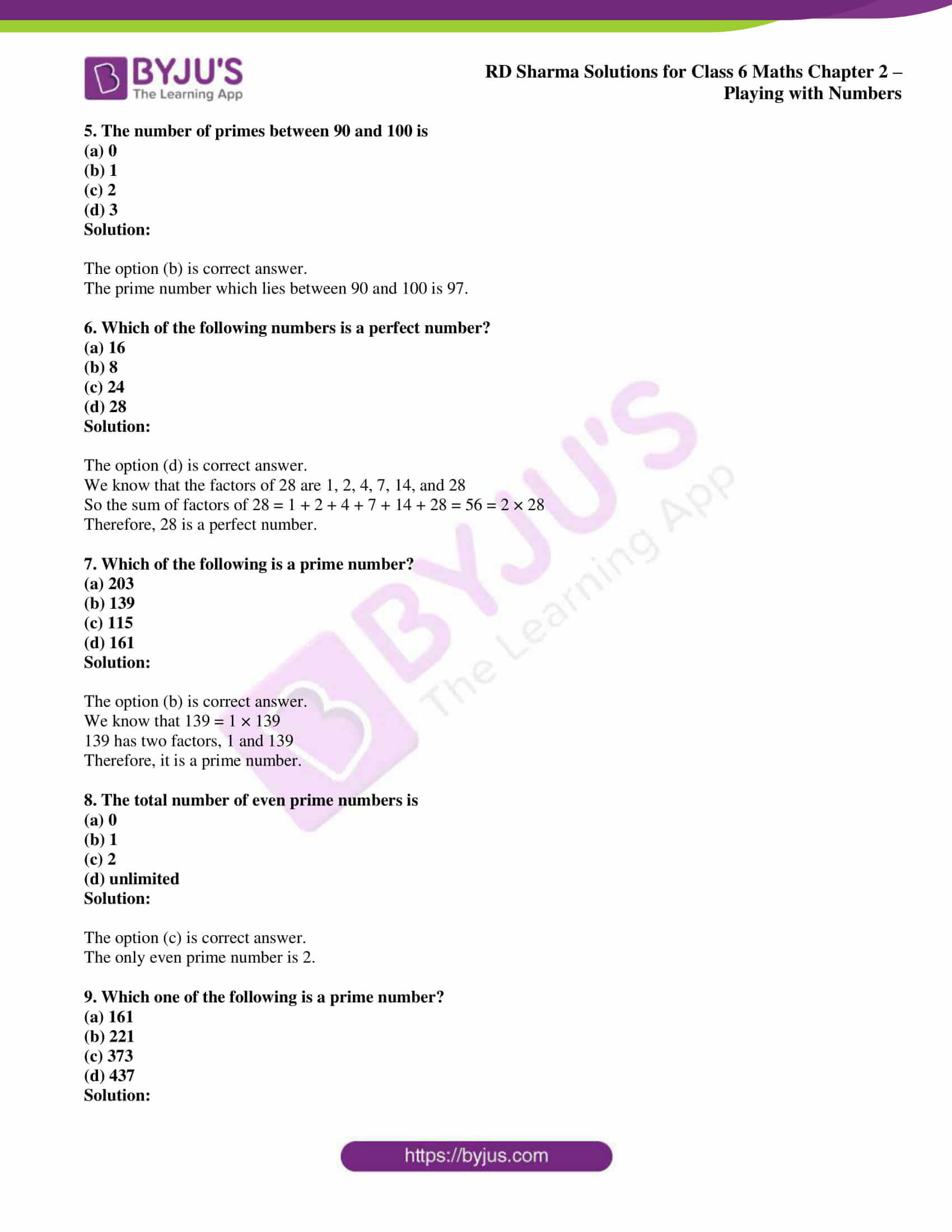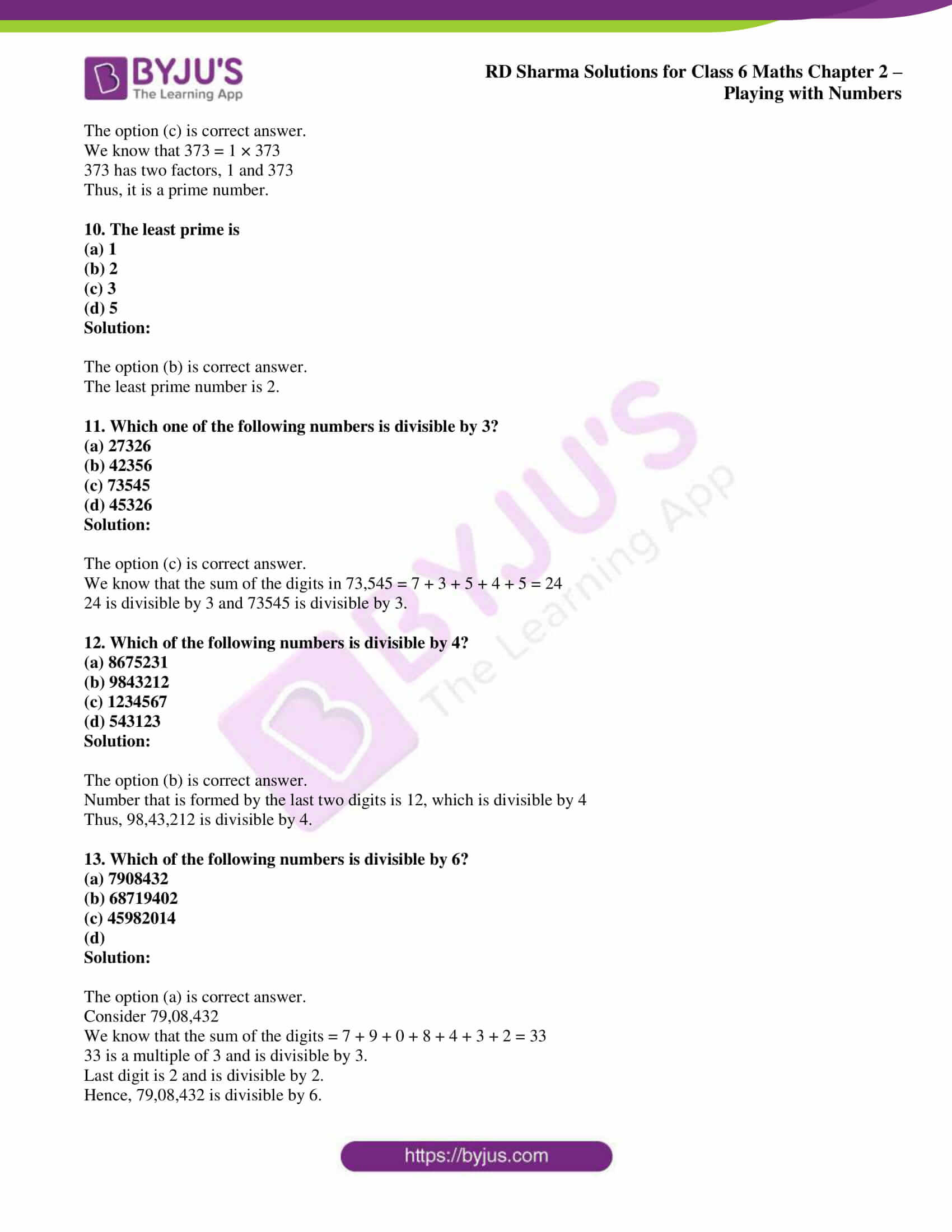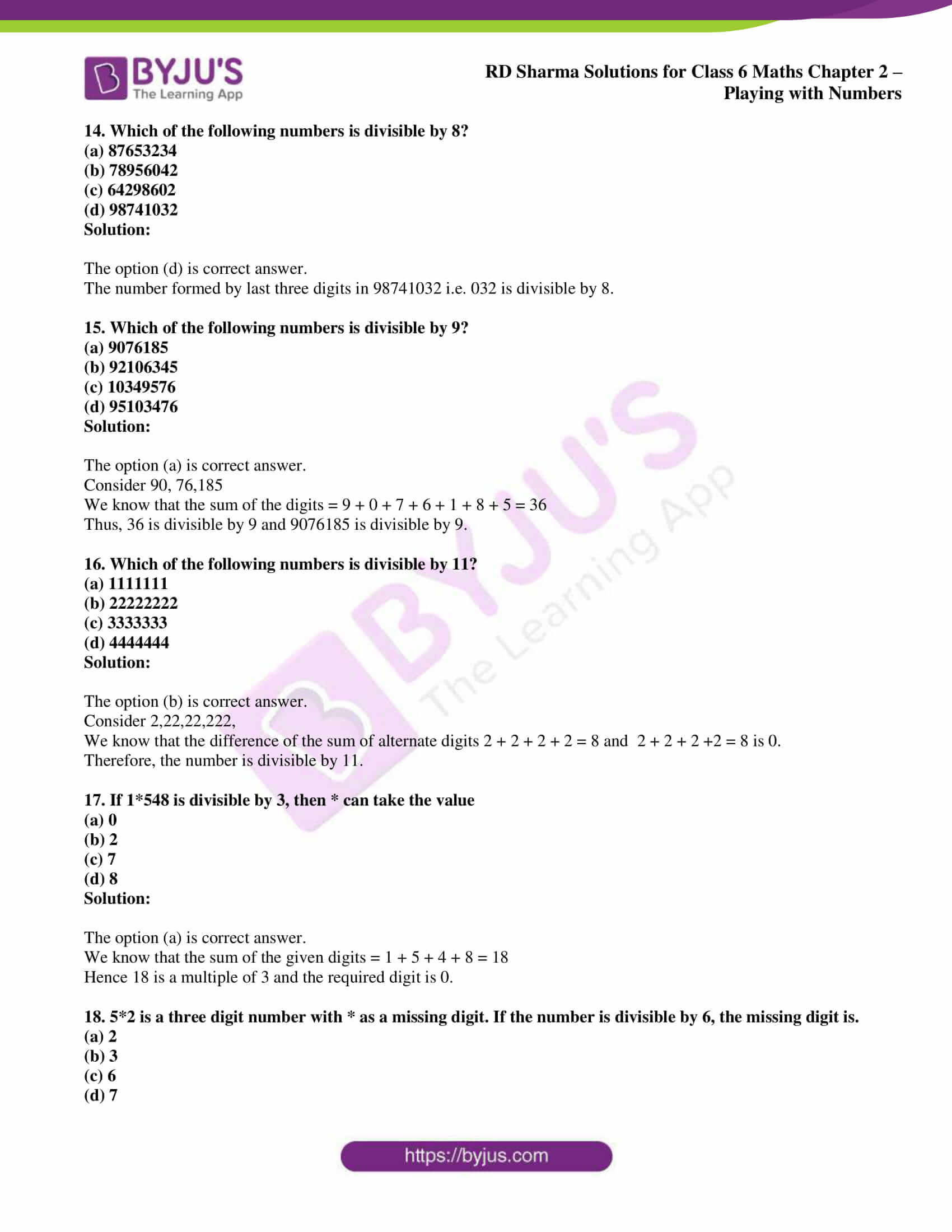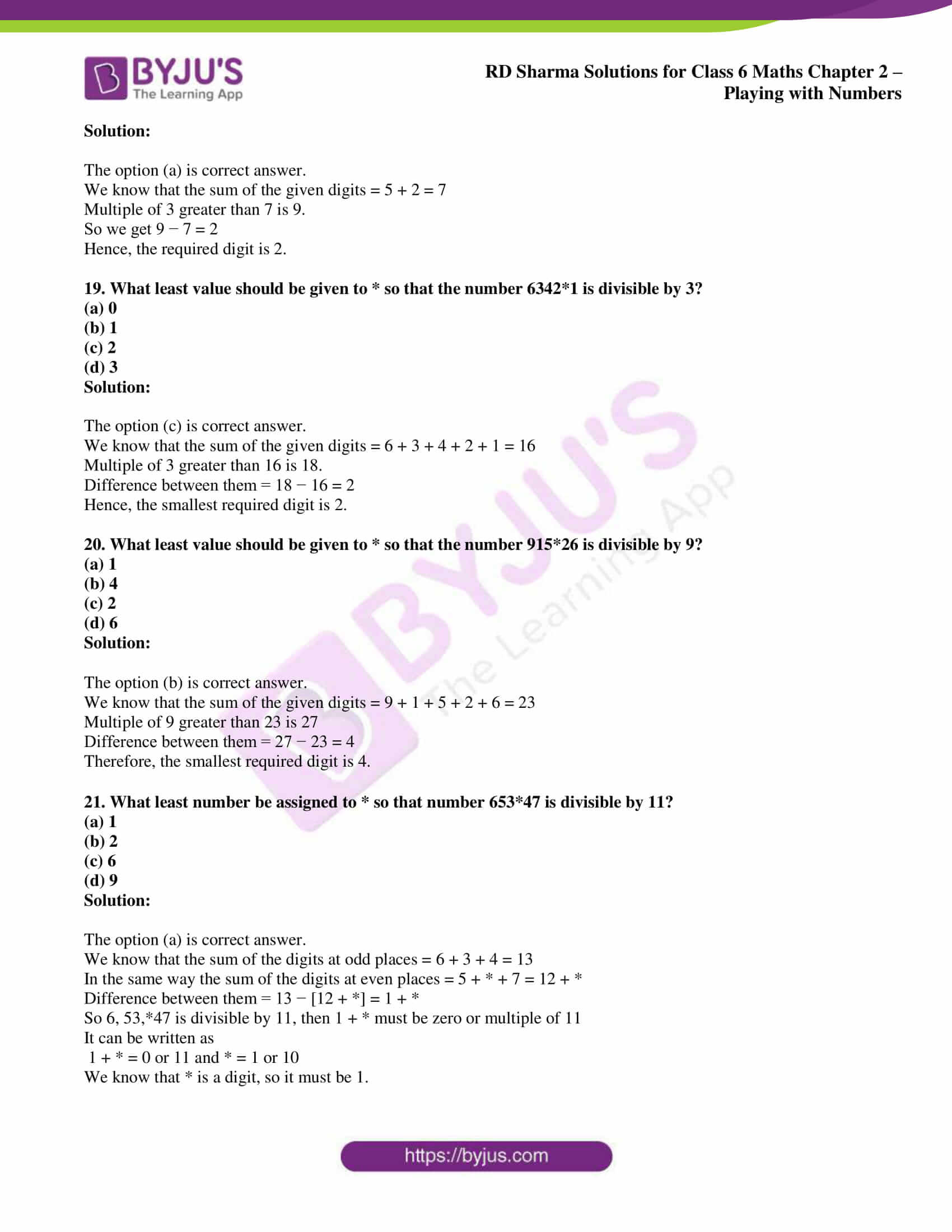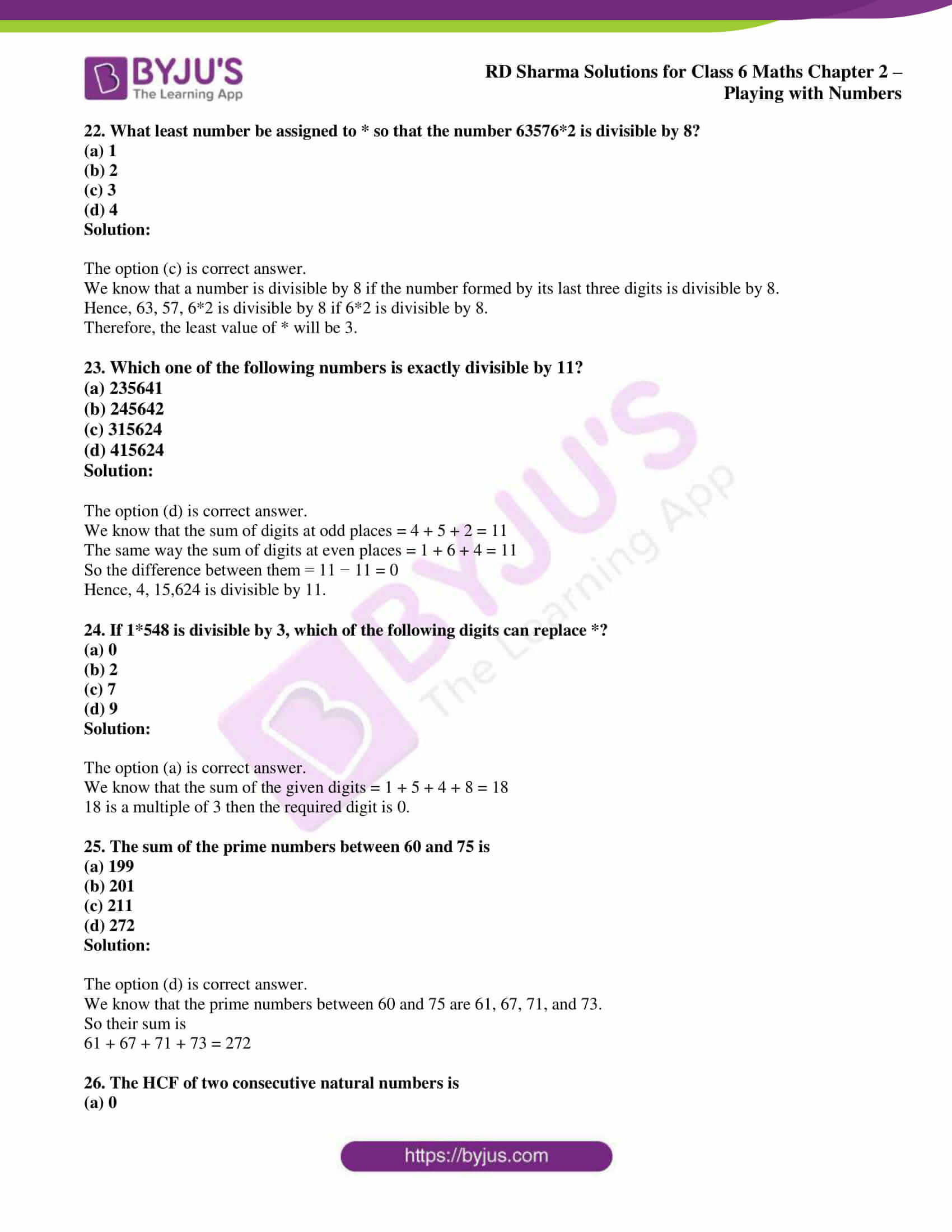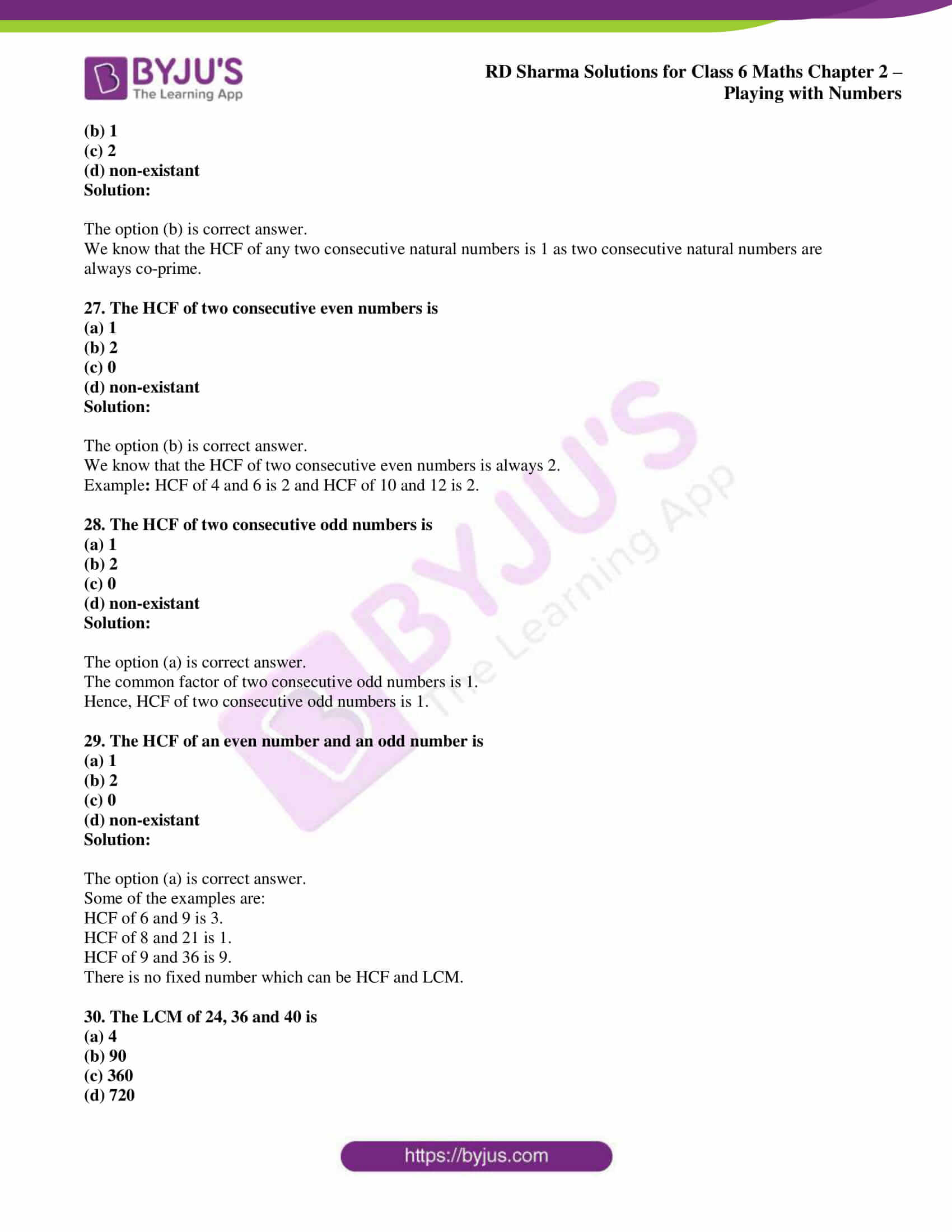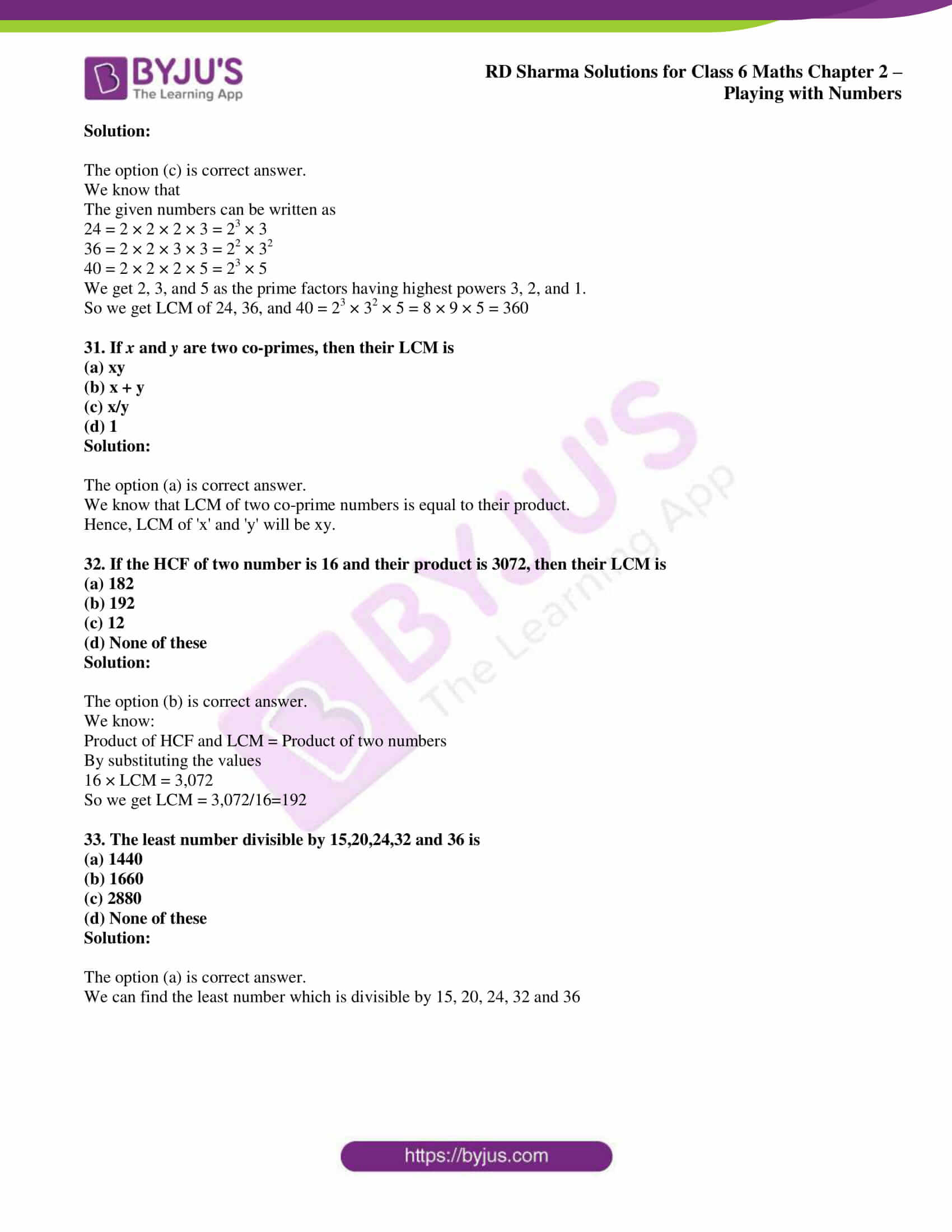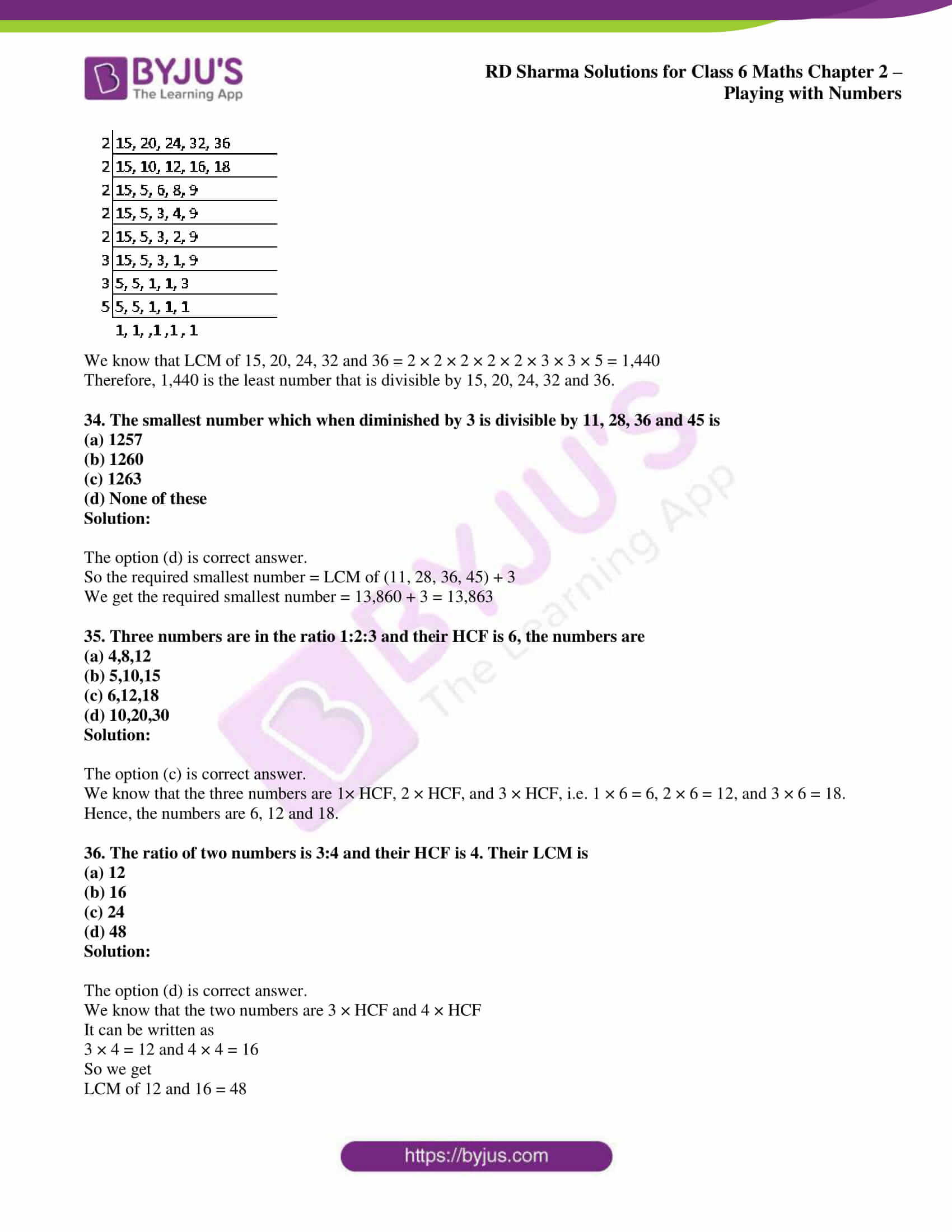## Access answers to Maths RD Sharma Solutions for Class 6 Chapter 2: Playing with Numbers

### Exercise 2.1 page: 2.5

1. Define:

(i) factor

(ii) multiple

Give four examples each.

Solution:

(i) A factor of a number is an exact divisor of that number.

Example:

1. 5 and 2 are factors of 10 i.e. 5 × 2 = 10

2. 4 and 3 are factors of 12 i.e. 4 × 3 = 12

3. 4 and 2 are factors of 8 i.e. 4 × 2 = 8

4. 2 and 6 are factors of 12 i.e. 2 × 6 = 12

(ii) A multiple of a number is a number obtained by multiplying it by a natural number.

Example:

1. 10 is a multiple of 2 i.e. 2 × 5 = 10

2. 12 is a multiple of 4 i.e. 4 × 3 = 12

3. 8 is a multiple of 2 i.e. 2 × 4 = 8

4. 21 is a multiple of 3 i.e. 3 × 7 = 21

2. Write all factors of each of the following numbers:

(i) 60

(ii) 76

(iii) 125

(iv) 729

Solution:

(i) 60

It can be written as

1 × 60 = 60

2 × 30 = 60

3 × 20 = 60

4 × 15 = 60

5 × 12 = 60

6 × 10 = 60

Therefore, the factors of 60 are 1, 2, 3, 4, 5, 6, 10, 12, 15, 20, 30 and 60.

(ii) 76

It can be written as

1 × 76 = 76

2 × 38 = 76

4 × 19 = 76

Therefore, the factors of 76 are 1, 2, 4, 19, 38 and 76.

(iii) 125

It can be written as

1 × 125 = 125

5 × 25 = 125

Therefore, the factors of 125 are 1, 5, 25 and 125.

(iv) 729

It can be written as

1 × 729 = 729

3 × 243 = 729

9 × 81 = 729

27 × 27 = 729

Therefore, the factors of 729 are 1, 3, 9, 27, 81, 243 and 729.

3. Write first five multiples of each of the following numbers:

(i) 25

(ii) 35

(iii) 45

(iv) 40

Solution:

(i) 25

It can be written as

1 × 25 = 25

2 × 25 = 50

3 × 25 = 75

4 × 25 = 100

5 × 25 = 125

Therefore, the first five multiples of 25 are 25, 50, 75, 100 and 125.

(ii) 35

It can be written as

1 × 35 = 35

2 × 35 = 70

3 × 35 = 105

4 × 35 = 140

5 × 35 = 175

Therefore, the first five multiples of 35 are 35, 70, 105, 140 and 175.

(iii) 45

It can be written as

1 × 45 = 45

2 × 45 = 90

3 × 45 = 135

4 × 45 = 180

5 × 45 = 225

Therefore, the first five multiples of 45 are 45, 90, 135, 180 and 225.

(iv) 40

It can be written as

1 × 40 = 40

2 × 40 = 80

3 × 40 = 120

4 × 40 = 160

5 × 40 = 200

Therefore, the first five multiples of 40 are 40, 80, 120, 160 and 200.

4. Which of the following numbers have 15 as their factor?

(i) 15625

(ii) 123015

Solution:

(i) 15625

We know that 15 is not a factor of 15625 as it is not a divisor of 15625.

(ii) 123015

We know that 15 is a factor of 123015 as it is a divisor of 123015 because 8201 × 15 = 123015.

5. Which of the following numbers are divisible by 21?

(i) 21063

(ii) 20163

Solution:

(i) 21063

We know that the sum of digits = 2 + 1 + 0 + 6 + 3 = 12 which is divisible by 3

So 21063 is divisible by 3

A number is divisible by 7 if the difference between two times ones digit and the number formed by other digit is 0 or multiple of 7.

We get

2106 – (2 × 3) = 2100 which is a multiple of 7.

Therefore, 21063 is divisible by 21.

(ii) 20163

We know that the sum of digits = 2 + 0 + 1 + 6 + 3 = 12 which is divisible by 3

So 20163 is divisible by 3

A number is divisible by 7 if the difference between two times ones digit and the number formed by other digit is 0 or multiple of 7.

We get

2016 – (2 × 3) = 2010 which is not a multiple of 7.

Therefore, 20163 is not divisible by 21.

6. Without actual division show that 11 is a factor of each of the following numbers:

(i) 1111

(ii) 11011

(iii) 110011

(iv) 1100011

Solution:

(i) 1111

Sum of digits at the odd places = 1 + 1 = 2

Sum of digits at the even places = 1 + 1 = 2

So the difference between the two sums = 2 – 2 = 0

Hence, 1111 is divisible by 11 as the difference between the two sums is zero.

(ii) 11011

Sum of digits at the odd places = 1 + 0 + 1 = 2

Sum of digits at the even places = 1 + 1 = 2

So the difference between the two sums = 2 – 2 = 0

Hence, 11011 is divisible by 11 as the difference between the two sums is zero.

(iii) 110011

Sum of digits at the odd places = 1 + 0 + 1 = 2

Sum of digits at the even places = 1 + 0 + 1 = 2

So the difference between the two sums = 2 – 2 = 0

Hence, 110011 is divisible by 11 as the difference between the two sums is zero.

(iv) 1100011

Sum of digits at the odd places = 1 + 0 + 0 + 1 = 2

Sum of digits at the even places = 1 + 0 + 1 = 2

So the difference between the two sums = 2 – 2 = 0

Hence, 1100011 is divisible by 11 as the difference between the two sums is zero.

7. Without actual division show that each of the following numbers is divisible by 5:

(i) 55

(ii) 555

(iii) 5555

(iv) 50005

Solution:

(i) 55

The units digit in 55 is 5.

Therefore, 55 is divisible by 5.

(ii) 555

The units digit in 555 is 5.

Therefore, 555 is divisible by 5.

(iii) 5555

The units digit in 5555 is 5.

Therefore, 5555 is divisible by 5.

(iv) 50005

The units digit in 50005 is 5.

Therefore, 50005 is divisible by 5.

8. Is there any natural number having no factor at all?

Solution:

No. All the natural numbers are a factor of itself.

9. Find numbers between 1 and 100 having exactly three factors.

Solution:

We know that the numbers between 1 and 100 which have exactly three factors are 4, 9, 25 and 49.

Factors of 4 are 1, 2 and 4.

Factors of 9 are 1, 3 and 9.

Factors of 25 are 1, 5 and 25.

Factors of 49 are 1, 7 and 49.

10. Sort out even and odd numbers:

(i) 42

(ii) 89

(iii) 144

(iv) 321

Solution:

We know that

The numbers which are divisible by 2 are even and those which are not divisible by 2 are odd numbers.

So we get

42 and 144 are even numbers and 89 and 321 are odd numbers.

### Exercise 2.2 page: 2.7

1. Find the common factors of:

(i) 15 and 25

(ii) 35 and 50

(iii) 20 and 28

Solution:

(i) 15 and 25

We know that

1 × 15 = 15

3 × 5 = 15

Factors of 15 are 1, 3, 5 and 15.

We know that

1 × 25 = 25

5 × 5 = 25

Factors of 25 are 1, 5 and 25.

Therefore, the common factors of 15 and 25 are 1 and 5.

(ii) 35 and 50

We know that

1 × 35 = 35

5 × 7 = 35

Factors of 35 are 1, 5, 7 and 35.

We know that

1 × 50 = 50

2 × 25 = 50

5 × 10 = 50

Factors of 50 are 1, 2, 5, 10, 25 and 50.

Therefore, the common factors of 35 and 50 are 1 and 5.

(iii) 20 and 28

We know that

1 × 20 = 20

2 × 10 = 20

4 × 5 = 20

Factors of 20 are 1, 2, 4, 5, 10 and 20.

We know that

1 × 28 = 28

2 × 14 = 28

7 × 4 = 28

Factors of 28 are 1, 2, 4, 7, 14 and 28.

Therefore, the common factors of 20 and 28 are 1, 2 and 4.

2. Find the common factors of:

(i) 5, 15 and 25

(ii) 2, 6 and 8

Solution:

(i) 5, 15 and 25

We know that

For 5

1 × 5 = 5

Factors of 5 are 1 and 5

For 15

1 × 15 = 15

3 × 5 = 15

Factors of 15 are 1, 3, 5 and 15

For 25

1 × 25 = 25

5 × 5 = 25

Factors of 25 are 1, 5 and 25

Therefore, the common factors of 5, 15 and 25 are 1 and 5.

(ii) 2, 6 and 8

We know that

For 2

1 × 2 = 2

Factors of 2 are 1 and 2

For 6

1 × 6 = 6

2 × 3 = 6

Factors of 6 are 1, 2, 3 and 6

For 8

1 × 8 = 8

2 × 4 = 8

Factors of 8 are 1, 2, 4 and 8

Therefore, the common factors of 2, 6 and 8 are 1 and 2.

3. Find first three common multiples of 6 and 8.

Solution:

We know that the multiples of 6 are

6, 12, 18, 24, 30, 36, 42, 48, 54, 60, 66, 72 …..

Multiples of 8 are

8, 16, 24, 32, 40, 48, 56, 64, 72, 80 ……

Hence, the first three common multiples of 6 and 8 are 24, 48 and 72.

4. Find first two common multiples of 12 and 18.

Solution:

We know that multiples of 12 are

12, 24, 36, 48, 60, 72, 84, 96, 108, 120 …..

Multiples of 18 are

18, 36, 54, 72, 90, 108, 126, 144, 162, 180 ….

Hence, the first two common multiples of 12 and 18 are 36 and 72.

5. A number is divisible by both 7 and 16. By which other number will that number be always divisible?

Solution:

It is given that a number is divisible by both 7 and 16.

We know that the factors of 7 are 1 and 7 and the factors of 16 are 1, 2, 4, 8 and 16

Hence, the common factor of 7 and 16 is 1 and the number is divisible by 1.

6. A number is divisible by 24. By what other numbers will that number be divisible?

Solution:

It is given that a number is divisible by 24

We know that the factors of 24 are

1, 2, 3, 4, 6, 8, 12 and 24

Therefore, the number is divisible by 1, 2, 3, 4, 6, 8 and 12.

### Exercise 2.3 page: 2.10

1. What are prime numbers? List all primes between 1 and 30.

Solution:

A number is called a prime number if it has no factor other than 1 and the number itself.

For example 2, 3, 5, 7, 11 and 13 are prime numbers.

The list of primes between 1 and 30 are

2, 3, 5, 7, 11, 13, 17, 19, 23 and 29.

2. Write all prime numbers between:

(i) 10 and 50

(ii) 70 and 90

(iii) 40 and 85

(iv) 60 and 100

Solution:

(i) The prime numbers between 10 and 50 are

11, 13, 17, 19, 23, 29, 31, 37, 41, 43 and 47.

(ii) The prime numbers between 70 and 90 are

71, 73, 79, 83 and 89.

(iii) The prime numbers between 40 and 85 are

41, 43, 47, 53, 59, 61, 67, 71, 73, 79 and 83.

(iv) The prime numbers between 60 and 100 are

61, 67, 71, 73, 79, 83, 89 and 97.

3. What is the smallest prime number? Is it an even number?

Solution:

2 is the smallest prime number.

We know that 2 is an even prime number since it is divisible by 2.

4. What is the smallest odd prime? Is every odd number a prime number? If not, give an example of an odd number which is not prime.

Solution:

3 is the smallest odd prime number.

No, every odd number is not a prime number.

Example: 9 is an odd number having factors 1, 3 and 9 and is not a prime number.

5. What are composite numbers? Can a composite number be odd? If yes, write the smallest odd composite number.

Solution:

A number is called a composite number if it has at least one factor other than 1 and the number itself.

Example: 4, 6, 8, 9, 10 and 15 are composite numbers.

Yes, a composite number can be odd and 9 is the smallest odd number.

6. What are twin-primes? Write all pairs of twin-primes between 50 and 100.

Solution:

Two prime numbers are known as twin-primes if there is only one composite number between them.

Example: 3, 5; 5, 7; 11, 13; 17, 19; 29, 31; 41, 43; 59, 61 and 71, 73 are the pairs of twin-primes between 1 and 100.

The pairs of twin-primes between 50 and 100 are

59, 61 and 71, 73.

7. What are co-primes? Give examples of five pairs of co-primes. Are co-primes always prime? If no, illustrate your answer by an example.

Solution:

Two numbers are said to be co-prime if they do not have a common factor other than 1.

2, 3; 3, 4; 4, 5; 5, 6; 6, 7 are the examples of five pairs of co-primes.

No. 15, 16 are co-prime but both of the numbers are not prime.

8. Which of the following pairs are always co-prime?

(i) two prime numbers

(ii) one prime and one composite number

(iii) two composite numbers

Solution:

(i) Two prime numbers are always co-prime to each other.

For example: The numbers 7 and 11 are co-prime to each other.

(ii) One prime and one composite number are not always co-prime.

For example: The numbers 3 and 21 are not co-prime to each other.

(iii) Two composite numbers are not always co-prime to each other.

For example: 4 and 6 are not co-prime to each other.

9. Express each of the following as a sum of two or more primes:

(i) 13

(ii) 130

(iii) 180

Solution:

(i) We know that

The number 13 can be written as

13 = 3 + 3 + 7 which is the sum of two or more primes.

(ii) We know that

The number 130 can be written as

130 = 59 + 71 which is the sum of two or more primes.

(iii) We know that

The number 180 can be written as

180 = 79 + 101 which is the sum of two or more primes.

10. Express each of the following numbers as the sum of two odd primes:

(i) 36

(ii) 42

(iii) 84

Solution:

(i) We know that

The number 36 can be written as

36 = 7 + 29 which is the sum of two odd primes

(ii) We know that

The number 42 can be written as

42 = 5 + 37 which is the sum of two odd primes

(iii) We know that

The number 84 can be written as

84 = 17 + 67 which is the sum of two odd primes

11. Express each of the following numbers as the sum of three odd prime numbers:

(i) 31

(ii) 35

(iii) 49

Solution:

(i) We know that

The number 31 can be written as

31 = 5 + 7 + 19 which is the sum of three odd prime numbers

(ii) We know that

The number 35 can be written as

35 = 5 + 7 + 23 which is the sum of three odd prime numbers

(iii) We know that

The number 49 can be written as

49 = 3 + 5 + 41 which is the sum of three odd prime numbers

12. Express each of the following numbers as the sum of twin primes:

(i) 36

(ii) 84

(iii) 120

Solution:

(i) We know that

The number 36 can be written as

36 = 17 + 19 which is the sum of twin primes

(ii) We know that

The number 84 can be written as

84 = 41 + 43 which is the sum of twin primes

(iii) We know that

The number 120 can be written as

120 = 59 + 61 which is the sum of twin primes

13. Find the possible missing twins for the following numbers so that they become twin primes:

(i) 29

(ii) 89

(iii) 101

Solution:

(i) We know that 27 and 31 are the possible missing twins for 29.

31 is a prime number and 27 is not a prime number

Therefore, 31 is the missing twin.

(ii) We know that 87 and 91 are the possible missing twins for 89

Both the numbers are not prime.

Therefore, 89 has no twin.

(iii) We know that 99 and 103 are the possible missing twins for 101

103 is a prime number and 99 is not a prime number

Therefore, 103 is the missing twin.

14. A list consists of the following pairs of numbers:

(i) 51, 53; 55, 57; 59, 61; 63, 65; 67, 69; 71, 73

Categorize them as pairs of

(i) co-primes

(ii) primes

(iii) composites

Solution:

(i) Two numbers are said to be co-prime if they do not have a common factor other than 1.

Therefore, all the given pairs of numbers are co-primes.

(ii) A number is called a prime number if it has no factor other than 1 and the number itself.

Therefore, 59, 61; 71, 73 are pairs of prime numbers.

(iii) A number is called a composite number if it has at least one factor other than 1 and the number itself.

Therefore, 55, 57; 63, 65 are pairs of composite numbers.

15. For a number, greater than 10, to be prime what may be the possible digit in the units place?

Solution:

For a number, greater than 10, the possible digit in the units place to be prime can be 1, 3, 7 or 9.

For example: 11, 13, 17 and 19 are prime numbers which are greater than 10.

16. Write seven consecutive numbers less than 100 so that there is no prime number between them.

Solution:

The seven consecutive composite numbers less than 100 so that there is no prime number between them are

90, 91, 92, 93, 94, 95 and 96.

17. State true (T) or false (F):

(i) The sum of primes cannot be a prime.

(ii) The product of primes cannot be a prime.

(iii) An even number is composite.

(iv) Two consecutive numbers cannot be both primes.

(v) Odd numbers cannot be composite.

(vi) Odd numbers cannot be written as sum of primes.

(vii) A number and its successor are always co-primes.

Solution:

(i) False. 2 + 3 = 5 is a prime number.

(ii) True. The product of primes is a composite number.

(iii) False. Even number 2 is not composite.

(iv) False. The numbers 2 and 3 are consecutive and prime numbers.

(v) False. 9 is an odd number and is composite having factors 1, 3 and 9.

(vi) False. 9 is an odd number and the sum of prime number 7 + 2 = 9.

(vii) True. A number and its successor have only one common factor.

18. Fill in the blanks in the following:

(i) A number having only two factors is called a ……….

(ii) A number having more than two factors is called a …………

(iii) 1 is neither ………. Nor ………

(iv) The smallest prime number is ………..

(v) The smallest composite number is ……….

Solution:

(i) A number having only two factors is called a prime number.

(ii) A number having more than two factors is called a composite number.

(iii) 1 is neither prime nor composite.

(iv) The smallest prime number is 2.

(v) The smallest composite number is 4.

### Exercise 2.4 page: 2.15

1. In which of the following expressions, prime factorization has been done?

(i) 24 = 2 × 3 × 4

(ii) 56 = 1 × 7 × 2 × 2 × 2

(iii) 70 = 2 × 5 × 7

(iv) 54 = 2 × 3 × 9

Solution:

(i) We know that

24 = 2 × 3 × 4 is not a prime factorization since 4 is not a prime number.

(ii) We know that

56 = 1 × 7 × 2 × 2 × 2 is not a prime factorization since 1 is not a prime number.

(iii) We know that

70 = 2 × 5 × 7 is a prime factorization since 2, 5 and 7 are prime numbers.

(iv) We know that

54 = 2 × 3 × 9 is not a prime factorization since 9 is not a prime number.

2. Determine prime factorization of each of the following numbers:

(i) 216

(ii) 420

(iii) 468

(iv) 945

(v) 7325

(vi) 13915

Solution:

(i) 216

We know that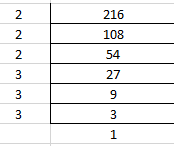Hence, the prime factorization of 216 is 2 × 2 × 2 × 3 × 3 × 3.

(ii) 420

We know that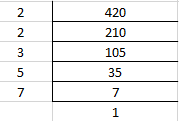Hence, the prime factorization of 420 is 2 × 2 × 3 × 5 × 7.

(iii) 468

We know that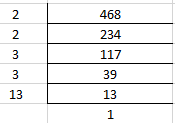Hence, the prime factorization of 468 is 2 × 2 × 3 × 3 × 13.

(iv) 945

We know that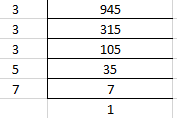Hence, the prime factorization of 945 is 3 × 3 × 3 × 5 × 7.

(v) 7325

We know that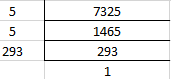Hence, the prime factorization of 7325 is 5 × 5 × 293.

(vi) 13915

We know that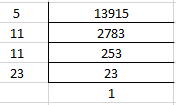Hence, the prime factorization of 13915 is 5 × 11 × 11 × 23.

3. Write the smallest 4-digit number and express it as a product of primes.

Solution:

1000 is the smallest 4-digit number.

We know that

1000 = 2 × 500

= 2 × 2 × 250

So we get

1000 = 2 × 2 × 2 × 125

It can be further expanded as

1000 = 2 × 2 × 2 × 5 × 25

We get

1000 = 2 × 2 × 2 × 5 × 5 × 5

Therefore, the smallest 4-digit number is 1000 and can be expressed as 2 × 2 × 2 × 5 × 5 × 5.

4. Write the largest 4-digit number and give its prime factorization.

Solution:

9999 is the largest 4-digit number

We know that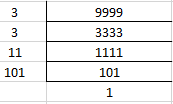Therefore, 9999 is the largest 4-digit number and can be expressed as 3 × 3 × 11 × 101.

5. Find the prime factors of 1729. Arrange the factors in ascending order, and find the relation between two consecutive prime factors.

Solution:

We know that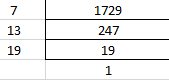Hence, 1729 can be expressed as 7 × 13 × 19

We know that the consecutive prime factors of 1729 are 7, 13 and 19

13 – 7 = 6

19 – 13 = 6

Therefore, in the two consecutive prime factors each factor is 6 more than the previous factor.

6. Which factors are not included in the prime factorization of a composite number?

Solution:

1 and the number itself are not included in the prime factorization of a composite number.

For example, 4 is a composite number having prime factorization 2 × 2.

7. Here are two different factor trees for 60. Write the missing numbers:

(i)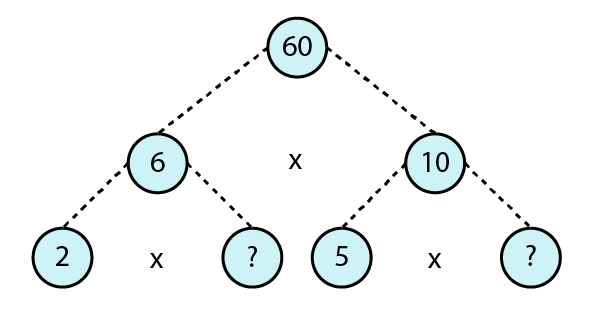(ii)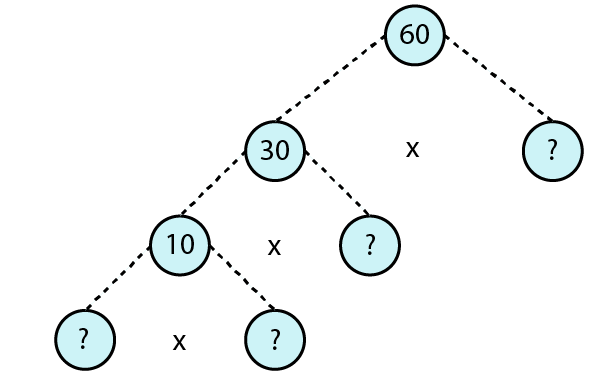Solution:

(i) We know that

6 = 2 × 3

10 = 5 × 2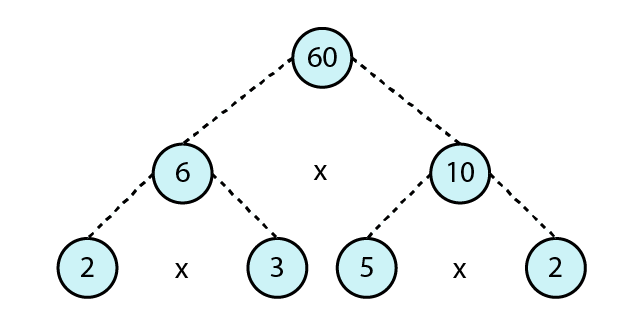(ii) We know that

60 = 30 × 2

30 = 10 × 3

10 = 2 × 5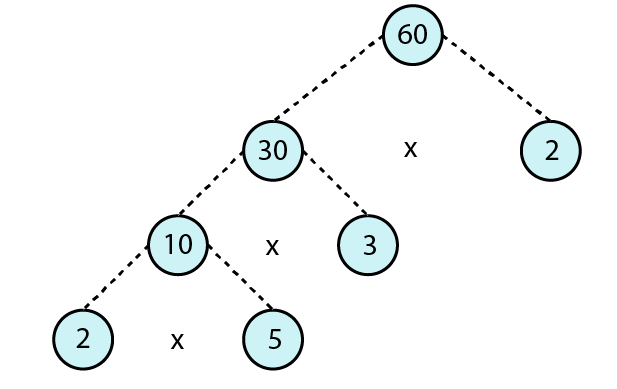### Exercise 2.5 page: 2.20

1. Test the divisibility of the following numbers by 2:

(i) 6520

(ii) 984325

(iii) 367314

Solution:

We know that a natural number is divisible by 2 if 0, 2, 4, 6 or 8 are unit digits.

(i) 6250

The units digit in 6250 is 0

Therefore, 6250 is divisible by 2.

(ii) 984325

The units digit in 984325 is 5

Therefore, 984325 is not divisible by 2.

(iii) 367314

The units digit in 367314 is 4

Therefore, 367314 is divisible by 2.

2. Test the divisibility of the following numbers by 3:

(i) 70335

(ii) 607439

(iii) 9082746

Solution:

We know that a number is divisible by 3 if the sum of digits is divisible by 3.

(i) 70335

We know that

7 + 0 + 3 + 3 + 5 = 18 which is divisible by 3

Therefore, 70335 is divisible by 3.

(ii) 607439

We know that

6 + 0 + 7 + 4 + 3 + 9 = 29 which is not divisible by 3

Therefore, 607439 is not divisible by 3.

(iii) 9082746

We know that

9 + 0 + 8 + 2 + 7 + 4 + 6 = 36 which is divisible by 3

Therefore, 9082746 is divisible by 3.

3. Test the divisibility of the following numbers by 6:

(i) 7020

(ii) 56423

(iii) 732510

Solution:

We know that a number is divisible by 6 if it is divisible by 2 and 3.

(i) 7020

The units digit in 7020 is 0

So 7020 is divisible by 2.

In the same way

7 + 0 + 2 + 0 = 9 which is divisible by 3

Therefore, 7020 is divisible by 6.

(ii) 56423

The units digit in 56423 is 3

So 56423 is not divisible by 2

In the same way

5 + 6 + 4 + 2 + 3 = 20 which is not divisible by 3

Therefore, 56423 is not divisible by 6.

(iii) 732510

The units digit in 732510 is 0

So 732510 is divisible by 2

In the same way

7 + 3 + 2 + 5 + 1 + 0 = 18 which is divisible by 3

Therefore, 732510 is divisible by 6.

4. Test the divisibility of the following numbers by 4:

(i) 786532

(ii) 1020531

(iii) 9801523

Solution:

We know that a natural number is divisible by 4 if the number which is formed by the last two digits is divisible by 4.

(i) 786532

The number which is formed by last two digits is 32 which is divisible by 4

Therefore, 786532 is divisible by 4.

(ii) 1020531

The number which is formed by last two digits is 31 which is not divisible by 4

Therefore, 1020531 is not divisible by 4.

(iii) 9801523

The number which is formed by last two digits is 23 which is not divisible by 4

Therefore, 9801523 is not divisible by 4.

5. Test the divisibility of the following numbers by 8:

(i) 8364

(ii) 7314

(iii) 36712

Solution:

We know that a number is divisible by 8 if the number which is formed by the last three digits is divisible by 8.

(i) 8364

Numbers which is formed by the last three digits is 364 which is not divisible by 8.

Hence, 8364 is not divisible by 8.

(ii) 7314

Numbers which is formed by the last three digits is 314 which is not divisible by 8.

Hence, 7314 is not divisible by 8.

(iii) 36712

Numbers which is formed by the last three digits is 712 which is divisible by 8.

Hence, 36712 is divisible by 8.

6. Test the divisibility of the following numbers by 9:

(i) 187245

(ii) 3478

(iii) 547218

Solution:

We know that a number is divisible by 9 if the sum of digits is divisible by 9.

(i) 187245

Sum of digits = 1 + 8 + 7 + 2 + 4 + 5 = 27 which is divisible by 9.

Hence, 187245 is divisible by 9.

(ii) 3478

Sum of digits = 3 + 4 + 7 + 8 = 22 which is not divisible by 9.

Hence, 3478 is not divisible by 9.

(iii) 547218

Sum of digits = 5 + 4 + 7 + 2 + 1 + 8 = 27 which is divisible by 9.

Hence, 547218 is divisible by 9.

7. Test the divisibility of the following numbers by 11:

(i) 5335

(ii) 70169803

(iii) 10000001

Solution:

(i) 5335

Sum of digits at odd places = 5 + 3 = 8

Sum of digits at even places = 3 + 5 = 8

Difference between them = 8 – 8 = 0

Hence, 5335 is divisible by 11.

(ii) 70169803

Sum of digits at odd places = 7 + 1 + 9 + 0 = 17

Sum of digits at even places = 0 + 6 + 8 + 3 = 17

Difference between them = 17 – 17 = 0

Hence, 70169803 is divisible by 11.

(iii) 10000001

Sum of digits at odd places = 1 + 0 + 0 + 0 = 1

Sum of digits at even places = 0 + 0 + 0 + 1 = 1

Difference between them = 1 – 1 = 0

Hence, 10000001 is divisible by 11.

8. In each of the following numbers, replace * by the smallest number to make it divisible by 3:

(i) 75 * 5

(ii) 35 * 64

(iii) 18 * 71

Solution:

Replacing * by smallest number in order to make it divisible by 3.

(i) 75 * 5

Replacing * by 1 we get

75 * 5 = 7515

By adding the digits we get

7 + 5 + 1 + 5 = 18 which is divisible by 3.

(ii) 35 * 64

Replacing * by 0 we get

35 * 64 = 35064

By adding the digits we get

3 + 5 + 0 + 6 + 4 = 18 which is divisible by 3.

(iii) 18 * 71

Replacing * by 1 we get

18 * 71 = 18171

By adding the digits we get

1 + 8 + 1 + 7 + 1 = 18 which is divisible by 3.

9. In each of the following numbers, replace * by the smallest number to make it divisible by 9:

(i) 67 * 19

(ii) 66784 *

(iii) 538 * 8

Solution:

(i) 67 * 19

Sum of digits = 6 + 7 + 1 + 9 = 23

We know 27 is the multiple of 9 which is greater than 23.

So the smallest required number = 27 – 23 = 4

Hence, the smallest required number is 4.

(ii) 66784 *

Sum of digits = 6 + 6 + 7 + 8 + 4 = 31

We know 36 is the multiple of 9 which is greater than 31.

So the smallest required number = 36 – 31 = 5

Hence, the smallest required number is 5.

(iii) 538 * 8

Sum of digits = 5 + 3 + 8 + 8 = 24

We know that 27 is the multiple of 9 which is greater than 24.

So the smallest required number = 27 – 24 = 3

Hence, the smallest required number is 3.

10. In each of the following numbers, replace * by the smallest number to make it divisible by 11:

(i) 86 * 72

(ii) 467 * 91

(iii) 9 * 8071

Solution:

We know that a number is divisible by 11 if the difference of the sum of alternate digits is 0 or a multiple of 11.

(i) 86 * 72

Sum of digits at odd places = 8 + missing number + 2

= 10 + missing number

Sum of digits at even places = 6 + 7 = 13

Difference between them = 10 + missing number – 13

So the difference = missing number – 3

We know that missing number – 3 = 0 as it is a single digit

Missing number = 3

Therefore, the smallest required number to make it divisible by 11 is 3.

(ii) 467 * 91

Sum of digits at odd places = 4 + 7 + 9 = 20

Sum of digits at even places = 6 + missing number + 1

= 7 + missing number

Difference between them = 20 – (7 + missing number)

So the difference = 13 – missing number

13 – missing number = 11 (Since the missing number is a single digit)

Missing number = 2

Therefore, the smallest required number to make it divisible by 11 is 2.

(iii) 9 * 8071

Sum of digits at odd places = 9 + 8 + 7 = 24

Sum of digits at even places = missing number + 0 + 1

= 1 + missing number

Difference between them = 24 – (1 + missing number)

So the difference = 23 – missing number

23 – missing number = 22 (Since 22 is a multiple of 11 and the missing number is a single digit)

Missing number = 1

Therefore, the smallest required number to make it divisible by 11 is 1.

11. Given an example of a number which is divisible by

(i) 2 but not by 4

(ii) 3 but not by 6

(iii) 4 but not by 8

(iv) both 4 and 8 but not by 32

Solution:

(i) The example of a number which is divisible by 2 but not by 4 is 10.

(ii) The example of a number which is divisible by 3 but not by 6 is 15.

(iii) The example of a number which is divisible by 4 but not by 8 is 28.

(iv) The example of a number which is divisible by both 4 and 8 but not by 32 is 48.

12. Which of the following statements are true?

(i) If a number is divisible by 3, it must be divisible by 9.

(ii) If a number is divisible by 9, it must be divisible by 3.

(iii) If a number is divisible by 4, it must be divisible by 8.

(iv) If a number is divisible by 8, it must be divisible by 4.

(v) A number is divisible by 18, if it is divisible by both 3 and 6.

(vi) If a number is divisible by both 9 and 10, it must be divisible by 90.

(vii) If a number exactly divides the sum of two numbers, it must exactly divide the numbers separately.

(viii) If a number divides three numbers exactly, it must divide their sum exactly.

(ix) If two numbers are co-prime, at least one of them must be a prime number.

(x) The sum of two consecutive odd numbers is always divisible by 4.

Solution:

(i) False. The number 15 is divisible by 3 but not by 9.

(ii) True. The number 18 is divisible by 9 and by 3.

(iii) False. 12 is divisible by 4 but not by 8.

(iv) True. The number 16 is divisible by 8 and by 4.

(v) False. 24 is divisible by both 3 and 6 but not divisible by 18.

(vi) True. 90 is divisible by both 9 and 10.

(vii) False. The number 10 divides the sum of 18 and 2 but 10 does not divide neither 18 nor 2.

(viii) True. 2 divides the number 4, 6 and 8 and the sum 18 exactly.

(ix) False. The numbers 4 and 9 are co-primes which are composite numbers.

(x) True. The sum 3 + 5 = 8 is divisible by 4.

### Exercise 2.6 page: 2.24

1. Find the HCF of the following numbers using prime factorization method:

(i) 144, 198

(ii) 81, 117

(iii) 84, 98

(iv) 225, 450

(v) 170, 238

(vi) 504, 980

(vii) 150, 140, 210

(viii) 84, 120, 138

(ix) 106, 159, 265

Solution:

(i) 144, 198

We know that the prime factorization of 144 = 2 × 2 × 2 × 2 × 3 × 3

The same way prime factorization of 198 = 2 × 3 × 3 × 11

Hence, HCF of 144, 198 is 2 × 3 × 3 = 18

(ii) 81, 117

We know that prime factorization of 81 = 3 × 3 × 3 × 3

The same way prime factorization of 117 = 3 × 3 × 13

Hence, HCF of 81, 117 = 3 × 3 = 9

(iii) 84, 98

We know that prime factorization of 84 = 2 × 2 × 3 × 7

The same way prime factorization of 98 = 2 × 7 × 7

Hence, HCF of 84, 98 = 2 × 7 = 14

(iv) 225, 450

We know that prime factorization of 225 = 3 × 3 × 5 × 5

The same way prime factorization of 450 = 2 × 3 × 3 × 5 × 5

Hence, HCF of 225, 450 = 3 × 3 × 5 × 5 = 225

(v) 170, 238

We know that prime factorization of 170 = 2 × 5 × 17

The same way prime factorization of 238 = 2 × 7 × 17

Hence, HCF of 170, 238 = 2 × 17 = 34

(vi) 504, 980

We know that the prime factorization of 504 = 2 × 2 × 2 × 3 × 3 × 7

The same way prime factorization of 980 = 2 × 2 × 5 × 7 × 7

Hence, HCF of 504, 980 = 2 × 2 × 7 = 28

(vii) 150, 140, 210

We know that prime factorization of 150 = 2 × 3 × 5 × 5

The same way prime factorization of 140 = 2 × 2 × 5 × 7

Prime factorization of 210 = 2 × 3 × 5 × 7

Hence, HCF of 150, 140, 210 = 2 × 5 = 10

(viii) 84, 120, 138

We know that prime factorization of 84 = 2 × 2 × 3 × 7

The same way prime factorization of 120 = 2 × 2 × 2 × 3 × 5

Prime factorization of 138 = 2 × 3 × 23

Hence, HCF of 84, 120, 138 = 2 × 3 = 6

(ix) 106, 159, 265

We know that prime factorization of 106 = 2 × 53

The same way prime factorization of 159 = 3 × 53

Prime factorization of 265 = 5 × 53

Hence, HCF of 106, 159, 265 = 53

2. What is the HCF of two consecutive

(i) Numbers

(ii) even numbers

(iii) odd numbers

Solution:

(i) We know that the common factor of two consecutive numbers is 1.

Hence, HCF of two consecutive numbers is 1.

(ii) We know that the common factors of two consecutive even numbers are 1 and 2.

Hence, HCF of two consecutive even numbers is 2.

(iii) We know that the common factors of two consecutive odd numbers is 1.

Hence, HCF of two consecutive odd numbers is 1.

3. HCF of co-prime numbers 4 and 15 was found as follows:

4 = 2 × 2 and 15 = 3 × 5

Since there is no common prime factor. So, HCF of 4 and 15 is 0. Is the answer correct? If not, what is the correct HCF?

Solution:

No. It is not correct.

The HCF of two co-prime numbers is 1.

We know that 4 and 15 are co-prime numbers having common factor 1.

Therefore, HCF of 4 and 15 is 1.

### Exercise 2.7 page: 2.27

1. Determine the HCF of the following numbers by using Euclid’s algorithm (i – x):

(i) 300, 450

(ii) 399, 437

(iii) 1045, 1520

Solution:

(i) 300, 450

Taking 450 as dividend and 300 as divisor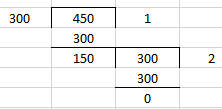We know that the last divisor is 150

Therefore, HCF of 300, 450 is 150.

(ii) 399, 437

Taking 437 as dividend and 399 as divisor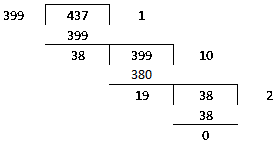We know that the last divisor is 19

Therefore, HCF of 399, 437 is 19.

(iii) 1045, 1520

Taking 1520 as dividend and 1045 as divisor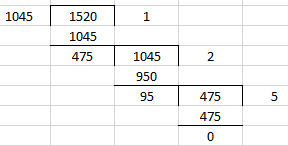We know that the last divisor is 95

Therefore, HCF of 1045, 1520 is 95.

2. Show that the following pairs are co-prime:

(i) 59, 97

(ii) 875, 1859

(iii) 288, 1375

Solution:

(i) 59, 97

Taking 97 as dividend and 59 as divisor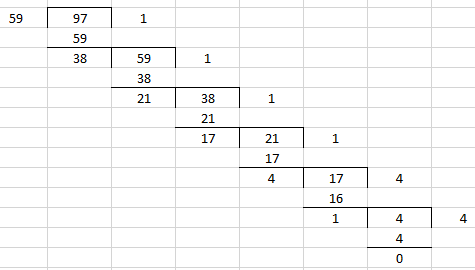We know that the last divisor is 1.

Therefore, the numbers 59, 97 are co-prime.

(ii) 875, 1859

Taking 1859 as dividend and 875 as divisor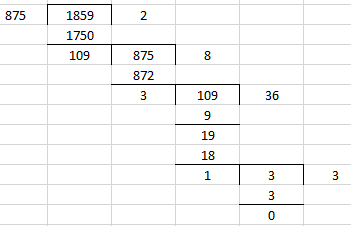We know that the last divisor is 1.

Therefore, the numbers 875, 1859 are co-prime.

(iii) 288, 1375

Taking 1375 as dividend and 288 as divisor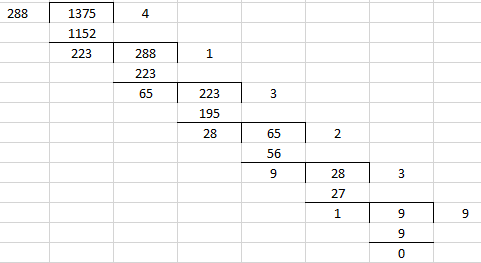We know that the last divisor is 1.

Therefore, the numbers 288, 1375 are co-prime.

3. What is the HCF of two consecutive numbers?

Solution:

We know that the HCF of two consecutive numbers is 1.

For example consider 4 and 5 as two consecutive numbers

Taking 5 as dividend and 4 as divisor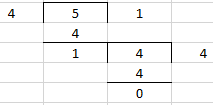We know that the last divisor is 1.

Therefore, HCF of 4 and 5 is 1.

4. Write true (T) or false (F) for each of the following statements:

(i) The HCF of two distinct prime numbers is 1.

(ii) The HCF of two co-prime number is 1.

(iii) The HCF of an even and an odd number is 1.

(iv) The HCF of two consecutive even numbers is 2.

(v) The HCF of two consecutive odd numbers is 2.

Solution:

(i) True.

(ii) True.

(iii) False. The HCF of even number 6 and odd number 9 is 3.

(iv) True.

(v) False. The HCF of numbers 25 and 27 is 1.

### Exercise 2.8 PAGE: 2.31

1. Find the largest number which divides 615 and 963 leaving remainder 6 in each case.

Solution:

In order to find the largest number which divides 615 and 963 leaving remainder 6

We get

615 – 6 = 609

963 – 6 = 957

So the required number = HCF of 609 and 957

By resolving the required number into prime factors we get

609 = 3 × 7 × 29

957 = 3 × 11 × 29

So the HCF of 609 and 957 = 29 × 3 = 87

Therefore, the largest number which divides 615 and 963 leaving remainder 6 in each case is 87.

2. Find the greatest number which divides 285 and 1249 leaving remainders 9 and 7 respectively.

Solution:

In order to find the greatest number which divides 285 and 1249 leaving remainders 9 and 7

We get

285 – 9 = 276

1249 – 7 = 1242

So the required number = HCF of 276 and 1242

By resolving the required number into prime factors we get

276 = 2 × 2 × 3 × 23

1242 = 2 × 3 × 3 × 3 × 23

So the HCF of 276 and 1242 = 2 × 3 × 23 = 138

Therefore, the greatest number which divides 285 and 1249 leaving remainders 9 and 7 is 138.

3. What is the largest number that divides 626, 3127 and 15628 and leaves remainders of 1, 2 and 3 respectively?

Solution:

In order to find the largest number which divides 626, 3127 and 15628 leaving remainders 1, 2 and 3

We get

626 – 1 = 625

3127 – 2 = 3125

15628 – 3 = 15625

So the required number = HCF of 625, 3125 and 15625

By resolving the required number into prime factors we get

625 = 5 × 5 × 5 × 5

3125 = 5 × 5 × 5 × 5 × 5

15625 = 5 × 5 × 5 × 5 × 5 × 5

So the HCF of 625, 3125 and 15625 = 5 × 5 × 5 × 5 = 625

Therefore, the largest number that divides 626, 3127 and 15628 and leaves remainders 1, 2 and 3 is 625.

4. The length, breadth and height of a room are 8m 25cm, 6m 75cm and 4m 50cm, respectively. Determine the longest rod which can measure the three dimensions of the room exactly.

Solution:

The dimensions of room are

Length = 8m 25cm = 825cm

Breadth = 6m 75cm = 675cm

Height = 4m 50cm = 450cm

So the longest rod = HCF of 825, 675 and 450

We know that the prime factorization of 825 = 3 × 5 × 5 × 11

The same way prime factorization of 675 = 3 × 3 × 3 × 5 × 5

Prime factorization of 450 = 2 × 3 × 3 × 5 × 5

So the HCF of 825, 675 and 450 = 3 × 5 × 5 = 75

Therefore, the longest rod which can measure the dimensions of the room exactly is 75cm.

5. A rectangular courtyard is 20m 16cm long and 15m 60cm broad. It is to be paved with square stones of the same size. Find the least possible number of such stones.

Solution:

The dimensions of courtyard are

Length = 20m 16cm = 2016cm

Breadth = 15m 60cm = 1560cm

Least possible side of square stones used = HCF of 2016 and 1560

We know that the prime factorization of

2016 = 2 × 2 × 2 × 2 × 2 × 3 × 3 × 7

1560 = 2 × 2 × 2 × 3 × 5 × 13

So HCF of 2016 and 1560 = 2 × 2 × 2 × 3 = 24

Hence, the least possible side of square stones used is 24cm.

We know that

No. of square stones which is used to pave the rectangular courtyard = Area of courtyard/ Area of stone

By substituting the values

No. of square stones which is used to pave the rectangular courtyard = (2016 × 1560)/ (24)2

= 84 × 65

= 5460

Therefore, the least possible number of such stones is 5460.

6. Determine the longest tape which can be used to measure exactly the lengths 7m, 3m 85cm and 12m 95cm.

Solution:

The lengths of tapes are

First = 7m = 700cm

Second = 3m 85cm = 385cm

Third = 12m 95cm = 1295cm

So the length of longest tape = HCF of 700, 385 and 1295

Prime factorizations are

700 = 2 × 2 × 5 × 5 × 7

385 = 5 × 7 × 11

1295 = 5 × 7 × 37

We get HCF of 700, 385 and 1295 = 5 × 7 = 35

Therefore, the longest tape which can be used is of 35cm length.

7. 105 goats, 140 donkeys and 175 cows have to be taken across a river. There is only one boat which will have to make many trips in order to do so. The lazy boatman has his own conditions for transporting them. He insists that he will take the same number of animals in every trip and they have to be of the same kind. He will naturally like to take the largest possible number each time. Can you tell how many animals went in each trip?

Solution:

In order to find the largest possible number of animals = HCF of 105, 140 and 175

So prime factorization is

105 = 3 × 5 × 7

140 = 2 × 2 × 5 × 7

175 = 5 × 5 × 7

We get HCF of 105, 140 and 175 = 5 × 7 = 35

Therefore, 35 animals went in each trip.

8. Two brands of chocolates are available in packs of 24 and 15 respectively. If I need to buy an equal number of chocolates of both kinds, what is the least number of boxes of each kind I would need to buy?

Solution:

Consider brand A contain 24 chocolates and brand B contain 15 chocolates

We know that equal number of chocolates can be found by taking LCM of the number of chocolates

So we get

LCM of 15 and 24 is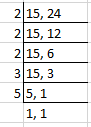So the required LCM = 2 × 2 × 2 × 3 × 5 = 120

Hence, 120 chocolates of each kind should be purchased.

No. of boxes of brand A that should be purchased = 120 ÷ 24 = 5

No. of boxes of brand B that should be purchased = 120 ÷ 15 = 8

Therefore, the least number of boxes of each kind to be purchased is 5 and 8.

9. During a sale, colour pencils were being sold in packs of 24 each and crayons in packs of 32 each. If you want full packs of both and the same number of pencils and crayons, how many of each would you need to buy?

Solution:

In order to find the required number of pencils and crayons we need to determine the LCM of 24 and 32

Prime factorizations are

24 = 2 × 2 × 2 × 3

32 = 2 × 2 × 2 × 2 × 2

So the required LCM = 2 × 2 × 2 × 2 × 2 × 3 = 96

We know that

No. of pencils and crayons which should be bought is 96 each

So we get

96 ÷ 24 = 4 packs of colour pencils

96 ÷ 32 = 3 packs of crayons.

Therefore, the person should buy 4 packs of colour pencils and 3 packs of crayons.

10. Reduce each of the following fractions to the lowest terms:

(i) 161/ 207

(ii) 296/ 481

Solution:

(i) 161/ 207

In order to reduce the fraction to lowest terms, we have to divide both numerator and denominator by their HCF

So we must find HCF of 161 and 207

We know that prime factorization of

161 = 7 × 23

207 = 3 × 3 × 23

So we get HCF of 161 and 207 = 23

It can be written as

(161 + 23)/ (207 + 23) = 7/9

Therefore, the required fraction is 7/9.

(ii) 296/ 481

In order to reduce the fraction to lowest terms, we have to divide both numerator and denominator by their HCF

So we must find HCF of 296 and 481

We know that prime factorization of

296 = 2 × 2 × 2 × 37

481 = 13 × 37

So we get HCF of 296 and 481 = 37

It can be written as

(296 + 37)/ (481 + 37) = 8/ 13

Therefore, the required fraction is 8/13.

11. A merchant has 120 litres of oil of one kind, 180 litres of another kind and 240 litres of third kind. He wants to sell the oil by filling the three kinds of oil in tins of equal capacity. What should be the greatest capacity of such a tin?

Solution:

Prime factorization of

120 = 2 × 2 × 2 × 3 × 5

180 = 2 × 2 × 3 × 3 × 5

240 = 2 × 2 × 2 × 2 × 3 × 5

So HCF of 120, 180, 240 = 2 × 2 × 3 × 5 = 60

Therefore, the greatest capacity of such a tin is 60 litres.

### Exercise 2.9 page: 2.34

1. Determine the LCM of the numbers given below:

(i) 48, 60

(ii) 42, 63

(iii) 18, 17

(iv) 15, 30, 90

(v) 56, 65, 85

(vi) 180, 384, 144

(vii) 108, 135, 162

(viii) 28, 36, 45, 60

Solution:

(i) 48, 60

We know that prime factorization of

48 = 2 × 2 × 2 × 2 × 3

60 = 2 × 2 × 3 × 5

Hence, the required LCM = 2 × 2 × 2 × 2 × 3 × 5 = 240

(ii) 42, 63

We know that prime factorization of

42 = 2 × 3 × 7

63 = 3 × 3 × 7

Hence, the required LCM = 2 × 3 × 3 × 7 = 126

(iii) 18, 17

We know that prime factorization of

18 = 2 × 3 × 3

17 = 17

Hence, the required LCM = 2 × 3 × 3 × 17 = 306

(iv) 15, 30, 90

We know that prime factorization of

15 = 3 × 5

30 = 2 × 3 × 5

90 = 2 × 3 × 3 × 5

Hence, the required LCM = 2 × 3 × 3 × 5 = 90

(v) 56, 65, 85

We know that prime factorization of

56 = 2 × 2 × 2 × 7

65 = 5 × 13

85 = 5 × 17

Hence, the required LCM = 2 × 2 × 2 × 5 × 7 × 13 × 17 = 61880

(vi) 180, 384, 144

We know that prime factorization of

180 = 2 × 2 × 3 × 3 × 5

384 = 2 × 2 × 2 × 2 × 2 × 2 × 2 × 3

144 = 2 × 2 × 2 × 2 × 3 × 3

Hence, the required LCM = 2 × 2 × 2 × 2 × 2 × 2 × 2 × 3 × 3 × 5 = 5760

(vii) 108, 135, 162

We know that prime factorization of

108 = 2 × 2 × 3 × 3 × 3

135 = 3 × 3 × 3 × 5

162 = 2 × 3 × 3 × 3 × 3

Hence, the required LCM = 2 × 2 × 3 × 3 × 3 × 3 × 5 = 1620

(viii) 28, 36, 45, 60

We know that prime factorization of

28 = 2 × 2 × 7

36 = 2 × 2 × 3 × 3

45 = 3 × 3 × 5

60 = 2 × 2 × 3 × 5

Hence, the required LCM = 2 × 2 × 3 × 3 × 5 × 7 = 1260

### Exercise 2.10 page: 2.37

1. What is the smallest number which when divided by 24, 36 and 54 gives a remainder of 5 each time?

Solution:

Prime factorization of

24 = 2 × 2 × 2 × 3

36 = 2 × 2 × 3 × 3

54 = 2 × 3 × 3 × 3

So the required LCM = 2 × 2 × 2 × 3 × 3 × 3 = 216

The smallest number which is exactly divisible by 24, 36 and 54 is 216

In order to get remainder as 5

Required smallest number = 216 + 5 = 221

Therefore, the smallest number which when divided by 24, 36 and 54 gives a remainder of 5 each time is 221.

2. What is the smallest number that both 33 and 39 divide leaving remainder of 5?

Solution:

Prime factorization of

33 = 3 × 11

39 = 3 × 13

So the required LCM = 3 × 11 × 13 = 429

The smallest number which is exactly divisible by 33 and 39 is 429

In order to get remainder as 5

Required smallest number = 429 + 5 = 434

Therefore, the smallest number that both 33 and 39 divide leaving remainder of 5 is 434.

3. Find the least number that is divisible by all the numbers between 1 and 10 (both inclusive).

Solution:

The LCM of numbers from 1 to 10 need to be found out to find the least number

We know that 2, 3, 5 and 7 are prime numbers

Prime factorization of

4 = 2 × 2

6 = 2 × 3

8 = 2 × 2 × 2

9 = 3 × 3

10 = 2 × 5

So we get required least number = 2 × 2 × 2 × 3 × 3 × 5 × 7 = 2520

Therefore, the least number that is divisible by all the numbers between 1 and 10 is 2520.

4. What is the smallest number that, when divided by 35, 56 and 91 leaves remainder of 7 in each case?

Solution:

Prime factorization of

35 = 5 × 7

56 = 2 × 2 × 2 × 7

91 = 7 × 13

So the required LCM = 2 × 2 × 2 × 5 × 7 × 13 = 3640

The smallest number which is exactly divisible by 35, 56 and 91 is 364

In order to get the remainder as 7

Required smallest number = 3640 + 7 = 3647

Therefore, 3647 is the smallest number that when divided by 35, 56 and 91 leaves remainder of 7 in each case.

5. In a school there are two sections – section A and section B of Class VI. There are 32 students in section A and 36 in section B. Determine the minimum number of books required for their class library so that they can be distributed equally among students of section A or section B.

Solution:

Prime factorization of

32 = 2 × 2 × 2 × 2 × 2

36 = 2 × 2 × 3 × 3

So the required LCM = 2 × 2 × 2 × 2 × 2 × 3 × 3 = 288

Hence, the minimum number of books required for their class library = LCM of 32 and 36 = 288 books

6. In a morning walk three persons step off together. Their steps measure 80cm, 85cm and 90cm respectively. What is the minimum distance each should walk so that he can cover the distance in complete steps?

Solution:

Prime factorization of

80 = 2 × 2 × 2 × 2 × 5

85 = 5 × 17

90 = 2 × 3 × 3 × 5

So the required LCM = 2 × 2 × 2 × 2 × 3 × 3 × 5 × 17 = 12240

Hence, the required minimum distance each should walk = LCM of 80cm, 85cm and 90cm = 12240 cm = 122m 40cm.

7. Determine the number nearest to 100000 but greater than 100000 which is exactly divisible by each of 8, 15 and 21.

Solution:

In order to determine the number we must find LCM of 8, 15 and 21

Prime factorization of

8 = 2 × 2 × 2

15 = 3 × 5

21 = 3 × 7

So the required LCM = 2 × 2 × 2 × 3 × 5 × 7 = 840

840 is the number nearest to 100000 and divisible by 8, 15 and 21 and by LCM

Taking 100000 as dividend and 840 as divisor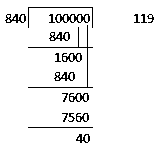The remainder obtained is 40

So we get the number greater than 100000 and exactly divisible by 840 = 100000 + (840 – 40) = 100000 + 800 = 100800.

Hence, the required number is 100800.

8. A school bus picking up children in a colony of flats stops at every sixth block of flats. Another school bus starting from the same place stops at every eighth blocks of flats. Which is the first bus stop at which both of them will stop?

Solution:

We know that

The first bus stop at which both of them will stop = LCM of 6th and 8th block

Prime factorization of

6 = 2 × 3

8 = 2 × 2 × 2

So the required LCM = 2 × 2 × 2 × 3 = 24

Therefore, 24th block is the first bus stop at which both of them will stop.

9. Telegraph poles occur at equal distances of 220m along a road and heaps of stones are put at equal distances of 300m along the same road. The first heap is at the foot of the first pole. How far from it along the road is the next heap which lies at the foot of a pole?

Solution:

Prime factorization of

220 = 2 × 2 × 5 × 11

300 = 2 × 2 × 3 × 5 × 5

So the required LCM = 2 × 2 × 3 × 5 × 5 × 11 = 3300

Therefore, the next heap which lies at the foot of a pole is 3300m far along the road.

10. Find the smallest number which leaves remainders 8 and 12 when divided by 28 and 32 respectively.

Solution:

Prime factorization of

28 = 2 × 2 × 7

32 = 2 × 2 × 2 × 2 × 2

So the required LCM = 2 × 2 × 2 × 2 × 2 × 7 = 224

The number leaves remainders 8 and 12 when divided by 28 and 32

So we get

28 – 8 = 20 and 32 – 12 = 20

Hence, the required number is 224 – 20 = 204

### Exercise 2.11 PAGE: 2.40

1. For each of the following pairs of numbers, verify the property:

Product of the number = Product of their HCF and LCM

(i) 25, 65

(ii) 117, 221

(iii) 35, 40

(iv) 87, 145

(v) 490, 1155

Solution:

(i) 25, 65

Prime factorization of

25 = 5 × 5

65 = 5 × 13

So we get

HCF of 25, 65 = 5

LCM of 25 ,65 = 5 × 5 × 13 = 325

So we get the product of numbers = 25 × 65 = 1625

Product of HCF and LCM = 5 × 325 = 1625

Hence, it is verified that product of the number = product of their HCF and LCM.

(ii) 117, 221

Prime factorization of

117 = 3 × 3 × 13

221 = 13 × 17

So we get

HCF of 117, 221 = 13

LCM of 117, 221 = 3 × 3 × 13 × 17 = 1989

So we get the product of numbers = 117 × 221 = 25857

Product of HCF and LCM = 13 × 1989 = 25857

Hence, it is verified that product of the number = product of their HCF and LCM.

(iii) 35, 40

Prime factorization of

35 = 5 × 7

40 = 2 × 2 × 2 × 5

So we get

HCF of 35, 40 = 5

LCM of 35, 40 = 2 × 2 × 2 × 5 × 7 = 280

So we get the product of numbers = 35 × 40 = 1400

Product of HCF and LCM = 5 × 280 = 1400

Hence, it is verified that product of the number = product of their HCF and LCM.

(iv) 87, 145

Prime factorization of

87 = 3 × 29

145 = 5 × 29

So we get

HCF of 87, 145 = 29

LCM of 87, 145 = 3 × 5 × 29 = 435

So we get the product of numbers = 87 × 145 = 12615

Product of HCF and LCM = 29 × 435 = 12615

Hence, it is verified that product of the number = product of their HCF and LCM.

(v) 490, 1155

Prime factorization of

490 = 2 × 5 × 7 × 7

1155 = 3 × 5 × 7 × 11

So we get

HCF of 490, 1155 = 35

LCM of 490, 1155 = 2 × 3 × 3 × 5 × 7 × 7 × 11 = 16170

So we get the product of numbers = 490 × 1155 = 565950

Product of HCF and LCM = 35 × 16170 = 565950

Hence, it is verified that product of the number = product of their HCF and LCM.

2. Find the HCF and LCM of the following pairs of numbers:

(i) 117, 221

(ii) 234, 572

(iii) 145, 232

(iv) 861, 1353

Solution:

(i) 117, 221

Prime factorization of

117 = 3 × 3 × 13

221 = 13 × 17

So the required HCF = 13

Required LCM = 3 × 3 × 13 × 17 = 1989

(ii) 234, 572

Prime factorization of

234 = 2 × 3 × 3 × 13

572 = 2 × 2 × 11 × 13

So the required HCF = 26

Required LCM = 2 × 2 × 3 × 3 × 11 × 13 = 5148

(iii) 145, 232

Prime factorization of

145 = 5 × 29

232 = 2 × 2 × 2 × 29

So the required HCF = 29

Required LCM = 2 × 2 × 2 × 5 × 29 = 1160

(iv) 861, 1353

Prime factorization of

861 = 3 × 7 × 41

1353 = 3 × 11 × 41

So the required HCF = 123

Required LCM = 3 × 7 × 11 × 41 = 9471

3. The LCM and HCF of two numbers are 180 and 6 respectively. If one of the numbers is 30, find the other number.

Solution:

It is given that

LCM of two numbers = 180

HCF of two numbers = 6

One of the number = 30

We know that

Product of two numbers = Product of HCF and LCM

So we get

30 × other number = 6 × 180

On further calculation

Other number = (6 × 180)/ 30 = 36

Hence, the other number is 36.

4. The HCF of two numbers is 16 and their product is 3072. Find their LCM.

Solution:

It is given that

HCF of two numbers = 16

Product = 3072

We know that

Product of two numbers = Product of HCF and LCM

So we get

3072 = 16 × LCM

On further calculation

LCM = 3072/ 16 = 192

Therefore, the LCM of two numbers is 192.

5. The HCF of two numbers is 145, their LCM is 2175. If one number is 725, find the other.

Solution:

It is given that

HCF of two numbers = 145

LCM = 2175

We know that

Product of two numbers = Product of HCF and LCM

So we get

725 × other number = 145 × 2175

On further calculation

Other number = (145 × 2175)/ 725 = 435

Therefore, the other number is 435.

6. Can two numbers have 16 as their HCF and 380 as their LCM? Give reason.

Solution:

No. HCF of two numbers must exactly divide their LCM

We know that 16 does not divide 380 exactly

Therefore, no two numbers can exist with 16 as HCF and 380 as LCM.

### Objective Type Questions page: 2.40

Mark the correct alternative in each of the following:

1. Which of the following numbers is a perfect number?
(a) 4
(b) 12
(c) 8
(d) 6

Solution:

The option (d) is correct answer.

We know that the factors of 6 are 1, 2, 3, and 6.

So the sum of the factors of 6 = 1 + 2 + 3 + 6 = 12 = 2 × 6

2. Which of the following are not twin-primes?
(a) 3, 5
(b) 5, 7
(c) 11, 13
(d) 17, 23

Solution:

The option (d) is correct answer.

We know that the difference between 17 and 23 = 23 – 17 = 6

Therefore, 17 and 23 are not twin-primes.

3. Which of the following are co-primes?
(a) 8,10
(b) 9,10
(c) 6,8
(d) 15,18

Solution:

The option (b) is correct answer.

We know that 9 = 3 ×3 × 1 and 10 = 2 × 5 × 1

Both 9 and 10 are composite numbers with common factor 1

Hence, 9 and 10 are co-primes

4. Which of the following is a prime number?
(a) 263
(b) 361
(c) 323
(d) 324

Solution:

The option (a) is correct answer.

We know that 263 = 1 × 263

263 has two factors, 1 and 263

Therefore, it is a prime number

5. The number of primes between 90 and 100 is
(a) 0
(b) 1
(c) 2
(d) 3

Solution:

The option (b) is correct answer.

The prime number which lies between 90 and 100 is 97.

6. Which of the following numbers is a perfect number?
(a) 16
(b) 8
(c) 24
(d) 28

Solution:

The option (d) is correct answer.

We know that the factors of 28 are 1, 2, 4, 7, 14, and 28

So the sum of factors of 28 = 1 + 2 + 4 + 7 + 14 + 28 = 56 = 2 × 28

Therefore, 28 is a perfect number.

7. Which of the following is a prime number?
(a) 203
(b) 139
(c) 115
(d) 161

Solution:

The option (b) is correct answer.

We know that 139 = 1 × 139

139 has two factors, 1 and 139

Therefore, it is a prime number.

8. The total number of even prime numbers is
(a) 0
(b) 1
(c) 2
(d) unlimited

Solution:

The option (c) is correct answer.

The only even prime number is 2.

9. Which one of the following is a prime number?
(a) 161
(b) 221
(c) 373
(d) 437

Solution:

The option (c) is correct answer.

We know that 373 = 1 × 373

373 has two factors, 1 and 373

Thus, it is a prime number.

10. The least prime is
(a) 1
(b) 2
(c) 3
(d) 5

Solution:

The option (b) is correct answer.

The least prime number is 2.

11. Which one of the following numbers is divisible by 3?
(a) 27326
(b) 42356
(c) 73545
(d) 45326

Solution:

The option (c) is correct answer.

We know that the sum of the digits in 73,545 = 7 + 3 + 5 + 4 + 5 = 24

24 is divisible by 3 and 73545 is divisible by 3.

12. Which of the following numbers is divisible by 4?
(a) 8675231
(b) 9843212
(c) 1234567
(d) 543123

Solution:

The option (b) is correct answer.

Number that is formed by the last two digits is 12, which is divisible by 4

Thus, 98,43,212 is divisible by 4.

13. Which of the following numbers is divisible by 6?
(a) 7908432
(b) 68719402
(c) 45982014
(d)

Solution:

The option (a) is correct answer.

Consider 79,08,432

We know that the sum of the digits = 7 + 9 + 0 + 8 + 4 + 3 + 2 = 33

33 is a multiple of 3 and is divisible by 3.

Last digit is 2 and is divisible by 2.

Hence, 79,08,432 is divisible by 6.

14. Which of the following numbers is divisible by 8?
(a) 87653234
(b) 78956042
(c) 64298602
(d) 98741032

Solution:

The option (d) is correct answer.

The number formed by last three digits in 98741032 i.e. 032 is divisible by 8.

15. Which of the following numbers is divisible by 9?
(a) 9076185
(b) 92106345
(c) 10349576
(d) 95103476

Solution:

The option (a) is correct answer.

Consider 90, 76,185

We know that the sum of the digits = 9 + 0 + 7 + 6 + 1 + 8 + 5 = 36

Thus, 36 is divisible by 9 and 9076185 is divisible by 9.

16. Which of the following numbers is divisible by 11?
(a) 1111111
(b) 22222222
(c) 3333333
(d) 4444444

Solution:

The option (b) is correct answer.

Consider 2,22,22,222,

We know that the difference of the sum of alternate digits 2 + 2 + 2 + 2 = 8 and  2 + 2 + 2 +2 = 8 is 0.

Therefore, the number is divisible by 11.

17. If 1*548 is divisible by 3, then * can take the value
(a) 0
(b) 2
(c) 7
(d) 8

Solution:

The option (a) is correct answer.

We know that the sum of the given digits = 1 + 5 + 4 + 8 = 18

Hence 18 is a multiple of 3 and the required digit is 0.

18. 5*2 is a three digit number with * as a missing digit. If the number is divisible by 6, the missing digit is.
(a) 2
(b) 3
(c) 6
(d) 7

Solution:

The option (a) is correct answer.

We know that the sum of the given digits = 5 + 2 = 7

Multiple of 3 greater than 7 is 9.

So we get 9 − 7 = 2

Hence, the required digit is 2.

19. What least value should be given to * so that the number 6342*1 is divisible by 3?
(a) 0
(b) 1
(c) 2
(d) 3

Solution:

The option (c) is correct answer.

We know that the sum of the given digits = 6 + 3 + 4 + 2 + 1 = 16

Multiple of 3 greater than 16 is 18.

Difference between them = 18 − 16 = 2

Hence, the smallest required digit is 2.

20. What least value should be given to * so that the number 915*26 is divisible by 9?
(a) 1
(b) 4
(c) 2
(d) 6

Solution:

The option (b) is correct answer.

We know that the sum of the given digits = 9 + 1 + 5 + 2 + 6 = 23

Multiple of 9 greater than 23 is 27

Difference between them = 27 − 23 = 4

Therefore, the smallest required digit is 4.

21. What least number be assigned to * so that number 653*47 is divisible by 11?
(a) 1
(b) 2
(c) 6
(d) 9

Solution:

The option (a) is correct answer.

We know that the sum of the digits at odd places = 6 + 3 + 4 = 13

In the same way the sum of the digits at even places = 5 + * + 7 = 12 + *

Difference between them = 13 − [12 + *] = 1 + *

So 6, 53,*47 is divisible by 11, then 1 + * must be zero or multiple of 11

It can be written as

1 + * = 0 or 11 and * = 1 or 10

We know that * is a digit, so it must be 1.

22. What least number be assigned to * so that the number 63576*2 is divisible by 8?
(a) 1
(b) 2
(c) 3
(d) 4

Solution:

The option (c) is correct answer.

We know that a number is divisible by 8 if the number formed by its last three digits is divisible by 8.

Hence, 63, 57, 6*2 is divisible by 8 if 6*2 is divisible by 8.

Therefore, the least value of * will be 3.

23. Which one of the following numbers is exactly divisible by 11?
(a) 235641
(b) 245642
(c) 315624
(d) 415624

Solution:

The option (d) is correct answer.

We know that the sum of digits at odd places = 4 + 5 + 2 = 11

The same way the sum of digits at even places = 1 + 6 + 4 = 11

So the difference between them = 11 − 11 = 0

Hence, 4, 15,624 is divisible by 11.

24. If 1*548 is divisible by 3, which of the following digits can replace *?
(a) 0
(b) 2
(c) 7
(d) 9

Solution:

The option (a) is correct answer.

We know that the sum of the given digits = 1 + 5 + 4 + 8 = 18

18 is a multiple of 3 then the required digit is 0.

25. The sum of the prime numbers between 60 and 75 is
(a) 199
(b) 201
(c) 211
(d) 272

Solution:

The option (d) is correct answer.

We know that the prime numbers between 60 and 75 are 61, 67, 71, and 73.

So their sum is

61 + 67 + 71 + 73 = 272

26. The HCF of two consecutive natural numbers is
(a) 0
(b) 1
(c) 2
(d) non-existant

Solution:

The option (b) is correct answer.

We know that the HCF of any two consecutive natural numbers is 1 as two consecutive natural numbers are always co-prime.

27. The HCF of two consecutive even numbers is
(a) 1
(b) 2
(c) 0
(d) non-existant

Solution:

The option (b) is correct answer.

We know that the HCF of two consecutive even numbers is always 2.

Example: HCF of 4 and 6 is 2 and HCF of 10 and 12 is 2.

28. The HCF of two consecutive odd numbers is
(a) 1
(b) 2
(c) 0
(d) non-existant

Solution:

The option (a) is correct answer.

The common factor of two consecutive odd numbers is 1.

Hence, HCF of two consecutive odd numbers is 1.

29. The HCF of an even number and an odd number is
(a) 1
(b) 2
(c) 0
(d) non-existant

Solution:

The option (a) is correct answer.

Some of the examples are:

HCF of 6 and 9 is 3.

HCF of 8 and 21 is 1.

HCF of 9 and 36 is 9.

There is no fixed number which can be HCF and LCM.

30. The LCM of 24, 36 and 40 is
(a) 4
(b) 90
(c) 360
(d) 720

Solution:

The option (c) is correct answer.

We know that

The given numbers can be written as

24 = 2 × 2 × 2 × 3 = 23 × 3

36 = 2 × 2 × 3 × 3 = 22 × 32

40 = 2 × 2 × 2 × 5 = 23 × 5

We get 2, 3, and 5 as the prime factors having highest powers 3, 2, and 1.

So we get LCM of 24, 36, and 40 = 23 × 32 × 5 = 8 × 9 × 5 = 360

31. If x and y are two co-primes, then their LCM is
(a) xy
(b) x + y
(c) x/y
(d) 1

Solution:

The option (a) is correct answer.

We know that LCM of two co-prime numbers is equal to their product.

Hence, LCM of ‘x’ and ‘y’ will be xy.

32. If the HCF of two number is 16 and their product is 3072, then their LCM is
(a) 182
(b) 192
(c) 12
(d) None of these

Solution:

The option (b) is correct answer.

We know:

Product of HCF and LCM = Product of two numbers

By substituting the values

16 × LCM = 3,072

So we get LCM = 3,072/16=192

33. The least number divisible by 15,20,24,32 and 36 is
(a) 1440
(b) 1660
(c) 2880
(d) None of these

Solution:

The option (a) is correct answer.

We can find the least number which is divisible by 15, 20, 24, 32 and 36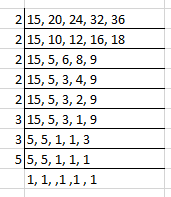We know that LCM of 15, 20, 24, 32 and 36 = 2 × 2 × 2 × 2 × 2 × 3 × 3 × 5 = 1,440

Therefore, 1,440 is the least number that is divisible by 15, 20, 24, 32 and 36.

34. The smallest number which when diminished by 3 is divisible by 11, 28, 36 and 45 is
(a) 1257
(b) 1260
(c) 1263
(d) None of these

Solution:

The option (d) is correct answer.

So the required smallest number = LCM of (11, 28, 36, 45) + 3

We get the required smallest number = 13,860 + 3 = 13,863

35. Three numbers are in the ratio 1:2:3 and their HCF is 6, the numbers are
(a) 4,8,12
(b) 5,10,15
(c) 6,12,18
(d) 10,20,30

Solution:

The option (c) is correct answer.

We know that the three numbers are 1× HCF, 2 × HCF, and 3 × HCF, i.e. 1 × 6 = 6, 2 × 6 = 12, and 3 × 6 = 18.

Hence, the numbers are 6, 12 and 18.

36. The ratio of two numbers is 3:4 and their HCF is 4. Their LCM is
(a) 12
(b) 16
(c) 24
(d) 48

Solution:

The option (d) is correct answer.

We know that the two numbers are 3 × HCF and 4 × HCF

It can be written as

3 × 4 = 12 and 4 × 4 = 16

So we get

LCM of 12 and 16 = 48

### RD Sharma Solutions for Class 6 Maths Chapter 2 – Playing with Numbers

Chapter 2, Playing with Numbers, has 11 exercises solved by expert Mathematicians based on CBSE guidelines for Class 6. Some of the topics which are covered in RD Sharma Solutions Chapter 2 are given below:

• Introduction
• Factors and Multiples
• Common Factors and Multiples
• Prime and Composite Numbers
• The Sieve of Eratosthenes
• Prime Factorization
• Tests for Divisibility of Numbers
• Some general properties of divisibility
• Highest Common Factor
• Some applications of HCF
• Lowest Common Multiple
• Some applications of LCM
• Some properties of HCF and LCM of given numbers

### Chapter brief of RD Sharma Solutions for Class 6 Maths Chapter 2 – Playing with Numbers

The primary mission of preparing solutions is to help students gain conceptual knowledge which is important in solving problems. The PDF of solutions can be downloaded and used as a reference guide to boost exam preparation. The problems in RD Sharma textbook is based on the latest syllabus of CBSE board.

Numbers play a very important role in our day to day life. Numbers can be used to count various things which are used on a daily basis. As it plays a vital role, it is important that the students gain a better knowledge about numbers. To know more about the concepts which are covered in this Chapter, students can download the solutions PDF for further reference.